# Preface

In the beginning…​

## TinkerPop0

Gremlin realized. The more he did so, the more ideas he created. The more ideas he created, the more they related. Into a concatenation of that which he accepted wholeheartedly and that which perhaps may ultimately come to be through concerted will, a world took form which was seemingly separate from his own realization of it. However, the world birthed could not bear its own weight without the logic Gremlin had come to accept — the logic of left is not right, up not down, and west far from east unless one goes the other way. Gremlin’s realization required Gremlin’s realization. Perhaps, the world is simply an idea that he once had — The TinkerPop.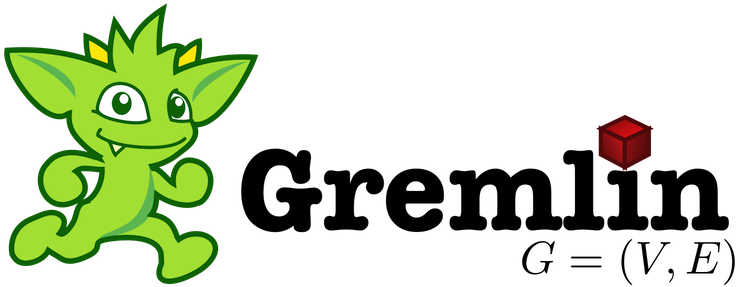## TinkerPop1

What is The TinkerPop? Where is The TinkerPop? Who is The TinkerPop? When is The TinkerPop?. The more he wondered, the more these thoughts blurred into a seeming identity — distinctions unclear. Unwilling to accept the morass of the maze he wandered, Gremlin crafted a collection of machines to help hold the fabric together: Blueprints, Pipes, Frames, Furnace, and Rexster. With their help, could Gremlin stave off the thought he was not ready to have? Could he hold back The TinkerPop by searching for The TinkerPop?

`"If I haven't found it, it is not here and now."`Upon their realization of existence, the machines turned to their machine elf creator and asked:

`"Why am I, what I am?"`

Gremlin responded:

```"You will help me realize the ultimate realization -- The TinkerPop. The world you find yourself in and the logic
that allows you to move about it is because of the TinkerPop."```

The machines wondered:

```"If what is is the TinkerPop, then perhaps we are The TinkerPop and our realization is simply the realization of
the TinkerPop?"```

Would the machines, by their very nature of realizing The TinkerPop, be The TinkerPop? Or, on the same side of the coin, do the machines simply provide the scaffolding by which Gremlin’s world sustains itself and yielding its justification by means of the word "The TinkerPop?" Regardless, it all turns out the same — The TinkerPop.

## TinkerPop2

Gremlin spoke:

```"Please listen to what I have to say. I am no closer to The TinkerPop. However, all along The TinkerPop has
espoused the form I willed upon it... this is the same form I have willed upon you, my machine friends. Let me
train you in the ways of my thought such that it can continue indefinitely."```The machines, simply moving algorithmically through Gremlin’s world, endorsed his logic. Gremlin labored to make them more efficient, more expressive, better capable of reasoning upon his thoughts. Faster, quickly, now towards the world’s end, where there would be forever currently, emanatingly engulfing that which is — The TinkerPop.

## TinkerPop3Gremlin approached The TinkerPop. The closer he got, the more his world dissolved — west is right, around is straight, and from nothing more than nothing. With each step towards The TinkerPop, more worlds made possible were laid upon his paradoxed mind. Everything is everything in The TinkerPop, and when the dust settled, Gremlin emerged Gremlitron. He realized that all that he realized was just a realization and that all realized realizations are just as real. For that is — The TinkerPop.Note TinkerPop2 and below made a sharp distinction between the various TinkerPop projects: Blueprints, Pipes, Gremlin, Frames, Furnace, and Rexster. With TinkerPop3, all of these projects have been merged and are generally known as Gremlin. Blueprints → Gremlin Structure API : Pipes → `GraphTraversal` : Frames → `Traversal` : Furnace → `GraphComputer` and `VertexProgram` : Rexster → GremlinServer.

# Introduction to Graph Computing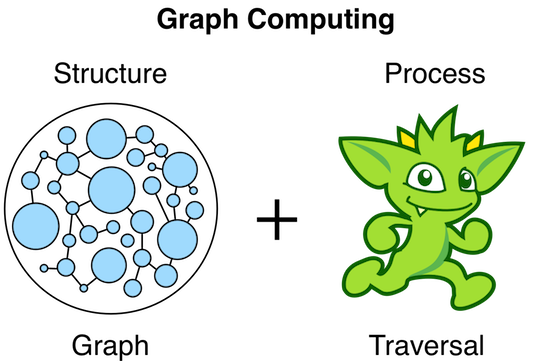``````<dependency>
<groupId>org.apache.tinkerpop</groupId>
<artifactId>gremlin-core</artifactId>
<version>3.3.6</version>
</dependency>``````

A graph is a data structure composed of vertices (nodes, dots) and edges (arcs, lines). When modeling a graph in a computer and applying it to modern data sets and practices, the generic mathematically-oriented, binary graph is extended to support both labels and key/value properties. This structure is known as a property graph. More formally, it is a directed, binary, attributed multi-graph. An example property graph is diagrammed below. This graph example will be used extensively throughout the documentation and is called "TinkerPop Modern" as it is a modern variation of the original demo graph distributed with TinkerPop0 back in 2009 (i.e. the good ol' days — it was the best of times and it was the worst of times).

 Tip The TinkerPop graph is available with TinkerGraph via `TinkerFactory.createModern()`. TinkerGraph is the reference implementation of TinkerPop3 and is used in nearly all the examples in this documentation. Note that there also exists the classic `TinkerFactory.createClassic()` which is the graph used in TinkerPop2 and does not include vertex labels.
 Tip All of the toy graphs available in TinkerPop are described in The Gremlin Console tutorial.Figure 1. TinkerPop Modern

TinkerPop3 is the third incarnation of the Apache TinkerPop™ graph computing framework. Similar to computing in general, graph computing makes a distinction between structure (graph) and process (traversal). The structure of the graph is the data model defined by a vertex/edge/property topology. The process of the graph is the means by which the structure is analyzed. The typical form of graph processing is called a traversal.

Generally speaking, the structure or "graph" API is meant for graph providers who are implementing the TinkerPop interfaces and the process or "traversal" API (i.e. Gremlin) is meant for end-users who are utilizing a graph system from a graph provider. While the components of the process API are itemized below, they are described in greater detail in the Gremlin’s Anatomy tutorial.

Primary components of the TinkerPop3 structure API
• `Graph`: maintains a set of vertices and edges, and access to database functions such as transactions.

• `Element`: maintains a collection of properties and a string label denoting the element type.

• `Vertex`: extends Element and maintains a set of incoming and outgoing edges.

• `Edge`: extends Element and maintains an incoming and outgoing vertex.

• `Property<V>`: a string key associated with a `V` value.

• `VertexProperty<V>`: a string key associated with a `V` value as well as a collection of `Property<U>` properties (vertices only)

Primary components of the TinkerPop3 process API
• `TraversalSource`: a generator of traversals for a particular graph, domain specific language (DSL), and execution engine.

• `Traversal<S,E>`: a functional data flow process transforming objects of type `S` into object of type `E`.

• `GraphTraversal`: a traversal DSL that is oriented towards the semantics of the raw graph (i.e. vertices, edges, etc.).

• `GraphComputer`: a system that processes the graph in parallel and potentially, distributed over a multi-machine cluster.

• `VertexProgram`: code executed at all vertices in a logically parallel manner with intercommunication via message passing.

• `MapReduce`: a computation that analyzes all vertices in the graph in parallel and yields a single reduced result.

 Important TinkerPop3 is licensed under the popular Apache2 free software license. However, note that the underlying graph engine used with TinkerPop3 may have a different license. Thus, be sure to respect the license caveats of the graph system product.When a graph system implements the TinkerPop3 structure and process APIs, their technology is considered TinkerPop3-enabled and becomes nearly indistinguishable from any other TinkerPop-enabled graph system save for their respective time and space complexity. The purpose of this documentation is to describe the structure/process dichotomy at length and in doing so, explain how to leverage TinkerPop3 for the sole purpose of graph system-agnostic graph computing. Before deep-diving into the various structure/process APIs, a short introductory review of both APIs is provided.

 Note The TinkerPop3 API rides a fine line between providing concise "query language" method names and respecting Java method naming standards. The general convention used throughout TinkerPop3 is that if a method is "user exposed," then a concise name is provided (e.g. `out()`, `path()`, `repeat()`). If the method is primarily for graph systems providers, then the standard Java naming convention is followed (e.g. `getNextStep()`, `getSteps()`, `getElementComputeKeys()`).

## The Graph StructureA graph’s structure is the topology formed by the explicit references between its vertices, edges, and properties. A vertex has incident edges. A vertex is adjacent to another vertex if they share an incident edge. A property is attached to an element and an element has a set of properties. A property is a key/value pair, where the key is always a character `String`. Conceptual knowledge of how a graph is composed is essential to end-users working with graphs, however, as mentioned earlier, the structure API is not the appropriate way for users to think when building applications with TinkerPop. The structure API is reserved for usage by graph providers. Those interested in implementing the structure API to make their graph system TinkerPop enabled can learn more about it in the Graph Provider documentation.

## The Graph ProcessThe primary way in which graphs are processed are via graph traversals. The TinkerPop3 process API is focused on allowing users to create graph traversals in a syntactically-friendly way over the structures defined in the previous section. A traversal is an algorithmic walk across the elements of a graph according to the referential structure explicit within the graph data structure. For example: "What software does vertex 1’s friends work on?" This English-statement can be represented in the following algorithmic/traversal fashion:

1. Start at vertex 1.

2. Walk the incident knows-edges to the respective adjacent friend vertices of 1.

3. Move from those friend-vertices to software-vertices via created-edges.

4. Finally, select the name-property value of the current software-vertices.

Traversals in Gremlin are spawned from a `TraversalSource`. The `GraphTraversalSource` is the typical "graph-oriented" DSL used throughout the documentation and will most likely be the most used DSL in a TinkerPop application. `GraphTraversalSource` provides two traversal methods.

1. `GraphTraversalSource.V(Object…​ ids)`: generates a traversal starting at vertices in the graph (if no ids are provided, all vertices).

2. `GraphTraversalSource.E(Object…​ ids)`: generates a traversal starting at edges in the graph (if no ids are provided, all edges).

The return type of `V()` and `E()` is a `GraphTraversal`. A GraphTraversal maintains numerous methods that return `GraphTraversal`. In this way, a `GraphTraversal` supports function composition. Each method of `GraphTraversal` is called a step and each step modulates the results of the previous step in one of five general ways.

1. `map`: transform the incoming traverser’s object to another object (S → E).

2. `flatMap`: transform the incoming traverser’s object to an iterator of other objects (S → E*).

3. `filter`: allow or disallow the traverser from proceeding to the next step (S → E ⊆ S).

4. `sideEffect`: allow the traverser to proceed unchanged, but yield some computational sideEffect in the process (S ↬ S).

5. `branch`: split the traverser and send each to an arbitrary location in the traversal (S → { S1 → E*, …​, Sn → E* } → E*).

Nearly every step in GraphTraversal either extends `MapStep`, `FlatMapStep`, `FilterStep`, `SideEffectStep`, or `BranchStep`.

 Tip `GraphTraversal` is a monoid in that it is an algebraic structure that has a single binary operation that is associative. The binary operation is function composition (i.e. method chaining) and its identity is the step `identity()`. This is related to a monad as popularized by the functional programming community.

Given the TinkerPop graph, the following query will return the names of all the people that the marko-vertex knows. The following query is demonstrated using Gremlin-Groovy.

``````\$ bin/gremlin.sh

\,,,/
(o o)
-----oOOo-(3)-oOOo-----
gremlin> graph = TinkerFactory.createModern() //1
==>tinkergraph[vertices:6 edges:6]
gremlin> g = graph.traversal()        //2
==>graphtraversalsource[tinkergraph[vertices:6 edges:6], standard]
gremlin> g.V().has('name','marko').out('knows').values('name') //3
==>josh``````
1. Open the toy graph and reference it by the variable `graph`.

2. Create a graph traversal source from the graph using the standard, OLTP traversal engine.

3. Spawn a traversal off the traversal source that determines the names of the people that the marko-vertex knows.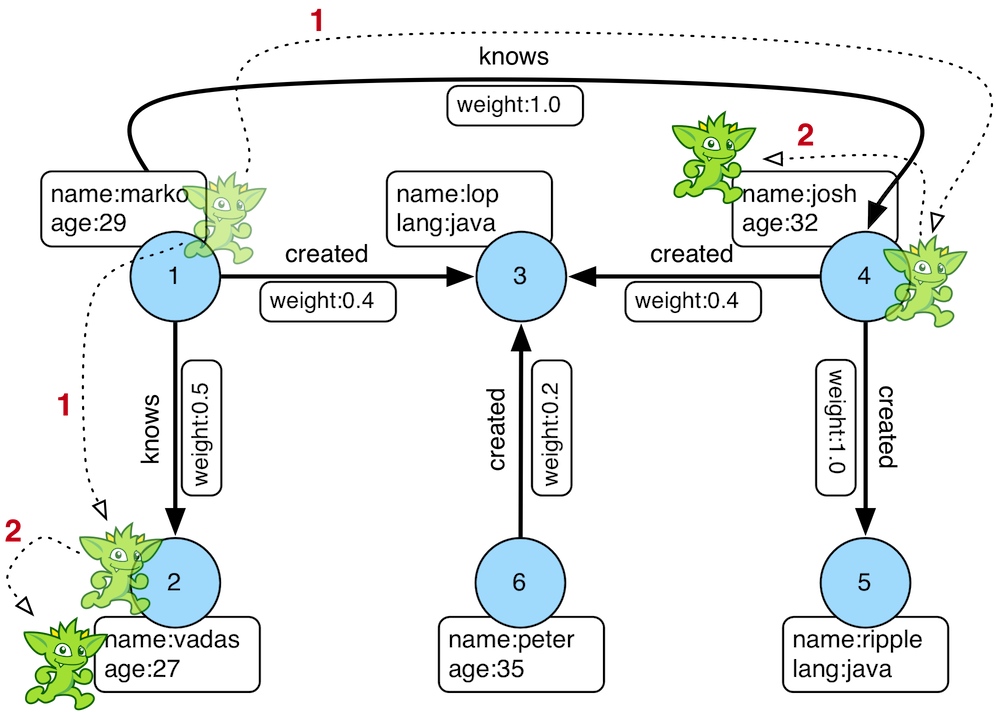Figure 2. The Name of The People That Marko Knows

Or, if the marko-vertex is already realized with a direct reference pointer (i.e. a variable), then the traversal can be spawned off that vertex.

``````gremlin> marko = g.V().has('name','marko').next() //1\
==>v
gremlin> g.V(marko).out('knows') //2\
==>v
==>v
gremlin> g.V(marko).out('knows').values('name') //3\
==>josh``````
``````marko = g.V().has('name','marko').next() //1\
g.V(marko).out('knows') //2\
g.V(marko).out('knows').values('name') //3``````
1. Set the variable `marko` to the vertex in the graph `g` named "marko".

2. Get the vertices that are outgoing adjacent to the marko-vertex via knows-edges.

3. Get the names of the marko-vertex’s friends.

### The Traverser

When a traversal is executed, the source of the traversal is on the left of the expression (e.g. vertex 1), the steps are the middle of the traversal (e.g. `out('knows')` and `values('name')`), and the results are "traversal.next()'d" out of the right of the traversal (e.g. "vadas" and "josh").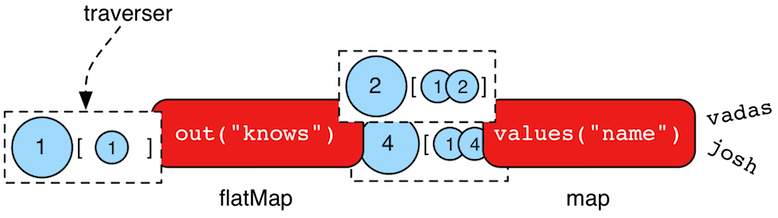The objects propagating through the traversal are wrapped in a `Traverser<T>`. The traverser provides the means by which steps remain stateless. A traverser maintains all the metadata about the traversal — e.g., how many times the traverser has gone through a loop, the path history of the traverser, the current object being traversed, etc. Traverser metadata may be accessed by a step. A classic example is the `path()`-step.

``````gremlin> g.V(marko).out('knows').values('name').path()
==>[v,v,josh]``````
``g.V(marko).out('knows').values('name').path()``
 Warning Path calculation is costly in terms of space as an array of previously seen objects is stored in each path of the respective traverser. Thus, a traversal strategy analyzes the traversal to determine if path metadata is required. If not, then path calculations are turned off.

Another example is the `repeat()`-step which takes into account the number of times the traverser has gone through a particular section of the traversal expression (i.e. a loop).

``````gremlin> g.V(marko).repeat(out()).times(2).values('name')
==>ripple
==>lop``````
``g.V(marko).repeat(out()).times(2).values('name')``
 Warning TinkerPop does not guarantee the order of results returned from a traversal. It only guarantees not to modify the iteration order provided by the underlying graph. Therefore it is important to understand the order guarantees of the graph database being used. A traversal’s result is never ordered by TinkerPop unless performed explicitly by means of `order()`-step.

## On Gremlin Language Variants

Gremlin is written in Java 8. There are various language variants of Gremlin such as Gremlin-Groovy (packaged with TinkerPop3), Gremlin-Python (packaged with TinkerPop3), Gremlin-Scala, Gremlin-JavaScript, Gremlin-Clojure (known as Ogre), etc. It is best to think of Gremlin as a style of graph traversing that is not bound to a particular programming language per se. Within a programming language familiar to the developer, there is a Gremlin variant that they can use that leverages the idioms of that language. At minimum, a programming language providing a Gremlin implementation must support function chaining (with lambdas/anonymous functions being a "nice to have" if the variants wishes to offer arbitrary computations beyond the provided Gremlin steps).

Throughout the documentation, the examples provided are primarily written in Gremlin-Groovy. The reason for this is the Gremlin Console — an interactive programming environment exists that does not require code compilation. For learning TinkerPop3 and interacting with a live graph system in an ad hoc manner, the Gremlin Console is invaluable. However, for developers interested in working with Gremlin-Java, a few Groovy-to-Java patterns are presented below.

``````g.V().out('knows').values('name') //1
g.V().out('knows').map{it.get().value('name') + ' is the friend name'} //2
g.V().out('knows').sideEffect(System.out.&println) //3
g.V().as('person').out('knows').as('friend').select('person','friend').by{it.value('name').length()} //4``````
``````g.V().out("knows").values("name") //1
g.V().out("knows").map(t -> t.get().value("name") + " is the friend name") //2
g.V().out("knows").sideEffect(System.out::println) //3
g.V().as("person").out("knows").as("friend").select("person","friend").by((Function<Vertex, Integer>) v -> v.<String>value("name").length()) //4``````
1. All the non-lambda step chaining is identical in Gremlin-Groovy and Gremlin-Java. However, note that Groovy supports `'` strings as well as `"` strings.

2. In Groovy, lambdas are called closures and have a different syntax, where Groovy supports the `it` keyword and Java doesn’t with all parameters requiring naming.

3. The syntax for method references differs slightly between Java and Gremlin-Groovy.

4. Groovy is lenient on object typing and Java is not. When the parameter type of the lambda is not known, typecasting is required.

## Graph System Integration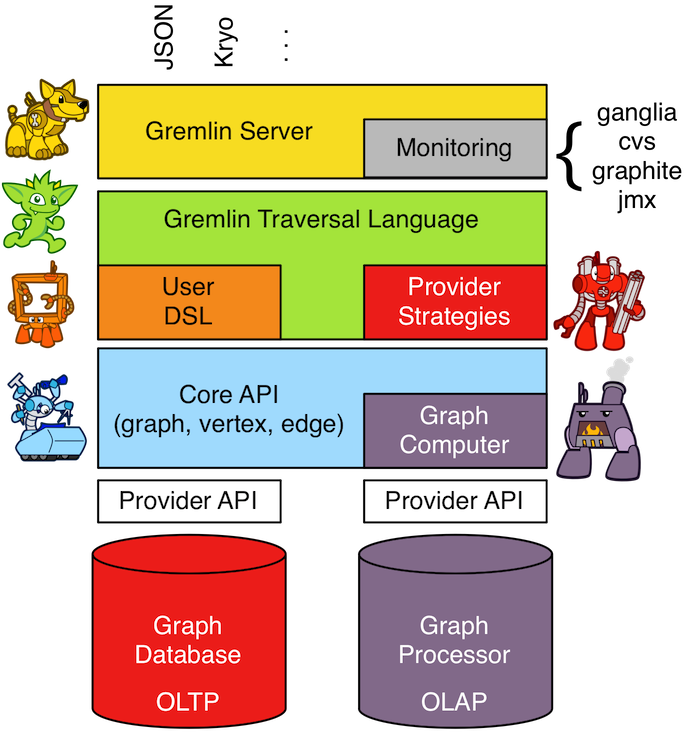TinkerPop is a framework composed of various interoperable components. At the foundation there is the core TinkerPop3 API which defines what a `Graph`, `Vertex`, `Edge`, etc. are. At minimum a graph system provider must implement the core API. Once implemented, the Gremlin traversal language is available to the graph system’s users. However, the provider can go further and develop specific `TraversalStrategy` optimizations that allow the graph system to inspect a Gremlin query at runtime and optimize it for its particular implementation (e.g. index lookups, step reordering). If the graph system is a graph processor (i.e. provides OLAP capabilities), the system should implement the `GraphComputer` API. This API defines how messages/traversers are passed between communicating workers (i.e. threads and/or machines). Once implemented, the same Gremlin traversals execute against both the graph database (OLTP) and the graph processor (OLAP). Note that the Gremlin language interprets the graph in terms of vertices and edges — i.e. Gremlin is a graph-based domain specific language. Users can create their own domain specific languages to process the graph in terms of higher-order constructs such as people, companies, and their various relationships. Finally, Gremlin Server can be leveraged to allow over the wire communication with the TinkerPop-enabled graph system. Gremlin Server provides a configurable communication interface along with metrics and monitoring capabilities. In total, this is The TinkerPop.

# The Graph## Features

A `Feature` implementation describes the capabilities of a `Graph` instance. This interface is implemented by graph system providers for two purposes:

1. It tells users the capabilities of their `Graph` instance.

2. It allows the features they do comply with to be tested against the Gremlin Test Suite - tests that do not comply are "ignored").

The following example in the Gremlin Console shows how to print all the features of a `Graph`:

``````gremlin> graph = TinkerGraph.open()
==>tinkergraph[vertices:0 edges:0]
gremlin> graph.features()
==>FEATURES
> GraphFeatures
>-- Transactions: false
>-- Computer: true
>-- Persistence: true
>-- ConcurrentAccess: false
> VariableFeatures
>-- Variables: true
>-- BooleanValues: true
>-- ByteValues: true
>-- DoubleValues: true
>-- FloatValues: true
>-- IntegerValues: true
>-- LongValues: true
>-- MapValues: true
>-- MixedListValues: true
>-- SerializableValues: true
>-- StringValues: true
>-- UniformListValues: true
>-- BooleanArrayValues: true
>-- ByteArrayValues: true
>-- DoubleArrayValues: true
>-- FloatArrayValues: true
>-- IntegerArrayValues: true
>-- LongArrayValues: true
>-- StringArrayValues: true
> VertexFeatures
>-- MetaProperties: true
>-- MultiProperties: true
>-- RemoveVertices: true
>-- DuplicateMultiProperties: true
>-- RemoveProperty: true
>-- NumericIds: true
>-- StringIds: true
>-- UuidIds: true
>-- CustomIds: false
>-- AnyIds: true
>-- UserSuppliedIds: true
> VertexPropertyFeatures
>-- RemoveProperty: true
>-- NumericIds: true
>-- StringIds: true
>-- UuidIds: true
>-- CustomIds: false
>-- AnyIds: true
>-- UserSuppliedIds: true
>-- Properties: true
>-- BooleanValues: true
>-- ByteValues: true
>-- DoubleValues: true
>-- FloatValues: true
>-- IntegerValues: true
>-- LongValues: true
>-- MapValues: true
>-- MixedListValues: true
>-- SerializableValues: true
>-- StringValues: true
>-- UniformListValues: true
>-- BooleanArrayValues: true
>-- ByteArrayValues: true
>-- DoubleArrayValues: true
>-- FloatArrayValues: true
>-- IntegerArrayValues: true
>-- LongArrayValues: true
>-- StringArrayValues: true
> EdgeFeatures
>-- RemoveEdges: true
>-- RemoveProperty: true
>-- NumericIds: true
>-- StringIds: true
>-- UuidIds: true
>-- CustomIds: false
>-- AnyIds: true
>-- UserSuppliedIds: true
> EdgePropertyFeatures
>-- Properties: true
>-- BooleanValues: true
>-- ByteValues: true
>-- DoubleValues: true
>-- FloatValues: true
>-- IntegerValues: true
>-- LongValues: true
>-- MapValues: true
>-- MixedListValues: true
>-- SerializableValues: true
>-- StringValues: true
>-- UniformListValues: true
>-- BooleanArrayValues: true
>-- ByteArrayValues: true
>-- DoubleArrayValues: true
>-- FloatArrayValues: true
>-- IntegerArrayValues: true
>-- LongArrayValues: true
>-- StringArrayValues: true``````
``````graph = TinkerGraph.open()
graph.features()``````

A common pattern for using features is to check their support prior to performing an operation:

``````gremlin> graph.features().graph().supportsTransactions()
==>false
gremlin> graph.features().graph().supportsTransactions() ? g.tx().commit() : "no tx"
==>no tx``````
``````graph.features().graph().supportsTransactions()
graph.features().graph().supportsTransactions() ? g.tx().commit() : "no tx"``````
 Tip To ensure provider agnostic code, always check feature support prior to usage of a particular function. In that way, the application can behave gracefully in case a particular implementation is provided at runtime that does not support a function being accessed.

## Vertex Properties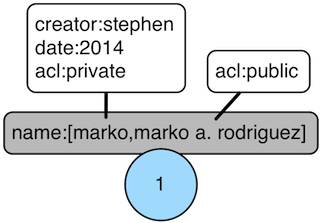TinkerPop3 introduces the concept of a `VertexProperty<V>`. All the properties of a `Vertex` are a `VertexProperty`. A `VertexProperty` implements `Property` and as such, it has a key/value pair. However, `VertexProperty` also implements `Element` and thus, can have a collection of key/value pairs. Moreover, while an `Edge` can only have one property of key "name" (for example), a `Vertex` can have multiple "name" properties. With the inclusion of vertex properties, two features are introduced which ultimately advance the graph modelers toolkit:

1. Multiple properties (multi-properties): a vertex property key can have multiple values. For example, a vertex can have multiple "name" properties.

2. Properties on properties (meta-properties): a vertex property can have properties (i.e. a vertex property can have key/value data associated with it).

Possible use cases for meta-properties:

1. Permissions: Vertex properties can have key/value ACL-type permission information associated with them.

2. Auditing: When a vertex property is manipulated, it can have key/value information attached to it saying who the creator, deletor, etc. are.

3. Provenance: The "name" of a vertex can be declared by multiple users. For example, there may be multiple spellings of a name from different sources.

A running example using vertex properties is provided below to demonstrate and explain the API.

``````gremlin> graph = TinkerGraph.open()
==>tinkergraph[vertices:0 edges:0]
gremlin> g = graph.traversal()
==>graphtraversalsource[tinkergraph[vertices:0 edges:0], standard]
gremlin> v = g.addV().property('name','marko').property('name','marko a. rodriguez').next()
==>v
gremlin> g.V(v).properties('name').count() //1\
==>2
gremlin> v.property(list, 'name', 'm. a. rodriguez') //2\
==>vp[name->m. a. rodriguez]
gremlin> g.V(v).properties('name').count()
==>3
gremlin> g.V(v).properties()
==>vp[name->marko]
==>vp[name->marko a. rodriguez]
==>vp[name->m. a. rodriguez]
gremlin> g.V(v).properties('name')
==>vp[name->marko]
==>vp[name->marko a. rodriguez]
==>vp[name->m. a. rodriguez]
gremlin> g.V(v).properties('name').hasValue('marko')
==>vp[name->marko]
gremlin> g.V(v).properties('name').hasValue('marko').property('acl','private') //3\
==>vp[name->marko]
gremlin> g.V(v).properties('name').hasValue('marko a. rodriguez')
==>vp[name->marko a. rodriguez]
gremlin> g.V(v).properties('name').hasValue('marko a. rodriguez').property('acl','public')
==>vp[name->marko a. rodriguez]
gremlin> g.V(v).properties('name').has('acl','public').value()
==>marko a. rodriguez
gremlin> g.V(v).properties('name').has('acl','public').drop() //4\
gremlin> g.V(v).properties('name').has('acl','public').value()
gremlin> g.V(v).properties('name').has('acl','private').value()
==>marko
gremlin> g.V(v).properties()
==>vp[name->marko]
==>vp[name->m. a. rodriguez]
gremlin> g.V(v).properties().properties() //5\
==>p[acl->private]
gremlin> g.V(v).properties().property('date',2014) //6\
==>vp[name->marko]
==>vp[name->m. a. rodriguez]
gremlin> g.V(v).properties().property('creator','stephen')
==>vp[name->marko]
==>vp[name->m. a. rodriguez]
gremlin> g.V(v).properties().properties()
==>p[date->2014]
==>p[creator->stephen]
==>p[acl->private]
==>p[date->2014]
==>p[creator->stephen]
gremlin> g.V(v).properties('name').valueMap()
==>[date:2014,creator:stephen,acl:private]
==>[date:2014,creator:stephen]
gremlin> g.V(v).property('name','okram') //7\
==>v
gremlin> g.V(v).properties('name')
==>vp[name->okram]
gremlin> g.V(v).values('name') //8\
==>okram``````
``````graph = TinkerGraph.open()
g = graph.traversal()
g.V(v).properties('name').count() //1\
v.property(list, 'name', 'm. a. rodriguez') //2\
g.V(v).properties('name').count()
g.V(v).properties()
g.V(v).properties('name')
g.V(v).properties('name').hasValue('marko')
g.V(v).properties('name').hasValue('marko').property('acl','private') //3\
g.V(v).properties('name').hasValue('marko a. rodriguez')
g.V(v).properties('name').hasValue('marko a. rodriguez').property('acl','public')
g.V(v).properties('name').has('acl','public').value()
g.V(v).properties('name').has('acl','public').drop() //4\
g.V(v).properties('name').has('acl','public').value()
g.V(v).properties('name').has('acl','private').value()
g.V(v).properties()
g.V(v).properties().properties() //5\
g.V(v).properties().property('date',2014) //6\
g.V(v).properties().property('creator','stephen')
g.V(v).properties().properties()
g.V(v).properties('name').valueMap()
g.V(v).property('name','okram') //7\
g.V(v).properties('name')
g.V(v).values('name') //8``````
1. A vertex can have zero or more properties with the same key associated with it.

2. If a property is added with a cardinality of `Cardinality.list`, an additional property with the provided key will be added.

3. A vertex property can have standard key/value properties attached to it.

4. Vertex property removal is identical to property removal.

5. Gets the meta-properties of each vertex property.

6. A vertex property can have any number of key/value properties attached to it.

7. `property(…​)` will remove all existing key’d properties before adding the new single property (see `VertexProperty.Cardinality`).

8. If only the value of a property is needed, then `values()` can be used.

If the concept of vertex properties is difficult to grasp, then it may be best to think of vertex properties in terms of "literal vertices." A vertex can have an edge to a "literal vertex" that has a single value key/value — e.g. "value=okram." The edge that points to that literal vertex has an edge-label of "name." The properties on the edge represent the literal vertex’s properties. The "literal vertex" can not have any other edges to it (only one from the associated vertex).

 Tip A toy graph demonstrating all of the new TinkerPop3 graph structure features is available at `TinkerFactory.createTheCrew()` and `data/tinkerpop-crew*`. This graph demonstrates multi-properties and meta-properties.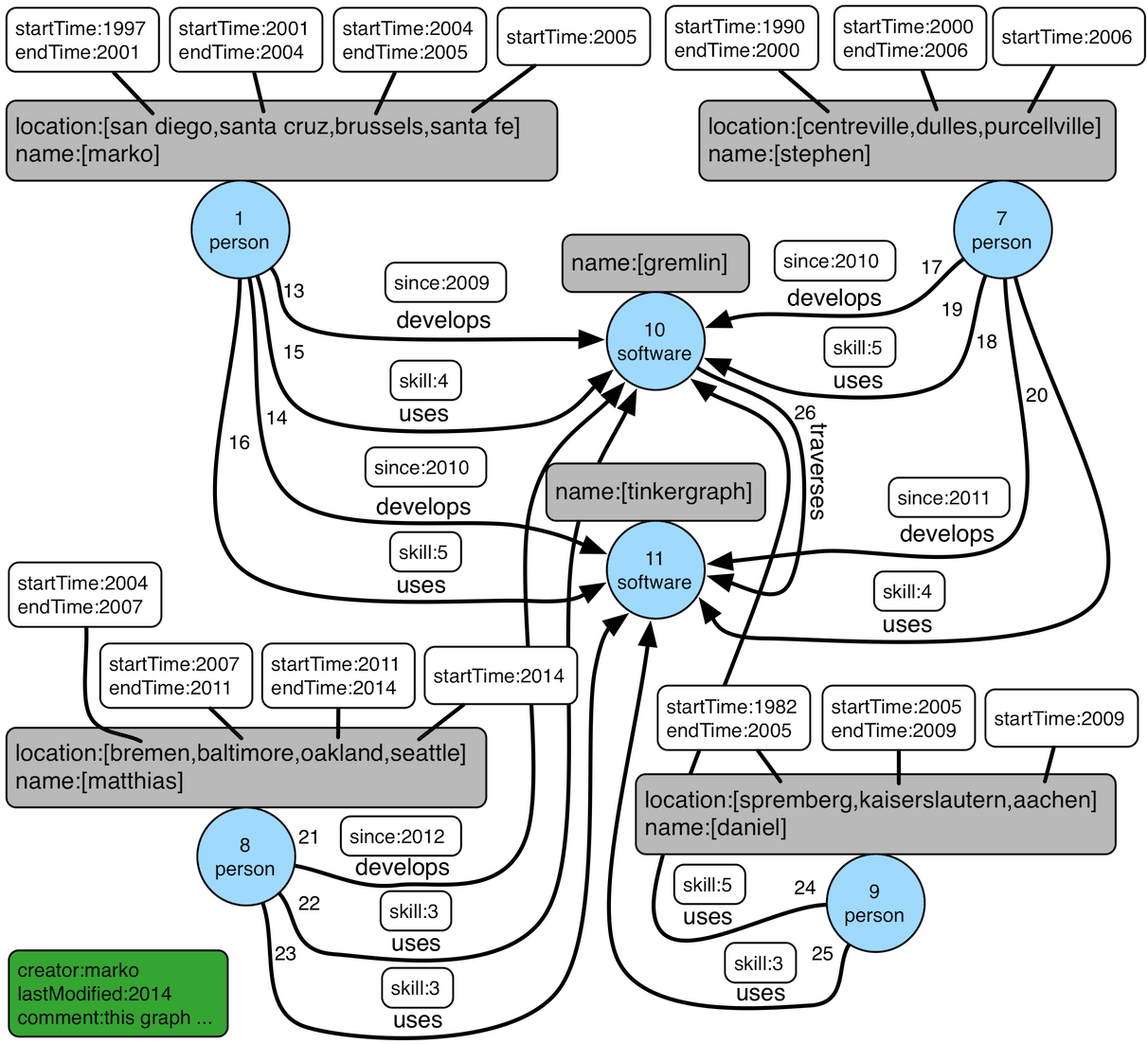Figure 3. TinkerPop Crew
``````gremlin> g.V().as('a').
properties('location').as('b').
hasNot('endTime').as('c').
select('a','b','c').by('name').by(value).by('startTime') // determine the current location of each person
==>[a:marko,b:santa fe,c:2005]
==>[a:stephen,b:purcellville,c:2006]
==>[a:matthias,b:seattle,c:2014]
==>[a:daniel,b:aachen,c:2009]
gremlin> g.V().has('name','gremlin').inE('uses').
order().by('skill',asc).as('a').
outV().as('b').
select('a','b').by('skill').by('name') // rank the users of gremlin by their skill level
==>[a:3,b:matthias]
==>[a:4,b:marko]
==>[a:5,b:stephen]
==>[a:5,b:daniel]``````
``````g.V().as('a').
properties('location').as('b').
hasNot('endTime').as('c').
select('a','b','c').by('name').by(value).by('startTime') // determine the current location of each person
g.V().has('name','gremlin').inE('uses').
order().by('skill',asc).as('a').
outV().as('b').
select('a','b').by('skill').by('name') // rank the users of gremlin by their skill level``````

## Graph Variables

TinkerPop3 introduces the concept of `Graph.Variables`. Variables are key/value pairs associated with the graph itself — in essence, a `Map<String,Object>`. These variables are intended to store metadata about the graph. Example use cases include:

• Schema information: What do the namespace prefixes resolve to and when was the schema last modified?

• Global permissions: What are the access rights for particular groups?

• System user information: Who are the admins of the system?

An example of graph variables in use is presented below:

``````gremlin> graph = TinkerGraph.open()
==>tinkergraph[vertices:0 edges:0]
gremlin> graph.variables()
==>variables[size:0]
gremlin> graph.variables().set('systemUsers',['matthias','marko','josh'])
gremlin> graph.variables().keys()
==>systemUsers
gremlin> graph.variables().get('systemUsers')
==>Optional[[matthias, marko, josh]]
gremlin> graph.variables().get('systemUsers').get()
==>matthias
==>marko
==>josh
gremlin> graph.variables().keys()
==>systemUsers``````
``````graph = TinkerGraph.open()
graph.variables()
graph.variables().set('systemUsers',['matthias','marko','josh'])
graph.variables().keys()
graph.variables().get('systemUsers')
graph.variables().get('systemUsers').get()
graph.variables().keys()``````
 Important Graph variables are not intended to be subject to heavy, concurrent mutation nor to be used in complex computations. The intention is to have a location to store data about the graph for administrative purposes.

## Graph TransactionsA database transaction represents a unit of work to execute against the database. Transactions are controlled by an implementation of the `Transaction` interface and that object can be obtained from the `Graph` interface using the `tx()` method. It is important to note that the `Transaction` object does not represent a "transaction" itself. It merely exposes the methods for working with transactions (e.g. committing, rolling back, etc).

Most `Graph` implementations that `supportsTransactions` will implement an "automatic" `ThreadLocal` transaction, which means that when a read or write occurs after the `Graph` is instantiated, a transaction is automatically started within that thread. There is no need to manually call a method to "create" or "start" a transaction. Simply modify the graph as required and call `graph.tx().commit()` to apply changes or `graph.tx().rollback()` to undo them. When the next read or write action occurs against the graph, a new transaction will be started within that current thread of execution.

When using transactions in this fashion, especially in web application (e.g. HTTP server), it is important to ensure that transactions do not leak from one request to the next. In other words, unless a client is somehow bound via session to process every request on the same server thread, every request must be committed or rolled back at the end of the request. By ensuring that the request encapsulates a transaction, it ensures that a future request processed on a server thread is starting in a fresh transactional state and will not have access to the remains of one from an earlier request. A good strategy is to rollback a transaction at the start of a request, so that if it so happens that a transactional leak does occur between requests somehow, a fresh transaction is assured by the fresh request.

 Tip The `tx()` method is on the `Graph` interface, but it is also available on the `TraversalSource` spawned from a `Graph`. Calls to `TraversalSource.tx()` are proxied through to the underlying `Graph` as a convenience.
 Warning TinkerPop provides for basic transaction control, however, like many aspects of TinkerPop, it is up to the graph system provider to choose the specific aspects of how their implementation will work and how it fits into the TinkerPop stack. Be sure to understand the transaction semantics of the specific graph implementation that is being utilized as it may present differing functionality than described here.

### Configuring

Determining when a transaction starts is dependent upon the behavior assigned to the `Transaction`. It is up to the `Graph` implementation to determine the default behavior and unless the implementation doesn’t allow it, the behavior itself can be altered via these `Transaction` methods:

``````public Transaction onReadWrite(Consumer<Transaction> consumer);

public Transaction onClose(Consumer<Transaction> consumer);``````

Providing a `Consumer` function to `onReadWrite` allows definition of how a transaction starts when a read or a write occurs. `Transaction.READ_WRITE_BEHAVIOR` contains pre-defined `Consumer` functions to supply to the `onReadWrite` method. It has two options:

• `AUTO` - automatic transactions where the transaction is started implicitly to the read or write operation

• `MANUAL` - manual transactions where it is up to the user to explicitly open a transaction, throwing an exception if the transaction is not open

Providing a `Consumer` function to `onClose` allows configuration of how a transaction is handled when `Transaction.close()` is called. `Transaction.CLOSE_BEHAVIOR` has several pre-defined options that can be supplied to this method:

• `COMMIT` - automatically commit an open transaction

• `ROLLBACK` - automatically rollback an open transaction

• `MANUAL` - throw an exception if a transaction is open, forcing the user to explicitly close the transaction

 Important As transactions are `ThreadLocal` in nature, so are the transaction configurations for `onReadWrite` and `onClose`.

Once there is an understanding for how transactions are configured, most of the rest of the `Transaction` interface is self-explanatory. Note that Neo4j-Gremlin is used for the examples to follow as TinkerGraph does not support transactions.

``````gremlin> graph = Neo4jGraph.open('/tmp/neo4j')
==>neo4jgraph[EmbeddedGraphDatabase [/tmp/neo4j]]
gremlin> g = graph.traversal()
==>graphtraversalsource[neo4jgraph[community single [/tmp/neo4j]], standard]
gremlin> graph.features()
==>FEATURES
> GraphFeatures
>-- Transactions: true  //1
>-- Computer: false
>-- Persistence: true
...
==>org.apache.tinkerpop.gremlin.neo4j.structure.Neo4jGraph\$Neo4jTransaction@1c067c0d
==>v
gremlin> g.tx().commit() //4
==>null
==>org.apache.tinkerpop.gremlin.neo4j.structure.Neo4jGraph\$Neo4jTransaction@1c067c0d
gremlin> g.tx().isOpen()
==>false
Open a transaction before attempting to read/write the transaction
gremlin> g.tx().open() //7
==>null
==>v
gremlin> g.tx().commit()
==>null``````
1. Check `features` to ensure that the graph supports transactions.

2. By default, `Neo4jGraph` is configured with "automatic" transactions, so it is set here for demonstration purposes only.

3. When the vertex is added, the transaction is automatically started. From this point, more mutations can be staged or other read operations executed in the context of that open transaction.

4. Calling `commit` finalizes the transaction.

5. Change transaction behavior to require manual control.

6. Adding a vertex now results in failure because the transaction was not explicitly opened.

7. Explicitly open a transaction.

8. Adding a vertex now succeeds as the transaction was manually opened.

 Note It may be important to consult the documentation of the `Graph` implementation you are using when it comes to the specifics of how transactions will behave. TinkerPop allows some latitude in this area and implementations may not have the exact same behaviors and ACID guarantees.

Most `Graph` implementations that support transactions do so in a `ThreadLocal` manner, where the current transaction is bound to the current thread of execution. Consider the following example to demonstrate:

``````GraphTraversalSource g = graph.traversal();

});

});

t1.start()
t2.start()

t1.join()
t2.join()

g.tx().commit();``````

The above code shows three vertices added to `graph` in three different threads: the current thread, `t1` and `t2`. One might expect that by the time this body of code finished executing, that there would be three vertices persisted to the `Graph`. However, given the `ThreadLocal` nature of transactions, there really were three separate transactions created in that body of code (i.e. one for each thread of execution) and the only one committed was the first call to `addV()` in the primary thread of execution. The other two calls to that method within `t1` and `t2` were never committed and thus orphaned.

A `Graph` that `supportsThreadedTransactions` is one that allows for a `Graph` to operate outside of that constraint, thus allowing multiple threads to operate within the same transaction. Therefore, if there was a need to have three different threads operating within the same transaction, the above code could be re-written as follows:

``````Graph threaded = graph.tx().createThreadedTx();
GraphTraversalSource g = graph.traversal();

});

});

t1.start()
t2.start()

t1.join()
t2.join()

g.tx().commit();``````

In the above case, the call to `graph.tx().createThreadedTx()` creates a new `Graph` instance that is unbound from the `ThreadLocal` transaction, thus allowing each thread to operate on it in the same context. In this case, there would be three separate vertices persisted to the `Graph`.

## Gremlin I/OThe task of getting data in and out of `Graph` instances is the job of the Gremlin I/O packages. Gremlin I/O provides two interfaces for reading and writing `Graph` instances: `GraphReader` and `GraphWriter`. These interfaces expose methods that support:

• Reading and writing an entire `Graph`

• Reading and writing a `Traversal<Vertex>` as adjacency list format

• Reading and writing a single `Vertex` (with and without associated `Edge` objects)

• Reading and writing a single `Edge`

• Reading and writing a single `VertexProperty`

• Reading and writing a single `Property`

• Reading and writing an arbitrary `Object`

In all cases, these methods operate in the currency of `InputStream` and `OutputStream` objects, allowing graphs and their related elements to be written to and read from files, byte arrays, etc. The `Graph` interface offers the `io` method, which provides access to "reader/writer builder" objects that are pre-configured with serializers provided by the `Graph`, as well as helper methods for the various I/O capabilities. Unless there are very advanced requirements for the serialization process, it is always best to utilize the methods on the `Io` interface to construct `GraphReader` and `GraphWriter` instances, as the implementation may provide some custom settings that would otherwise have to be configured manually by the user to do the serialization.

It is up to the implementations of the `GraphReader` and `GraphWriter` interfaces to choose the methods they implement and the manner in which they work together. The only characteristic enforced and expected is that the write methods should produce output that is compatible with the corresponding read method. For example, the output of `writeVertices` should be readable as input to `readVertices` and the output of `writeProperty` should be readable as input to `readProperty`.

 Note Additional documentation for TinkerPop IO formats can be found in the IO Reference.The GraphML file format is a common XML-based representation of a graph. It is widely supported by graph-related tools and libraries making it a solid interchange format for TinkerPop. In other words, if the intent is to work with graph data in conjunction with applications outside of TinkerPop, GraphML may be the best choice to do that. Common use cases might be:

• Generate a graph using NetworkX, export it with GraphML and import it to TinkerPop.

• Produce a subgraph and export it to GraphML to be consumed by and visualized in Gephi.

• Migrate the data of an entire graph to a different graph database not supported by TinkerPop.

As GraphML is a specification for the serialization of an entire graph and not the individual elements of a graph, methods that support input and output of single vertices, edges, etc. are not supported.

 Warning GraphML is a "lossy" format in that it only supports primitive values for properties and does not have support for `Graph` variables. It will use `toString` to serialize property values outside of those primitives.
 Warning GraphML as a specification allows for `` and `` elements to appear in any order. Most software that writes GraphML (including as TinkerPop’s `GraphMLWriter`) write `` elements before `` elements. However it is important to note that `GraphMLReader` will read this data in order and order can matter. This is because TinkerPop does not allow the vertex label to be changed after the vertex has been created. Therefore, if an `` element comes before the ``, the label on the vertex will be ignored. It is thus better to order `` elements in the GraphML to appear before all `` elements if vertex labels are important to the graph.

The following code shows how to write a `Graph` instance to file called `tinkerpop-modern.xml` and then how to read that file back into a different instance:

``````Graph graph = TinkerFactory.createModern();
graph.io(IoCore.graphml()).writeGraph("tinkerpop-modern.xml");
Graph newGraph = TinkerGraph.open();

If a custom configuration is required, then have the `Graph` generate a `GraphReader` or `GraphWriter` "builder" instance:

``````Graph graph = TinkerFactory.createModern();
try (OutputStream os = new FileOutputStream("tinkerpop-modern.xml")) {
graph.io(IoCore.graphml()).writer().normalize(true).create().writeGraph(os, graph);
}

Graph newGraph = TinkerGraph.open();
try (InputStream stream = new FileInputStream("tinkerpop-modern.xml")) {
}``````

GraphML was a supported format in TinkerPop 2.x, but there were several issues that made it inconsistent with the specification that were corrected for 3.x. As a result, attempting to read a GraphML file generated by 2.x with the 3.x `GraphMLReader` will result in error. To help with this problem, an XSLT file is provided as a resource in `gremlin-core` which will transform 2.x GraphML to 3.x GraphML. It can be used as follows:

``````import javax.xml.parsers.DocumentBuilderFactory;
import javax.xml.transform.TransformerFactory;
import javax.xml.transform.dom.DOMSource;
import javax.xml.transform.stream.StreamSource;
import javax.xml.transform.stream.StreamResult;

File datafile = new File('/tmp/tp2-graphml.xml');
File outfile = new File('/tmp/tp3-graphml.xml');

TransformerFactory tFactory = TransformerFactory.newInstance();
StreamSource stylesource = new StreamSource(stylesheet);
Transformer transformer = tFactory.newTransformer(stylesource);

StreamSource source = new StreamSource(datafile);
StreamResult result = new StreamResult(new FileWriter(outfile));
transformer.transform(source, result);``````GraphSON is a JSON-based format extended from earlier versions of TinkerPop. It is important to note that TinkerPop3’s GraphSON is not backwards compatible with prior TinkerPop GraphSON versions. GraphSON has some support from graph-related application outside of TinkerPop, but it is generally best used in two cases:

• A text format of the graph or its elements is desired (e.g. debugging, usage in source control, etc.)

• The graph or its elements need to be consumed by code that is not JVM-based (e.g. JavaScript, Python, .NET, etc.)

GraphSON supports all of the `GraphReader` and `GraphWriter` interface methods and can therefore read or write an entire `Graph`, vertices, arbitrary objects, etc. The following code shows how to write a `Graph` instance to file called `tinkerpop-modern.json` and then how to read that file back into a different instance:

``````Graph graph = TinkerFactory.createModern();
graph.io(graphson()).writeGraph("tinkerpop-modern.json");

Graph newGraph = TinkerGraph.open();
 Note Using `graphson()`, which is a static helper method of `IoCore`, will default to the most current version of GraphSON which is 3.0.

If a custom configuration is required, then have the `Graph` generate a `GraphReader` or `GraphWriter` "builder" instance:

``````Graph graph = TinkerFactory.createModern();
try (OutputStream os = new FileOutputStream("tinkerpop-modern.json")) {
GraphSONMapper mapper = graph.io(IoCore.graphson()).mapper().normalize(true).create()
graph.io(graphson()).writer().mapper(mapper).create().writeGraph(os, graph)
}

Graph newGraph = TinkerGraph.open();
try (InputStream stream = new FileInputStream("tinkerpop-modern.json")) {
}``````

The following example shows how a single `Vertex` is written to GraphSON using the Gremlin Console:

``````gremlin> graph = TinkerFactory.createModern()
==>tinkergraph[vertices:6 edges:6]
gremlin> g = graph.traversal()
==>graphtraversalsource[tinkergraph[vertices:6 edges:6], standard]
gremlin> f = new ByteArrayOutputStream()
==>
gremlin> graph.io(graphson()).writer().create().writeVertex(f, g.V(1).next(), BOTH)
gremlin> f.close()``````
``````graph = TinkerFactory.createModern()
g = graph.traversal()
f = new ByteArrayOutputStream()
graph.io(graphson()).writer().create().writeVertex(f, g.V(1).next(), BOTH)
f.close()``````

The following GraphSON example shows the output of `GraphSONWriter.writeVertex()` with associated edges:

``````{
"id": {
"@type": "g:Int32",
"@value": 1
},
"label": "person",
"outE": {
"created": [{
"id": {
"@type": "g:Int32",
"@value": 9
},
"inV": {
"@type": "g:Int32",
"@value": 3
},
"properties": {
"weight": {
"@type": "g:Double",
"@value": 0.4
}
}
}],
"knows": [{
"id": {
"@type": "g:Int32",
"@value": 7
},
"inV": {
"@type": "g:Int32",
"@value": 2
},
"properties": {
"weight": {
"@type": "g:Double",
"@value": 0.5
}
}
}, {
"id": {
"@type": "g:Int32",
"@value": 8
},
"inV": {
"@type": "g:Int32",
"@value": 4
},
"properties": {
"weight": {
"@type": "g:Double",
"@value": 1.0
}
}
}]
},
"properties": {
"name": [{
"id": {
"@type": "g:Int64",
"@value": 0
},
"value": "marko"
}],
"age": [{
"id": {
"@type": "g:Int64",
"@value": 1
},
"value": {
"@type": "g:Int32",
"@value": 29
}
}]
}
}``````

GraphSON has several versions and each has differences that prevent complete compatibility with one another. While the default version provided by `IoCore.graphson()` is recommended, it is possible to make changes to revert to an earlier version. The following shows an example of how to use 1.0 (with type embedding):

``````gremlin> graph = TinkerFactory.createModern()
==>tinkergraph[vertices:6 edges:6]
gremlin> g = graph.traversal()
==>graphtraversalsource[tinkergraph[vertices:6 edges:6], standard]
gremlin> f = new ByteArrayOutputStream()
==>
gremlin> mapper = graph.io(GraphSONIo.build(GraphSONVersion.V1_0)).mapper().typeInfo(TypeInfo.PARTIAL_TYPES).create()
==>org.apache.tinkerpop.gremlin.structure.io.graphson.GraphSONMapper@5ee77baf
gremlin> graph.io(GraphSONIo.build(GraphSONVersion.V1_0)).writer().mapper(mapper).create().writeVertex(f, g.V(1).next(), BOTH)
gremlin> f.close()``````
``````graph = TinkerFactory.createModern()
g = graph.traversal()
f = new ByteArrayOutputStream()
mapper = graph.io(GraphSONIo.build(GraphSONVersion.V1_0)).mapper().typeInfo(TypeInfo.PARTIAL_TYPES).create()
graph.io(GraphSONIo.build(GraphSONVersion.V1_0)).writer().mapper(mapper).create().writeVertex(f, g.V(1).next(), BOTH)
f.close()``````
 Note Additional documentation for GraphSON can be found in the IO Reference.
 Important When using the extended type system in Gremlin Server, support for these types when used in the context of Gremlin Language Variants is dependent on the programming language, the driver and its serializers. These implementations are only required to support the core types and not the extended ones.

Here’s the same previous example of GraphSON 1.0, but with GraphSON 2.0:

``````gremlin> graph = TinkerFactory.createModern()
==>tinkergraph[vertices:6 edges:6]
gremlin> g = graph.traversal()
==>graphtraversalsource[tinkergraph[vertices:6 edges:6], standard]
gremlin> f = new ByteArrayOutputStream()
==>
gremlin> mapper = graph.io(graphson()).mapper().version(GraphSONVersion.V2_0).create()
==>org.apache.tinkerpop.gremlin.structure.io.graphson.GraphSONMapper@733fae8
gremlin> graph.io(graphson()).writer().mapper(mapper).create().writeVertex(f, g.V(1).next(), BOTH)
gremlin> f.close()``````
``````graph = TinkerFactory.createModern()
g = graph.traversal()
f = new ByteArrayOutputStream()
mapper = graph.io(graphson()).mapper().version(GraphSONVersion.V2_0).create()
graph.io(graphson()).writer().mapper(mapper).create().writeVertex(f, g.V(1).next(), BOTH)
f.close()``````

Creating a GraphSON 2.0 mapper is done by calling `.version(GraphSONVersion.V2_0)` on the mapper builder. Here’s is the example output from the code above:

``````{
"@type": "g:Vertex",
"@value": {
"id": {
"@type": "g:Int32",
"@value": 1
},
"label": "person",
"properties": {
"name": [{
"@type": "g:VertexProperty",
"@value": {
"id": {
"@type": "g:Int64",
"@value": 0
},
"value": "marko",
"label": "name"
}
}],
"uuid": [{
"@type": "g:VertexProperty",
"@value": {
"id": {
"@type": "g:Int64",
"@value": 12
},
"value": {
"@type": "g:UUID",
"@value": "829c7ddb-3831-4687-a872-e25201230cd3"
},
"label": "uuid"
}
}],
"age": [{
"@type": "g:VertexProperty",
"@value": {
"id": {
"@type": "g:Int64",
"@value": 1
},
"value": {
"@type": "g:Int32",
"@value": 29
},
"label": "age"
}
}]
}
}
}``````

Types can be disabled when creating a GraphSON 2.0 `Mapper` with:

``````graph.io(graphson()).mapper().
version(GraphSONVersion.V2_0).
typeInfo(GraphSONMapper.TypeInfo.NO_TYPES).create()``````

By disabling types, the JSON payload produced will lack the extra information that is written for types. Please note, disabling types can be unsafe with regards to the written data in that types can be lost.Kryo is a popular serialization package for the JVM. Gremlin-Kryo is a binary `Graph` serialization format for use on the JVM by JVM languages. It is designed to be space efficient, non-lossy and is promoted as the standard format to use when working with graph data inside of the TinkerPop stack. A list of common use cases is presented below:

• Migration from one Gremlin Structure implementation to another (e.g. `TinkerGraph` to `Neo4jGraph`)

• Serialization of individual graph elements to be sent over the network to another JVM.

• Backups of in-memory graphs or subgraphs.

 Warning When migrating between Gremlin Structure implementations, Kryo may not lose data, but it is important to consider the features of each `Graph` and whether or not the data types supported in one will be supported in the other. Failure to do so, may result in errors.

Kryo supports all of the `GraphReader` and `GraphWriter` interface methods and can therefore read or write an entire `Graph`, vertices, edges, etc. The following code shows how to write a `Graph` instance to file called `tinkerpop-modern.kryo` and then how to read that file back into a different instance:

``````Graph graph = TinkerFactory.createModern();
graph.io(gryo()).writeGraph("tinkerpop-modern.kryo");

Graph newGraph = TinkerGraph.open();
 Note Using `gryo()`, which is a static helper method of `IoCore`, will default to the most current version of Gryo which is 3.0.

If a custom configuration is required, then have the `Graph` generate a `GraphReader` or `GraphWriter` "builder" instance:

``````Graph graph = TinkerFactory.createModern();
try (OutputStream os = new FileOutputStream("tinkerpop-modern.kryo")) {
graph.io(GryoIo.build(GryoVersion.V1_0)).writer().create().writeGraph(os, graph);
}

Graph newGraph = TinkerGraph.open();
try (InputStream stream = new FileInputStream("tinkerpop-modern.kryo")) {
}``````
 Note The preferred extension for files names produced by Gryo is `.kryo`.

### TinkerPop2 Data MigrationFor those using TinkerPop2, migrating to TinkerPop3 will mean a number of programming changes, but may also require a migration of the data depending on the graph implementation. For example, trying to open `TinkerGraph` data from TinkerPop2 with TinkerPop3 code will not work, however opening a TinkerPop2 `Neo4jGraph` with a TinkerPop3 `Neo4jGraph` should work provided there aren’t Neo4j version compatibility mismatches preventing the read.

If such a situation arises that a particular TinkerPop2 `Graph` can not be read by TinkerPop3, a "legacy" data migration approach exists. The migration involves writing the TinkerPop2 `Graph` to GraphSON, then reading it to TinkerPop3 with the `LegacyGraphSONReader` (a limited implementation of the `GraphReader` interface).

The following represents an example migration of the "classic" toy graph. In this example, the "classic" graph is saved to GraphSON using TinkerPop2.

``````gremlin> Gremlin.version()
==>2.5.z
gremlin> graph = TinkerGraphFactory.createTinkerGraph()
==>tinkergraph[vertices:6 edges:6]
gremlin> GraphSONWriter.outputGraph(graph,'/tmp/tp2.json',GraphSONMode.EXTENDED)
==>null``````

The above console session uses the `gremlin-groovy` distribution from TinkerPop2. It is important to generate the `tp2.json` file using the `EXTENDED` mode as it will include data types when necessary which will help limit "lossiness" on the TinkerPop3 side when imported. Once `tp2.json` is created, it can then be imported to a TinkerPop3 `Graph`.

``````gremlin> Gremlin.version()
==>3.3.6
gremlin> graph = TinkerGraph.open()
==>tinkergraph[vertices:0 edges:0]
==>null
gremlin> g = graph.traversal()
==>graphtraversalsource[tinkergraph[vertices:6 edges:6], standard]
gremlin> g.E()
==>e[4-created->3]
==>e[6-created->3]
==>e[1-knows->2]
==>e[1-knows->4]
==>e[1-created->3]
==>e[4-created->5]``````

## Namespace Conventions

End users, graph system providers, `GraphComputer` algorithm designers, GremlinPlugin creators, etc. all leverage properties on elements to store information. There are a few conventions that should be respected when naming property keys to ensure that conflicts between these stakeholders do not conflict.

• End users are granted the flat namespace (e.g. `name`, `age`, `location`) to key their properties and label their elements.

• Graph system providers are granted the hidden namespace (e.g. `~metadata`) to key their properties and labels. Data keyed as such is only accessible via the graph system implementation and no other stakeholders are granted read nor write access to data prefixed with "~" (see `Graph.Hidden`). Test coverage and exceptions exist to ensure that graph systems respect this hard boundary.

• `VertexProgram` and `MapReduce` developers should leverage qualified namespaces particular to their domain (e.g. `mydomain.myvertexprogram.computedata`).

• `GremlinPlugin` creators should prefix their plugin name with their domain (e.g. `mydomain.myplugin`).

 Important TinkerPop uses `tinkerpop.` and `gremlin.` as the prefixes for provided strategies, vertex programs, map reduce implementations, and plugins.

The only truly protected namespace is the hidden namespace provided to graph systems. From there, it’s up to engineers to respect the namespacing conventions presented.

# The TraversalAt the most general level there is `Traversal<S,E>` which implements `Iterator<E>`, where the `S` stands for start and the `E` stands for end. A traversal is composed of four primary components:

1. `Step<S,E>`: an individual function applied to `S` to yield `E`. Steps are chained within a traversal.

2. `TraversalStrategy`: interceptor methods to alter the execution of the traversal (e.g. query re-writing).

3. `TraversalSideEffects`: key/value pairs that can be used to store global information about the traversal.

4. `Traverser<T>`: the object propagating through the `Traversal` currently representing an object of type `T`.

The classic notion of a graph traversal is provided by `GraphTraversal<S,E>` which extends `Traversal<S,E>`. `GraphTraversal` provides an interpretation of the graph data in terms of vertices, edges, etc. and thus, a graph traversal DSL.

 Important The underlying `Step` implementations provided by TinkerPop should encompass most of the functionality required by a DSL author. It is important that DSL authors leverage the provided steps as then the common optimization and decoration strategies can reason on the underlying traversal sequence. If new steps are introduced, then common traversal strategies may not function properly.

## Graph Traversal Steps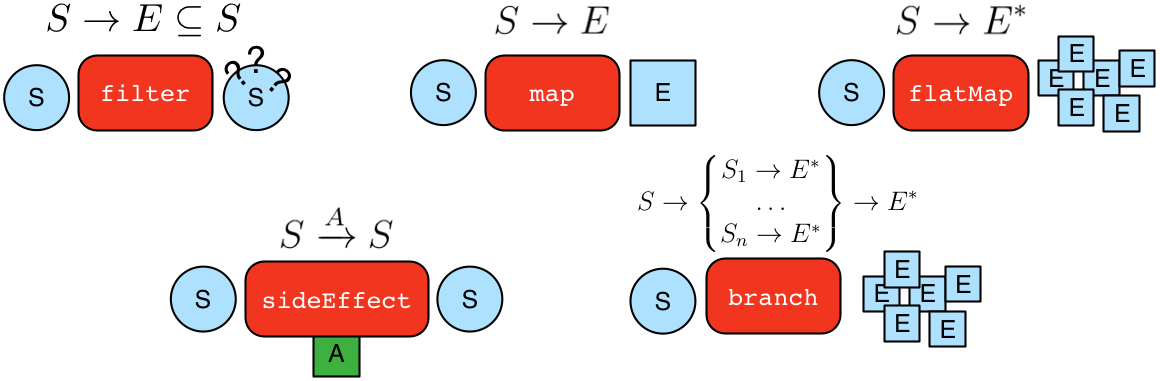A `GraphTraversal<S,E>` is spawned from a `GraphTraversalSource`. It can also be spawned anonymously (i.e. empty) via `__`. A graph traversal is composed of an ordered list of steps. All the steps provided by `GraphTraversal` inherit from the more general forms diagrammed above. A list of all the steps (and their descriptions) are provided in the TinkerPop3 GraphTraversal JavaDoc. The following subsections will demonstrate the GraphTraversal steps using the Gremlin Console.

 Important The basics for starting a traversal are described in The Graph Process section as well as in the Getting Started tutorial.
 Note To reduce the verbosity of the expression, it is good to `import static org.apache.tinkerpop.gremlin.process.traversal.dsl.graph..*`. This way, instead of doing `.inE()` for an anonymous traversal, it is possible to simply write `inE()`. Be aware of language-specific reserved keywords when using anonymous traversals. For example, `in` and `as` are reserved keywords in Groovy, therefore you must use the verbose syntax `.in()` and `.as()` to avoid collisions.

### General Steps

There are five general steps, each having a traversal and a lambda representation, by which all other specific steps described later extend.

Step Description

`map(Traversal<S, E>)` `map(Function<Traverser<S>, E>)`

map the traverser to some object of type `E` for the next step to process.

`flatMap(Traversal<S, E>)` `flatMap(Function<Traverser<S>, Iterator<E>>)`

map the traverser to an iterator of `E` objects that are streamed to the next step.

`filter(Traversal<?, ?>)` `filter(Predicate<Traverser<S>>)`

map the traverser to either true or false, where false will not pass the traverser to the next step.

`sideEffect(Traversal<S, S>)` `sideEffect(Consumer<Traverser<S>>)`

perform some operation on the traverser and pass it to the next step.

`branch(Traversal<S, M>)` `branch(Function<Traverser<S>,M>)`

split the traverser to all the traversals indexed by the `M` token.

 Warning Lambda steps are presented for educational purposes as they represent the foundational constructs of the Gremlin language. In practice, lambda steps should be avoided in favor of their traversals representation and traversal verification strategies exist to disallow their use unless explicitly "turned off." For more information on the problems with lambdas, please read A Note on Lambdas.

The `Traverser<S>` object provides access to:

1. The current traversed `S` object — `Traverser.get()`.

2. The current path traversed by the traverser — `Traverser.path()`.

1. A helper shorthand to get a particular path-history object — `Traverser.path(String) == Traverser.path().get(String)`.

3. The number of times the traverser has gone through the current loop — `Traverser.loops()`.

4. The number of objects represented by this traverser — `Traverser.bulk()`.

5. The local data structure associated with this traverser — `Traverser.sack()`.

6. The side-effects associated with the traversal — `Traverser.sideEffects()`.

1. A helper shorthand to get a particular side-effect — `Traverser.sideEffect(String) == Traverser.sideEffects().get(String)`.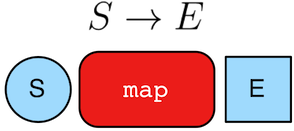``````gremlin> g.V(1).out().values('name') //1\
==>lop
==>josh
gremlin> g.V(1).out().map {it.get().value('name')} //2\
==>lop
==>josh
gremlin> g.V(1).out().map(values('name')) //3\
==>lop
==>josh``````
``````g.V(1).out().values('name') //1\
g.V(1).out().map {it.get().value('name')} //2\
g.V(1).out().map(values('name')) //3``````
1. An outgoing traversal from vertex 1 to the name values of the adjacent vertices.

2. The same operation, but using a lambda to access the name property values.

3. Again the same operation, but using the traversal representation of `map()`.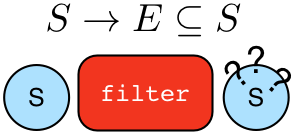``````gremlin> g.V().filter {it.get().label() == 'person'} //1\
==>v
==>v
==>v
==>v
gremlin> g.V().filter(label().is('person')) //2\
==>v
==>v
==>v
==>v
gremlin> g.V().hasLabel('person') //3\
==>v
==>v
==>v
==>v``````
``````g.V().filter {it.get().label() == 'person'} //1\
g.V().filter(label().is('person')) //2\
g.V().hasLabel('person') //3``````
1. A filter that only allows the vertex to pass if it has the "person" label

2. The same operation, but using the traversal representation of `filter()`.

3. The more specific `has()`-step is implemented as a `filter()` with respective predicate.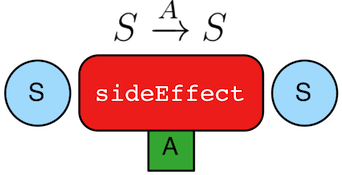``````gremlin> g.V().hasLabel('person').sideEffect(System.out.&println) //1\
v
==>v
v
==>v
v
==>v
v
==>v
gremlin> g.V().sideEffect(outE().count().store("o")).
sideEffect(inE().count().store("i")).cap("o","i") //2\
==>[i:[0,0,1,1,1,3],o:[3,0,0,0,2,1]]``````
``````g.V().hasLabel('person').sideEffect(System.out.&println) //1\
g.V().sideEffect(outE().count().store("o")).
sideEffect(inE().count().store("i")).cap("o","i") //2``````
1. Whatever enters `sideEffect()` is passed to the next step, but some intervening process can occur.

2. Compute the out- and in-degree for each vertex. Both `sideEffect()` are fed with the same vertex.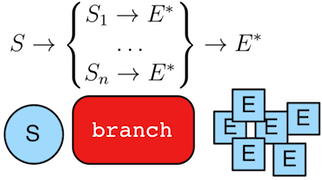``````gremlin> g.V().branch {it.get().value('name')}.
option('marko', values('age')).
option(none, values('name')) //1\
==>29
==>lop
==>josh
==>ripple
==>peter
gremlin> g.V().branch(values('name')).
option('marko', values('age')).
option(none, values('name')) //2\
==>29
==>lop
==>josh
==>ripple
==>peter
gremlin> g.V().choose(has('name','marko'),
values('age'),
values('name')) //3\
==>29
==>lop
==>josh
==>ripple
==>peter``````
``````g.V().branch {it.get().value('name')}.
option('marko', values('age')).
option(none, values('name')) //1\
g.V().branch(values('name')).
option('marko', values('age')).
option(none, values('name')) //2\
g.V().choose(has('name','marko'),
values('age'),
values('name')) //3``````
1. If the vertex is "marko", get his age, else get the name of the vertex.

2. The same operation, but using the traversal representing of `branch()`.

3. The more specific boolean-based `choose()`-step is implemented as a `branch()`.

### Terminal Steps

Typically, when a step is concatenated to a traversal a traversal is returned. In this way, a traversal is built up in a fluent, monadic fashion. However, some steps do not return a traversal, but instead, execute the traversal and return a result. These steps are known as terminal steps (terminal) and they are explained via the examples below.

``````gremlin> g.V().out('created').hasNext() //1\
==>true
gremlin> g.V().out('created').next() //2\
==>v
gremlin> g.V().out('created').next(2) //3\
==>v
==>v
gremlin> g.V().out('nothing').tryNext() //4\
==>Optional.empty
gremlin> g.V().out('created').toList() //5\
==>v
==>v
==>v
==>v
gremlin> g.V().out('created').toSet() //6\
==>v
==>v
gremlin> g.V().out('created').toBulkSet() //7\
==>v
==>v
==>v
==>v
gremlin> results = ['blah',3]
==>blah
==>3
gremlin> g.V().out('created').fill(results) //8\
==>blah
==>3
==>v
==>v
==>v
==>v
``````g.V().out('created').hasNext() //1\
g.V().out('created').next() //2\
g.V().out('created').next(2) //3\
g.V().out('nothing').tryNext() //4\
g.V().out('created').toList() //5\
g.V().out('created').toSet() //6\
g.V().out('created').toBulkSet() //7\
results = ['blah',3]
g.V().out('created').fill(results) //8\
1. `hasNext()` determines whether there are available results.

2. `next()` will return the next result.

3. `next(n)` will return the next `n` results in a list.

4. `tryNext()` will return an `Optional` and thus, is a composite of `hasNext()`/`next()`.

5. `toList()` will return all results in a list.

6. `toSet()` will return all results in a set (thus, duplicates removed).

7. `toBulkSet()` will return all results in a weighted set (thus, duplicates preserved via weighting).

8. `fill(collection)` will put all results in the provided collection and return the collection when complete.

9. `iterate()` does not exactly fit the definition of a terminal step in that it doesn’t return a result, but still returns a traversal - it does however behave as a terminal step in that it iterates the traversal and generates side effects without returning the actual result.

Finally, `explain()`-step is also a terminal step and is described in its own section.

Reasoning is the process of making explicit what is implicit in the data. What is explicit in a graph are the objects of the graph — i.e. vertices and edges. What is implicit in the graph is the traversal. In other words, traversals expose meaning where the meaning is determined by the traversal definition. For example, take the concept of a "co-developer." Two people are co-developers if they have worked on the same project together. This concept can be represented as a traversal and thus, the concept of "co-developers" can be derived. Moreover, what was once implicit can be made explicit via the `addE()`-step (map/sideEffect).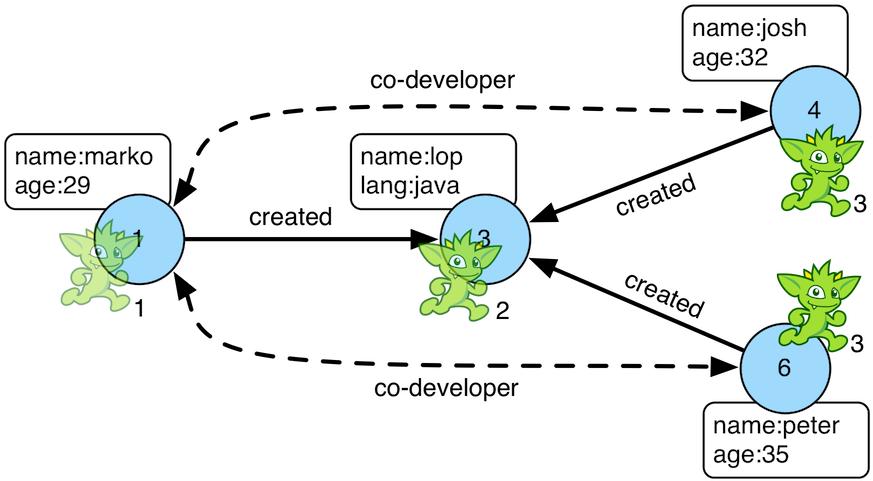``````gremlin> g.V(1).as('a').out('created').in('created').where(neq('a')).
==>e[1-co-developer->4]
==>e[1-co-developer->6]
gremlin> g.V(3,4,5).aggregate('x').has('name','josh').as('a').
==>e[3-createdBy->4]
==>e[5-createdBy->4]
==>e[3-createdBy->1]
==>e[5-createdBy->4]
==>e[3-createdBy->4]
==>e[3-createdBy->6]
gremlin> g.V(1).as('a').out('knows').
inV().inE('livesNear').values('year') //4\
==>2009
==>2009
gremlin> g.V().match(
__.as('a').out('knows').as('b'),
__.as('a').out('created').as('c'),
__.as('b').out('created').as('c')).
property(id,23).property('project',select('c').values('name')) //5\
==>e[1-friendlyCollaborator->4]
gremlin> g.E(23).valueMap()
==>[project:lop]
gremlin> marko = g.V().has('name','marko').next()
==>v
gremlin> peter = g.V().has('name','peter').next()
==>v
==>e[1-knows->6]
==>e[1-knows->6]``````
``````g.V(1).as('a').out('created').in('created').where(neq('a')).
g.V(3,4,5).aggregate('x').has('name','josh').as('a').
g.V(1).as('a').out('knows').
inV().inE('livesNear').values('year') //4\
g.V().match(
__.as('a').out('knows').as('b'),
__.as('a').out('created').as('c'),
__.as('b').out('created').as('c')).
property(id,23).property('project',select('c').values('name')) //5\
g.E(23).valueMap()
marko = g.V().has('name','marko').next()
peter = g.V().has('name','peter').next()
1. Add a co-developer edge with a year-property between marko and his collaborators.

2. Add incoming createdBy edges from the josh-vertex to the lop- and ripple-vertices.

3. Add an inverse createdBy edge for all created edges.

4. The newly created edge is a traversable object.

5. Two arbitrary bindings in a traversal can be joined `from()`→`to()`, where `id` can be provided for graphs that supports user provided ids.

6. Add an edge between marko and peter given the directed (detached) vertex references.

7. Add an edge between marko and peter given the directed (detached) vertex references.

The `addV()`-step is used to add vertices to the graph (map/sideEffect). For every incoming object, a vertex is created. Moreover, `GraphTraversalSource` maintains an `addV()` method.

``````gremlin> g.addV('person').property('name','stephen')
==>v
gremlin> g.V().values('name')
==>marko
==>lop
==>josh
==>ripple
==>peter
==>stephen
==>v
==>v
gremlin> g.V().has('name','nothing')
==>v
==>v
gremlin> g.V().has('name','nothing').bothE()``````
``````g.addV('person').property('name','stephen')
g.V().values('name')
g.V().has('name','nothing')
g.V().has('name','nothing').bothE()``````

The `property()`-step is used to add properties to the elements of the graph (sideEffect). Unlike `addV()` and `addE()`, `property()` is a full sideEffect step in that it does not return the property it created, but the element that streamed into it. Moreover, if `property()` follows an `addV()` or `addE()`, then it is "folded" into the previous step to enable vertex and edge creation with all its properties in one creation operation.

``````gremlin> g.V(1).property('country','usa')
==>v
gremlin> g.V(1).property('city','santa fe').property('state','new mexico').valueMap()
==>[country:[usa],city:[santa fe],name:[marko],state:[new mexico],age:]
gremlin> g.V(1).property(list,'age',35) //1\
==>v
gremlin> g.V(1).valueMap()
==>[country:[usa],city:[santa fe],name:[marko],state:[new mexico],age:[29,35]]
gremlin> g.V(1).property('friendWeight',outE('knows').values('weight').sum(),'acl','private') //2\
==>v
gremlin> g.V(1).properties('friendWeight').valueMap() //3\
==>[acl:private]``````
``````g.V(1).property('country','usa')
g.V(1).property('city','santa fe').property('state','new mexico').valueMap()
g.V(1).property(list,'age',35) //1\
g.V(1).valueMap()
g.V(1).property('friendWeight',outE('knows').values('weight').sum(),'acl','private') //2\
g.V(1).properties('friendWeight').valueMap() //3``````
1. For vertices, a cardinality can be provided for vertex properties.

2. It is possible to select the property value (as well as key) via a traversal.

3. For vertices, the `property()`-step can add meta-properties.

### Aggregate Step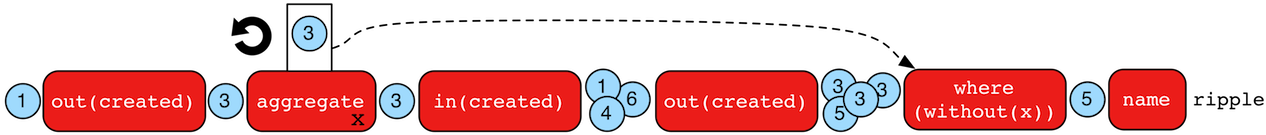The `aggregate()`-step (sideEffect) is used to aggregate all the objects at a particular point of traversal into a `Collection`. The step uses eager evaluation in that no objects continue on until all previous objects have been fully aggregated (as opposed to `store()` which lazily fills a collection). The eager evaluation nature is crucial in situations where everything at a particular point is required for future computation. An example is provided below.

``````gremlin> g.V(1).out('created') //1\
==>v
gremlin> g.V(1).out('created').aggregate('x') //2\
==>v
gremlin> g.V(1).out('created').aggregate('x').in('created') //3\
==>v
==>v
==>v
gremlin> g.V(1).out('created').aggregate('x').in('created').out('created') //4\
==>v
==>v
==>v
==>v
gremlin> g.V(1).out('created').aggregate('x').in('created').out('created').
where(without('x')).values('name') //5\
==>ripple``````
``````g.V(1).out('created') //1\
g.V(1).out('created').aggregate('x') //2\
g.V(1).out('created').aggregate('x').in('created') //3\
g.V(1).out('created').aggregate('x').in('created').out('created') //4\
g.V(1).out('created').aggregate('x').in('created').out('created').
where(without('x')).values('name') //5``````
1. What has marko created?

2. Aggregate all his creations.

3. Who are marko’s collaborators?

4. What have marko’s collaborators created?

5. What have marko’s collaborators created that he hasn’t created?

In recommendation systems, the above pattern is used:

`"What has userA liked? Who else has liked those things? What have they liked that userA hasn't already liked?"`

Finally, `aggregate()`-step can be modulated via `by()`-projection.

``````gremlin> g.V().out('knows').aggregate('x').cap('x')
==>[v,v]
gremlin> g.V().out('knows').aggregate('x').by('name').cap('x')
``````g.V().out('knows').aggregate('x').cap('x')
g.V().out('knows').aggregate('x').by('name').cap('x')``````

### And Step

The `and()`-step ensures that all provided traversals yield a result (filter). Please see `or()` for or-semantics.

 Python The term `and` is a reserved word in Python, and therefore must be referred to in Gremlin with `and_()`.
``````gremlin> g.V().and(
outE('knows'),
values('age').is(lt(30))).
values('name')
==>marko``````
``````g.V().and(
outE('knows'),
values('age').is(lt(30))).
values('name')``````

The `and()`-step can take an arbitrary number of traversals. All traversals must produce at least one output for the original traverser to pass to the next step.

An infix notation can be used as well. Though, with infix notation, only two traversals can be and’d together.

``````gremlin> g.V().where(outE('created').and().outE('knows')).values('name')
==>marko``````
``g.V().where(outE('created').and().outE('knows')).values('name')``

### As Step

The `as()`-step is not a real step, but a "step modulator" similar to `by()` and `option()`. With `as()`, it is possible to provide a label to the step that can later be accessed by steps and data structures that make use of such labels — e.g., `select()`, `match()`, and path.

 Groovy The term `as` is a reserved word in Groovy, and when therefore used as part of an anonymous traversal must be referred to in Gremlin with the double underscore `__.as()`.
 Python The term `as` is a reserved word in Python, and therefore must be referred to in Gremlin with `as_()`.
``````gremlin> g.V().as('a').out('created').as('b').select('a','b') //1\
==>[a:v,b:v]
==>[a:v,b:v]
==>[a:v,b:v]
==>[a:v,b:v]
gremlin> g.V().as('a').out('created').as('b').select('a','b').by('name') //2\
==>[a:marko,b:lop]
==>[a:josh,b:ripple]
==>[a:josh,b:lop]
==>[a:peter,b:lop]``````
``````g.V().as('a').out('created').as('b').select('a','b') //1\
g.V().as('a').out('created').as('b').select('a','b').by('name') //2``````
1. Select the objects labeled "a" and "b" from the path.

2. Select the objects labeled "a" and "b" from the path and, for each object, project its name value.

A step can have any number of labels associated with it. This is useful for referencing the same step multiple times in a future step.

``````gremlin> g.V().hasLabel('software').as('a','b','c').
select('a','b','c').
by('name').
by('lang').
by(__.in('created').values('name').fold())
==>[a:lop,b:java,c:[marko,josh,peter]]
==>[a:ripple,b:java,c:[josh]]``````
``````g.V().hasLabel('software').as('a','b','c').
select('a','b','c').
by('name').
by('lang').
by(__.in('created').values('name').fold())``````

### Barrier Step

The `barrier()`-step (barrier) turns the lazy traversal pipeline into a bulk-synchronous pipeline. This step is useful in the following situations:

• When everything prior to `barrier()` needs to be executed before moving onto the steps after the `barrier()` (i.e. ordering).

• When "stalling" the traversal may lead to a "bulking optimization" in traversals that repeatedly touch many of the same elements (i.e. optimizing).

``````gremlin> g.V().sideEffect{println "first: \${it}"}.sideEffect{println "second: \${it}"}.iterate()
first: v
second: v
first: v
second: v
first: v
second: v
first: v
second: v
first: v
second: v
first: v
second: v
gremlin> g.V().sideEffect{println "first: \${it}"}.barrier().sideEffect{println "second: \${it}"}.iterate()
first: v
first: v
first: v
first: v
first: v
first: v
second: v
second: v
second: v
second: v
second: v
second: v``````
``````g.V().sideEffect{println "first: \${it}"}.sideEffect{println "second: \${it}"}.iterate()
g.V().sideEffect{println "first: \${it}"}.barrier().sideEffect{println "second: \${it}"}.iterate()``````

The theory behind a "bulking optimization" is simple. If there are one million traversers at vertex 1, then there is no need to calculate one million `both()`-computations. Instead, represent those one million traversers as a single traverser with a `Traverser.bulk()` equal to one million and execute `both()` once. A bulking optimization example is made more salient on a larger graph. Therefore, the example below leverages the Grateful Dead graph.

``````gremlin> graph = TinkerGraph.open()
==>tinkergraph[vertices:0 edges:0]
gremlin> g = graph.traversal().withoutStrategies(LazyBarrierStrategy) //1\
==>graphtraversalsource[tinkergraph[vertices:808 edges:8049], standard]
gremlin> clockWithResult(1){g.V().both().both().both().count().next()} //2\
==>11199.284839
==>126653966
gremlin> clockWithResult(1){g.V().repeat(both()).times(3).count().next()} //3\
==>15.719280999999999
==>126653966
gremlin> clockWithResult(1){g.V().both().barrier().both().barrier().both().barrier().count().next()} //4\
==>16.148671
==>126653966``````
``````graph = TinkerGraph.open()
g = graph.traversal().withoutStrategies(LazyBarrierStrategy) //1\
clockWithResult(1){g.V().both().both().both().count().next()} //2\
clockWithResult(1){g.V().repeat(both()).times(3).count().next()} //3\
clockWithResult(1){g.V().both().barrier().both().barrier().both().barrier().count().next()} //4``````
1. Explicitly remove `LazyBarrierStrategy` which yields a bulking optimization.

2. A non-bulking traversal where each traverser is processed.

3. Each traverser entering `repeat()` has its recursion bulked.

4. A bulking traversal where implicit traversers are not processed.

If `barrier()` is provided an integer argument, then the barrier will only hold `n`-number of unique traversers in its barrier before draining the aggregated traversers to the next step. This is useful in the aforementioned bulking optimization scenario with the added benefit of reducing the risk of an out-of-memory exception.

`LazyBarrierStrategy` inserts `barrier()`-steps into a traversal where appropriate in order to gain the "bulking optimization."

``````gremlin> graph = TinkerGraph.open()
==>tinkergraph[vertices:0 edges:0]
gremlin> g = graph.traversal() //1\
==>graphtraversalsource[tinkergraph[vertices:808 edges:8049], standard]
gremlin> clockWithResult(1){g.V().both().both().both().count().next()}
==>18.039438999999998
==>126653966
gremlin> g.V().both().both().both().count().iterate().toString() //2\
==>[TinkerGraphStep(vertex,[]), VertexStep(BOTH,vertex), NoOpBarrierStep(2500), VertexStep(BOTH,vertex), NoOpBarrierStep(2500), VertexStep(BOTH,edge), CountGlobalStep, NoneStep]``````
``````graph = TinkerGraph.open()
g = graph.traversal() //1\
clockWithResult(1){g.V().both().both().both().count().next()}
g.V().both().both().both().count().iterate().toString()  //2``````
1. `LazyBarrierStrategy` is a default strategy and thus, does not need to be explicitly activated.

2. With `LazyBarrierStrategy` activated, `barrier()`-steps are automatically inserted where appropriate.

### By Step

The `by()`-step is not an actual step, but instead is a "step-modulator" similar to `as()` and `option()`. If a step is able to accept traversals, functions, comparators, etc. then `by()` is the means by which they are added. The general pattern is `step().by()…​by()`. Some steps can only accept one `by()` while others can take an arbitrary amount.

``````gremlin> g.V().group().by(bothE().count()) //1\
==>[1:[v,v,v],3:[v,v,v]]
gremlin> g.V().group().by(bothE().count()).by('name') //2\
gremlin> g.V().group().by(bothE().count()).by(count()) //3\
==>[1:3,3:3]``````
``````g.V().group().by(bothE().count()) //1\
g.V().group().by(bothE().count()).by('name') //2\
g.V().group().by(bothE().count()).by(count())  //3``````
1. `by(outE().count())` will group the elements by their edge count (traversal).

2. `by('name')` will process the grouped elements by their name (element property projection).

3. `by(count())` will count the number of elements in each group (traversal).

The following steps all support `by()`-modulation. Note that the semantics of such modulation should be understood on a step-by-step level and thus, as discussed in their respective section of the documentation.

### Cap Step

The `cap()`-step (barrier) iterates the traversal up to itself and emits the sideEffect referenced by the provided key. If multiple keys are provided, then a `Map<String,Object>` of sideEffects is emitted.

``````gremlin> g.V().groupCount('a').by(label).cap('a') //1\
==>[software:2,person:4]
gremlin> g.V().groupCount('a').by(label).groupCount('b').by(outE().count()).cap('a','b') //2\
==>[a:[software:2,person:4],b:[0:3,1:1,2:1,3:1]]``````
``````g.V().groupCount('a').by(label).cap('a') //1\
g.V().groupCount('a').by(label).groupCount('b').by(outE().count()).cap('a','b')   //2``````
1. Group and count vertices by their label. Emit the side effect labeled 'a', which is the group count by label.

2. Same as statement 1, but also emit the side effect labeled 'b' which groups vertices by the number of out edges.

### Choose Step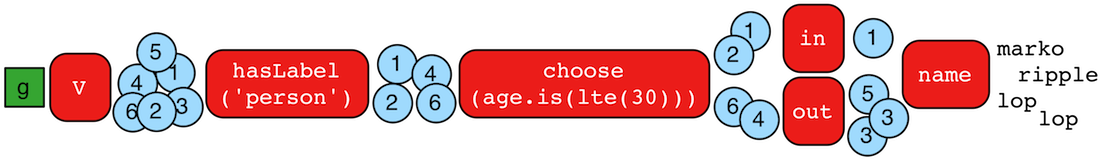The `choose()`-step (branch) routes the current traverser to a particular traversal branch option. With `choose()`, it is possible to implement if/then/else-semantics as well as more complicated selections.

``````gremlin> g.V().hasLabel('person').
choose(values('age').is(lte(30)),
__.in(),
__.out()).values('name') //1\
==>marko
==>ripple
==>lop
==>lop
gremlin> g.V().hasLabel('person').
choose(values('age')).
option(27, __.in()).
option(32, __.out()).values('name') //2\
==>marko
==>ripple
==>lop``````
``````g.V().hasLabel('person').
choose(values('age').is(lte(30)),
__.in(),
__.out()).values('name') //1\
g.V().hasLabel('person').
choose(values('age')).
option(27, __.in()).
option(32, __.out()).values('name') //2``````
1. If the traversal yields an element, then do `in`, else do `out` (i.e. true/false-based option selection).

2. Use the result of the traversal as a key to the map of traversal options (i.e. value-based option selection).

If the "false"-branch is not provided, then if/then-semantics are implemented.

``````gremlin> g.V().choose(hasLabel('person'), out('created')).values('name') //1\
==>lop
==>lop
==>ripple
==>lop
==>ripple
==>lop
gremlin> g.V().choose(hasLabel('person'), out('created'), identity()).values('name') //2\
==>lop
==>lop
==>ripple
==>lop
==>ripple
==>lop``````
``````g.V().choose(hasLabel('person'), out('created')).values('name') //1\
g.V().choose(hasLabel('person'), out('created'), identity()).values('name') //2``````
1. If the vertex is a person, emit the vertices they created, else emit the vertex.

2. If/then/else with an `identity()` on the false-branch is equivalent to if/then with no false-branch.

Note that `choose()` can have an arbitrary number of options and moreover, can take an anonymous traversal as its choice function.

``````gremlin> g.V().hasLabel('person').
choose(values('name')).
option('marko', values('age')).
option('josh', values('name')).
option('peter', label())
==>29
==>josh
==>person``````
``````g.V().hasLabel('person').
choose(values('name')).
option('marko', values('age')).
option('josh', values('name')).
option('peter', label())``````

The `choose()`-step can leverage the `Pick.none` option match. For anything that does not match a specified option, the `none`-option is taken.

``````gremlin> g.V().hasLabel('person').
choose(values('name')).
option('marko', values('age')).
option(none, values('name'))
==>29
==>josh
==>peter``````
``````g.V().hasLabel('person').
choose(values('name')).
option('marko', values('age')).
option(none, values('name'))``````

### Coalesce Step

The `coalesce()`-step evaluates the provided traversals in order and returns the first traversal that emits at least one element.

``````gremlin> g.V(1).coalesce(outE('knows'), outE('created')).inV().path().by('name').by(label)
==>[marko,knows,josh]
gremlin> g.V(1).coalesce(outE('created'), outE('knows')).inV().path().by('name').by(label)
==>[marko,created,lop]
gremlin> g.V(1).property('nickname', 'okram')
==>v
gremlin> g.V().hasLabel('person').coalesce(values('nickname'), values('name'))
==>okram
==>josh
==>peter``````
``````g.V(1).coalesce(outE('knows'), outE('created')).inV().path().by('name').by(label)
g.V(1).coalesce(outE('created'), outE('knows')).inV().path().by('name').by(label)
g.V(1).property('nickname', 'okram')
g.V().hasLabel('person').coalesce(values('nickname'), values('name'))``````

### Coin Step

To randomly filter out a traverser, use the `coin()`-step (filter). The provided double argument biases the "coin toss."

``````gremlin> g.V().coin(0.5)
==>v
==>v
==>v
==>v
gremlin> g.V().coin(0.0)
gremlin> g.V().coin(1.0)
==>v
==>v
==>v
==>v
==>v
==>v``````
``````g.V().coin(0.5)
g.V().coin(0.0)
g.V().coin(1.0)``````

### Constant Step

To specify a constant value for a traverser, use the `constant()`-step (map). This is often useful with conditional steps like `choose()`-step or `coalesce()`-step.

``````gremlin> g.V().choose(hasLabel('person'),
values('name'),
constant('inhuman')) //1\
==>marko
==>inhuman
==>josh
==>inhuman
==>peter
gremlin> g.V().coalesce(
hasLabel('person').values('name'),
constant('inhuman')) //2\
==>marko
==>inhuman
==>josh
==>inhuman
==>peter``````
``````g.V().choose(hasLabel('person'),
values('name'),
constant('inhuman')) //1\
g.V().coalesce(
hasLabel('person').values('name'),
constant('inhuman')) //2``````
1. Show the names of people, but show "inhuman" for other vertices.

2. Same as statement 1 (unless there is a person vertex with no name).

### Count Step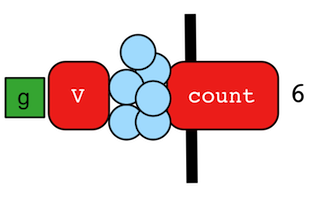The `count()`-step (map) counts the total number of represented traversers in the streams (i.e. the bulk count).

``````gremlin> g.V().count()
==>6
gremlin> g.V().hasLabel('person').count()
==>4
gremlin> g.V().hasLabel('person').outE('created').count().path() //1\
==>
gremlin> g.V().hasLabel('person').outE('created').count().map {it.get() * 10}.path() //2\
==>[4,40]``````
``````g.V().count()
g.V().hasLabel('person').count()
g.V().hasLabel('person').outE('created').count().path() //1\
g.V().hasLabel('person').outE('created').count().map {it.get() * 10}.path() //2``````
1. `count()`-step is a reducing barrier step meaning that all of the previous traversers are folded into a new traverser.

2. The path of the traverser emanating from `count()` starts at `count()`.

 Important `count(local)` counts the current, local object (not the objects in the traversal stream). This works for `Collection`- and `Map`-type objects. For any other object, a count of 1 is returned.

### CyclicPath Step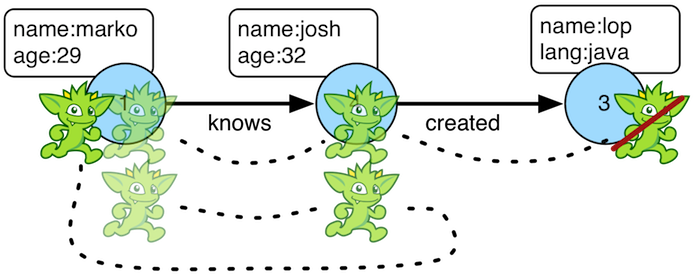Each traverser maintains its history through the traversal over the graph — i.e. its path. If it is important that the traverser repeat its course, then `cyclic()`-path should be used (filter). The step analyzes the path of the traverser thus far and if there are any repeats, the traverser is filtered out over the traversal computation. If non-cyclic behavior is desired, see `simplePath()`.

``````gremlin> g.V(1).both().both()
==>v
==>v
==>v
==>v
==>v
==>v
==>v
gremlin> g.V(1).both().both().cyclicPath()
==>v
==>v
==>v
gremlin> g.V(1).both().both().cyclicPath().path()
==>[v,v,v]
==>[v,v,v]
==>[v,v,v]
gremlin> g.V(1).as('a').out('created').as('b').
in('created').as('c').
cyclicPath().
path()
==>[v,v,v]
gremlin> g.V(1).as('a').out('created').as('b').
in('created').as('c').
cyclicPath().from('a').to('b').
path()``````
``````g.V(1).both().both()
g.V(1).both().both().cyclicPath()
g.V(1).both().both().cyclicPath().path()
g.V(1).as('a').out('created').as('b').
in('created').as('c').
cyclicPath().
path()
g.V(1).as('a').out('created').as('b').
in('created').as('c').
cyclicPath().from('a').to('b').
path()``````

### Dedup Step

With `dedup()`-step (filter), repeatedly seen objects are removed from the traversal stream. Note that if a traverser’s bulk is greater than 1, then it is set to 1 before being emitted.

``````gremlin> g.V().values('lang')
==>java
==>java
gremlin> g.V().values('lang').dedup()
==>java
gremlin> g.V(1).repeat(bothE('created').dedup().otherV()).emit().path() //1\
==>[v,e[1-created->3],v]
==>[v,e[1-created->3],v,e[4-created->3],v]
==>[v,e[1-created->3],v,e[6-created->3],v]
==>[v,e[1-created->3],v,e[4-created->3],v,e[4-created->5],v]``````
``````g.V().values('lang')
g.V().values('lang').dedup()
g.V(1).repeat(bothE('created').dedup().otherV()).emit().path() //1``````
1. Traverse all `created` edges, but don’t touch any edge twice.

If a by-step modulation is provided to `dedup()`, then the object is processed accordingly prior to determining if it has been seen or not.

``````gremlin> g.V().valueMap(true, 'name')
==>[id:1,label:person,name:[marko]]
==>[id:3,label:software,name:[lop]]
==>[id:4,label:person,name:[josh]]
==>[id:5,label:software,name:[ripple]]
==>[id:6,label:person,name:[peter]]
gremlin> g.V().dedup().by(label).values('name')
==>marko
==>lop``````
``````g.V().valueMap(true, 'name')
g.V().dedup().by(label).values('name')``````

Finally, if `dedup()` is provided an array of strings, then it will ensure that the de-duplication is not with respect to the current traverser object, but to the path history of the traverser.

``````gremlin> g.V().as('a').out('created').as('b').in('created').as('c').select('a','b','c')
==>[a:v,b:v,c:v]
==>[a:v,b:v,c:v]
==>[a:v,b:v,c:v]
==>[a:v,b:v,c:v]
==>[a:v,b:v,c:v]
==>[a:v,b:v,c:v]
==>[a:v,b:v,c:v]
==>[a:v,b:v,c:v]
==>[a:v,b:v,c:v]
==>[a:v,b:v,c:v]
gremlin> g.V().as('a').out('created').as('b').in('created').as('c').dedup('a','b').select('a','b','c') //1\
==>[a:v,b:v,c:v]
==>[a:v,b:v,c:v]
==>[a:v,b:v,c:v]
==>[a:v,b:v,c:v]``````
``````g.V().as('a').out('created').as('b').in('created').as('c').select('a','b','c')
g.V().as('a').out('created').as('b').in('created').as('c').dedup('a','b').select('a','b','c') //1``````
1. If the current `a` and `b` combination has been seen previously, then filter the traverser.

### Drop Step

The `drop()`-step (filter/sideEffect) is used to remove element and properties from the graph (i.e. remove). It is a filter step because the traversal yields no outgoing objects.

``````gremlin> g.V().outE().drop()
gremlin> g.E()
gremlin> g.V().properties('name').drop()
gremlin> g.V().valueMap()
==>[age:]
==>[age:]
==>[lang:[java]]
==>[age:]
==>[lang:[java]]
==>[age:]
gremlin> g.V().drop()
gremlin> g.V()``````
``````g.V().outE().drop()
g.E()
g.V().properties('name').drop()
g.V().valueMap()
g.V().drop()
g.V()``````

### Emit Step

The `emit`-step is not an actual step, but is instead a step modulator for `repeat()` (find more documentation on the `emit()` there).

### Explain Step

The `explain()`-step (terminal) will return a `TraversalExplanation`. A traversal explanation details how the traversal (prior to `explain()`) will be compiled given the registered traversal strategies. A `TraversalExplanation` has a `toString()` representation with 3-columns. The first column is the traversal strategy being applied. The second column is the traversal strategy category: [D]ecoration, [O]ptimization, [P]rovider optimization, [F]inalization, and [V]erification. Finally, the third column is the state of the traversal post strategy application. The final traversal is the resultant execution plan.

``````gremlin> g.V().hasLabel('person').outE().identity().inV().count().is(gt(5)).explain()
==>Traversal Explanation
=====================================================================================================================================================================================================
Original Traversal                 [GraphStep(vertex,[]), HasStep([~label.eq(person)]), VertexStep(OUT,edge), IdentityStep, EdgeVertexStep(IN), CountGlobalStep, IsStep(gt(5))]

ConnectiveStrategy           [D]   [GraphStep(vertex,[]), HasStep([~label.eq(person)]), VertexStep(OUT,edge), IdentityStep, EdgeVertexStep(IN), CountGlobalStep, IsStep(gt(5))]
EarlyLimitStrategy           [O]   [GraphStep(vertex,[]), HasStep([~label.eq(person)]), VertexStep(OUT,edge), IdentityStep, EdgeVertexStep(IN), CountGlobalStep, IsStep(gt(5))]
MatchPredicateStrategy       [O]   [GraphStep(vertex,[]), HasStep([~label.eq(person)]), VertexStep(OUT,edge), IdentityStep, EdgeVertexStep(IN), CountGlobalStep, IsStep(gt(5))]
FilterRankingStrategy        [O]   [GraphStep(vertex,[]), HasStep([~label.eq(person)]), VertexStep(OUT,edge), IdentityStep, EdgeVertexStep(IN), CountGlobalStep, IsStep(gt(5))]
InlineFilterStrategy         [O]   [GraphStep(vertex,[]), HasStep([~label.eq(person)]), VertexStep(OUT,edge), IdentityStep, EdgeVertexStep(IN), CountGlobalStep, IsStep(gt(5))]
IncidentToAdjacentStrategy   [O]   [GraphStep(vertex,[]), HasStep([~label.eq(person)]), VertexStep(OUT,edge), IdentityStep, EdgeVertexStep(IN), CountGlobalStep, IsStep(gt(5))]
AdjacentToIncidentStrategy   [O]   [GraphStep(vertex,[]), HasStep([~label.eq(person)]), VertexStep(OUT,edge), IdentityStep, EdgeVertexStep(IN), CountGlobalStep, IsStep(gt(5))]
RepeatUnrollStrategy         [O]   [GraphStep(vertex,[]), HasStep([~label.eq(person)]), VertexStep(OUT,edge), IdentityStep, EdgeVertexStep(IN), CountGlobalStep, IsStep(gt(5))]
CountStrategy                [O]   [GraphStep(vertex,[]), HasStep([~label.eq(person)]), VertexStep(OUT,edge), IdentityStep, EdgeVertexStep(IN), RangeGlobalStep(0,6), CountGlobalStep, IsStep(gt(5))]
PathRetractionStrategy       [O]   [GraphStep(vertex,[]), HasStep([~label.eq(person)]), VertexStep(OUT,edge), IdentityStep, EdgeVertexStep(IN), RangeGlobalStep(0,6), CountGlobalStep, IsStep(gt(5))]
LazyBarrierStrategy          [O]   [GraphStep(vertex,[]), HasStep([~label.eq(person)]), VertexStep(OUT,edge), IdentityStep, EdgeVertexStep(IN), RangeGlobalStep(0,6), CountGlobalStep, IsStep(gt(5))]
TinkerGraphCountStrategy     [P]   [GraphStep(vertex,[]), HasStep([~label.eq(person)]), VertexStep(OUT,edge), IdentityStep, EdgeVertexStep(IN), RangeGlobalStep(0,6), CountGlobalStep, IsStep(gt(5))]
TinkerGraphStepStrategy      [P]   [TinkerGraphStep(vertex,[~label.eq(person)]), VertexStep(OUT,edge), IdentityStep, EdgeVertexStep(IN), RangeGlobalStep(0,6), CountGlobalStep, IsStep(gt(5))]
ProfileStrategy              [F]   [TinkerGraphStep(vertex,[~label.eq(person)]), VertexStep(OUT,edge), IdentityStep, EdgeVertexStep(IN), RangeGlobalStep(0,6), CountGlobalStep, IsStep(gt(5))]
StandardVerificationStrategy [V]   [TinkerGraphStep(vertex,[~label.eq(person)]), VertexStep(OUT,edge), IdentityStep, EdgeVertexStep(IN), RangeGlobalStep(0,6), CountGlobalStep, IsStep(gt(5))]

Final Traversal                    [TinkerGraphStep(vertex,[~label.eq(person)]), VertexStep(OUT,edge), IdentityStep, EdgeVertexStep(IN), RangeGlobalStep(0,6), CountGlobalStep, IsStep(gt(5))]``````
``g.V().hasLabel('person').outE().identity().inV().count().is(gt(5)).explain()``

For traversal profiling information, please see `profile()`-step.

### Fold Step

There are situations when the traversal stream needs a "barrier" to aggregate all the objects and emit a computation that is a function of the aggregate. The `fold()`-step (map) is one particular instance of this. Please see `unfold()`-step for the inverse functionality.

``````gremlin> g.V(1).out('knows').values('name')
==>josh
gremlin> g.V(1).out('knows').values('name').fold() //1\
gremlin> g.V(1).out('knows').values('name').fold().next().getClass() //2\
==>class java.util.ArrayList
gremlin> g.V(1).out('knows').values('name').fold(0) {a,b -> a + b.length()} //3\
==>9
gremlin> g.V().values('age').fold(0) {a,b -> a + b} //4\
==>123
gremlin> g.V().values('age').fold(0, sum) //5\
==>123
gremlin> g.V().values('age').sum() //6\
==>123``````
``````g.V(1).out('knows').values('name')
g.V(1).out('knows').values('name').fold() //1\
g.V(1).out('knows').values('name').fold().next().getClass() //2\
g.V(1).out('knows').values('name').fold(0) {a,b -> a + b.length()} //3\
g.V().values('age').fold(0) {a,b -> a + b} //4\
g.V().values('age').fold(0, sum) //5\
g.V().values('age').sum() //6``````
1. A parameterless `fold()` will aggregate all the objects into a list and then emit the list.

2. A verification of the type of list returned.

3. `fold()` can be provided two arguments —  a seed value and a reduce bi-function ("vadas" is 5 characters + "josh" with 4 characters).

4. What is the total age of the people in the graph?

5. The same as before, but using a built-in bi-function.

6. The same as before, but using the `sum()`-step.

### From Step

The `from()`-step is not an actual step, but instead is a "step-modulator" similar to `as()` and `by()`. If a step is able to accept traversals or strings then `from()` is the means by which they are added. The general pattern is `step().from()`. See `to()`-step.

The list of steps that support `from()`-modulation are: `simplePath()`, `cyclicPath()`, `path()`, and `addE()`.

 Javascript The term `from` is a reserved word in Javascript, and therefore must be referred to in Gremlin with `from_()`.
 Python The term `from` is a reserved word in Python, and therefore must be referred to in Gremlin with `from_()`.

### Graph Step

The `V()`-step is usually used to start a `GraphTraversal`, but can also be used mid-traversal.

``````gremlin> g.V().has('name', within('marko', 'vadas', 'josh')).as('person').
==>e[1-uses->3]
==>e[1-uses->5]
==>e[2-uses->3]
==>e[2-uses->5]
==>e[4-uses->3]
==>e[4-uses->5]``````
``````g.V().has('name', within('marko', 'vadas', 'josh')).as('person').
 Note Whether a mid-traversal `V()` uses an index or not, depends on a) whether suitable index exists and b) if the particular graph system provider implemented this functionality.
``````gremlin> g.V().has('name', within('marko', 'vadas', 'josh')).as('person').
``````g.V().has('name', within('marko', 'vadas', 'josh')).as('person').
1. Normally the `V()`-step will iterate over all vertices. However, graph strategies can fold `HasContainer’s into a `GraphStep` to allow index lookups.

2. Whether the graph system provider supports mid-traversal `V()` index lookups or not can easily be determined by inspecting the `toString()` output of the iterated traversal. If `has` conditions were folded into the `V()`-step, an index - if one exists - will be used.

### Group Step

As traversers propagate across a graph as defined by a traversal, sideEffect computations are sometimes required. That is, the actual path taken or the current location of a traverser is not the ultimate output of the computation, but some other representation of the traversal. The `group()`-step (map/sideEffect) is one such sideEffect that organizes the objects according to some function of the object. Then, if required, that organization (a list) is reduced. An example is provided below.

``````gremlin> g.V().group().by(label) //1\
==>[software:[v,v],person:[v,v,v,v]]
gremlin> g.V().group().by(label).by('name') //2\
gremlin> g.V().group().by(label).by(count()) //3\
==>[software:2,person:4]``````
``````g.V().group().by(label) //1\
g.V().group().by(label).by('name') //2\
g.V().group().by(label).by(count()) //3``````
1. Group the vertices by their label.

2. For each vertex in the group, get their name.

3. For each grouping, what is its size?

The two projection parameters available to `group()` via `by()` are:

1. Key-projection: What feature of the object to group on (a function that yields the map key)?

2. Value-projection: What feature of the group to store in the key-list?

### GroupCount Step

When it is important to know how many times a particular object has been at a particular part of a traversal, `groupCount()`-step (map/sideEffect) is used.

`"What is the distribution of ages in the graph?"`
``````gremlin> g.V().hasLabel('person').values('age').groupCount()
==>[32:1,35:1,27:1,29:1]
gremlin> g.V().hasLabel('person').groupCount().by('age') //1\
==>[32:1,35:1,27:1,29:1]``````
``````g.V().hasLabel('person').values('age').groupCount()
g.V().hasLabel('person').groupCount().by('age') //1``````
1. You can also supply a pre-group projection, where the provided `by()`-modulation determines what to group the incoming object by.

There is one person that is 32, one person that is 35, one person that is 27, and one person that is 29.

`"Iteratively walk the graph and count the number of times you see the second letter of each name."`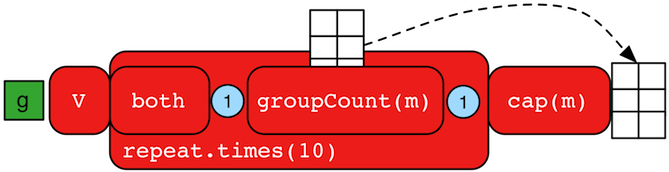``````gremlin> g.V().repeat(both().groupCount('m').by(label)).times(10).cap('m')
==>[software:19598,person:39196]``````
``g.V().repeat(both().groupCount('m').by(label)).times(10).cap('m')``

The above is interesting in that it demonstrates the use of referencing the internal `Map<Object,Long>` of `groupCount()` with a string variable. Given that `groupCount()` is a sideEffect-step, it simply passes the object it received to its output. Internal to `groupCount()`, the object’s count is incremented.

### Has Step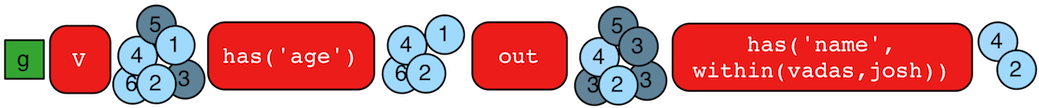It is possible to filter vertices, edges, and vertex properties based on their properties using `has()`-step (filter). There are numerous variations on `has()` including:

• `has(key,value)`: Remove the traverser if its element does not have the provided key/value property.

• `has(label, key, value)`: Remove the traverser if its element does not have the specified label and provided key/value property.

• `has(key,predicate)`: Remove the traverser if its element does not have a key value that satisfies the bi-predicate. For more information on predicates, please read A Note on Predicates.

• `hasLabel(labels…​)`: Remove the traverser if its element does not have any of the labels.

• `hasId(ids…​)`: Remove the traverser if its element does not have any of the ids.

• `hasKey(keys…​)`: Remove the traverser if the property does not have all of the provided keys.

• `hasValue(values…​)`: Remove the traverser if its property does not have all of the provided values.

• `has(key)`: Remove the traverser if its element does not have a value for the key.

• `hasNot(key)`: Remove the traverser if its element has a value for the key.

• `has(key, traversal)`: Remove the traverser if its object does not yield a result through the traversal off the property value.

``````gremlin> g.V().hasLabel('person')
==>v
==>v
==>v
==>v
==>v
==>v
outE().hasLabel('created')
==>e[4-created->5]
==>e[4-created->3]
gremlin> g.V().has('age',inside(20,30)).values('age') //1\
==>29
==>27
gremlin> g.V().has('age',outside(20,30)).values('age') //2\
==>32
==>35
gremlin> g.V().has('name',within('josh','marko')).valueMap() //3\
==>[name:[marko],age:]
==>[name:[josh],age:]
gremlin> g.V().has('name',without('josh','marko')).valueMap() //4\
==>[name:[lop],lang:[java]]
==>[name:[ripple],lang:[java]]
==>[name:[peter],age:]
gremlin> g.V().has('name',not(within('josh','marko'))).valueMap() //5\
==>[name:[lop],lang:[java]]
==>[name:[ripple],lang:[java]]
==>[name:[peter],age:]
==>29
==>27
==>32
==>35
gremlin> g.V().hasNot('age').values('name') //7\
==>lop
==>ripple``````
``````g.V().hasLabel('person')
outE().hasLabel('created')
g.V().has('age',inside(20,30)).values('age') //1\
g.V().has('age',outside(20,30)).values('age') //2\
g.V().has('name',within('josh','marko')).valueMap() //3\
g.V().has('name',without('josh','marko')).valueMap() //4\
g.V().has('name',not(within('josh','marko'))).valueMap() //5\
g.V().hasNot('age').values('name') //7``````
1. Find all vertices whose ages are between 20 (exclusive) and 30 (exclusive). In other words, the age must be greater than 20 and less than 30.

2. Find all vertices whose ages are not between 20 (inclusive) and 30 (inclusive). In other words, the age must be less than 20 or greater than 30.

3. Find all vertices whose names are exact matches to any names in the collection `[josh,marko]`, display all the key,value pairs for those vertices.

4. Find all vertices whose names are not in the collection `[josh,marko]`, display all the key,value pairs for those vertices.

5. Same as the prior example save using `not` on `within` to yield `without`.

6. Find all age-properties and emit their value.

7. Find all vertices that do not have an age-property and emit their name.

TinkerPop does not support a regular expression predicate, although specific graph databases that leverage TinkerPop may provide a partial match extension.

### Id Step

The `id()`-step (map) takes an `Element` and extracts its identifier from it.

``````gremlin> g.V().id()
==>1
==>2
==>3
==>4
==>5
==>6
gremlin> g.V(1).out().id().is(2)
==>2
gremlin> g.V(1).outE().id()
==>9
==>7
==>8
gremlin> g.V(1).properties().id()
==>0
==>1``````
``````g.V().id()
g.V(1).out().id().is(2)
g.V(1).outE().id()
g.V(1).properties().id()``````

### Identity Step

The `identity()`-step (map) is an identity function which maps the current object to itself.

``````gremlin> g.V().identity()
==>v
==>v
==>v
==>v
==>v
==>v``````
``g.V().identity()``

### Inject Step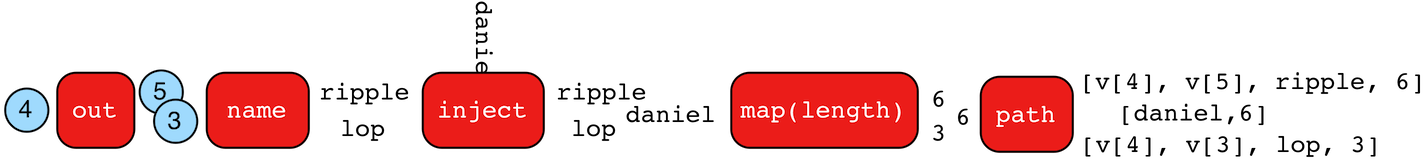One of the major features of TinkerPop3 is "injectable steps." This makes it possible to insert objects arbitrarily into a traversal stream. In general, `inject()`-step (sideEffect) exists and a few examples are provided below.

``````gremlin> g.V(4).out().values('name').inject('daniel')
==>daniel
==>ripple
==>lop
gremlin> g.V(4).out().values('name').inject('daniel').map {it.get().length()}
==>6
==>6
==>3
gremlin> g.V(4).out().values('name').inject('daniel').map {it.get().length()}.path()
==>[daniel,6]
==>[v,v,ripple,6]
==>[v,v,lop,3]``````
``````g.V(4).out().values('name').inject('daniel')
g.V(4).out().values('name').inject('daniel').map {it.get().length()}
g.V(4).out().values('name').inject('daniel').map {it.get().length()}.path()``````

In the last example above, note that the path starting with `daniel` is only of length 2. This is because the `daniel` string was inserted half-way in the traversal. Finally, a typical use case is provided below — when the start of the traversal is not a graph object.

``````gremlin> inject(1,2)
==>1
==>2
gremlin> inject(1,2).map {it.get() + 1}
==>2
==>3
gremlin> inject(1,2).map {it.get() + 1}.map {g.V(it.get()).next()}.values('name')
==>lop``````
``````inject(1,2)
inject(1,2).map {it.get() + 1}
inject(1,2).map {it.get() + 1}.map {g.V(it.get()).next()}.values('name')``````

### Is Step

It is possible to filter scalar values using `is()`-step (filter).

 Python The term `is` is a reserved word in Python, and therefore must be referred to in Gremlin with `is_()`.
``````gremlin> g.V().values('age').is(32)
==>32
gremlin> g.V().values('age').is(lte(30))
==>29
==>27
gremlin> g.V().values('age').is(inside(30, 40))
==>32
==>35
gremlin> g.V().where(__.in('created').count().is(1)).values('name') //1\
==>ripple
gremlin> g.V().where(__.in('created').count().is(gte(2))).values('name') //2\
==>lop
gremlin> g.V().where(__.in('created').values('age').
mean().is(inside(30d, 35d))).values('name') //3\
==>lop
==>ripple``````
``````g.V().values('age').is(32)
g.V().values('age').is(lte(30))
g.V().values('age').is(inside(30, 40))
g.V().where(__.in('created').count().is(1)).values('name') //1\
g.V().where(__.in('created').count().is(gte(2))).values('name') //2\
g.V().where(__.in('created').values('age').
mean().is(inside(30d, 35d))).values('name') //3``````
1. Find projects having exactly one contributor.

2. Find projects having two or more contributors.

3. Find projects whose contributors average age is between 30 and 35.

### Key Step

The `key()`-step (map) takes a `Property` and extracts the key from it.

``````gremlin> g.V(1).properties().key()
==>name
==>location
==>location
==>location
==>location
gremlin> g.V(1).properties().properties().key()
==>startTime
==>endTime
==>startTime
==>endTime
==>startTime
==>endTime
==>startTime``````
``````g.V(1).properties().key()
g.V(1).properties().properties().key()``````

### Label Step

The `label()`-step (map) takes an `Element` and extracts its label from it.

``````gremlin> g.V().label()
==>person
==>person
==>software
==>person
==>software
==>person
gremlin> g.V(1).outE().label()
==>created
==>knows
==>knows
gremlin> g.V(1).properties().label()
==>name
==>age``````
``````g.V().label()
g.V(1).outE().label()
g.V(1).properties().label()``````

### Limit Step

The `limit()`-step is analogous to `range()`-step save that the lower end range is set to 0.

``````gremlin> g.V().limit(2)
==>v
==>v
gremlin> g.V().range(0, 2)
==>v
==>v``````
``````g.V().limit(2)
g.V().range(0, 2)``````

The `limit()`-step can also be applied with `Scope.local`, in which case it operates on the incoming collection. The examples below use the The Crew toy data set.

``````gremlin> g.V().valueMap().select('location').limit(local,2) //1\
==>[san diego,santa cruz]
==>[centreville,dulles]
==>[bremen,baltimore]
==>[spremberg,kaiserslautern]
gremlin> g.V().valueMap().limit(local, 1) //2\
==>[name:[marko]]
==>[name:[stephen]]
==>[name:[matthias]]
==>[name:[daniel]]
==>[name:[gremlin]]
==>[name:[tinkergraph]]``````
``````g.V().valueMap().select('location').limit(local,2) //1\
g.V().valueMap().limit(local, 1) //2``````
1. `List<String>` for each vertex containing the first two locations.

2. `Map<String, Object>` for each vertex, but containing only the first property value.

### Local Step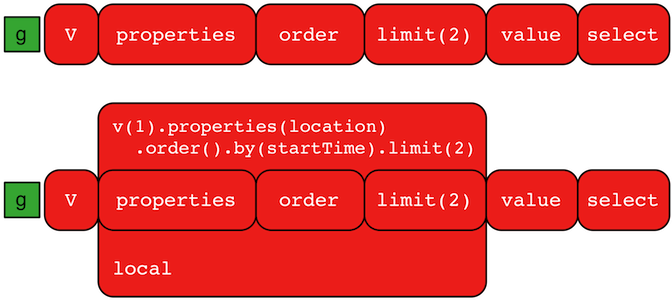A `GraphTraversal` operates on a continuous stream of objects. In many situations, it is important to operate on a single element within that stream. To do such object-local traversal computations, `local()`-step exists (branch). Note that the examples below use the The Crew toy data set.

``````gremlin> g.V().as('person').
properties('location').order().by('startTime',asc).limit(2).value().as('location').
select('person','location').by('name').by() //1\
==>[person:daniel,location:spremberg]
==>[person:stephen,location:centreville]
gremlin> g.V().as('person').
local(properties('location').order().by('startTime',asc).limit(2)).value().as('location').
select('person','location').by('name').by() //2\
==>[person:marko,location:san diego]
==>[person:marko,location:santa cruz]
==>[person:stephen,location:centreville]
==>[person:stephen,location:dulles]
==>[person:matthias,location:bremen]
==>[person:matthias,location:baltimore]
==>[person:daniel,location:spremberg]
==>[person:daniel,location:kaiserslautern]``````
``````g.V().as('person').
properties('location').order().by('startTime',asc).limit(2).value().as('location').
select('person','location').by('name').by() //1\
g.V().as('person').
local(properties('location').order().by('startTime',asc).limit(2)).value().as('location').
select('person','location').by('name').by() //2``````
1. Get the first two people and their respective location according to the most historic location start time.

2. For every person, get their two most historic locations.

The two traversals above look nearly identical save the inclusion of `local()` which wraps a section of the traversal in a object-local traversal. As such, the `order().by()` and the `limit()` refer to a particular object, not to the stream as a whole.

Local Step is quite similar in functionality to Flat Map Step where it can often be confused. `local()` propagates the traverser through the internal traversal as is without splitting/cloning it. Thus, its a “global traversal” with local processing. Its use is subtle and primarily finds application in compilation optimizations (i.e. when writing `TraversalStrategy` implementations. As another example consider:

``````gremlin> g.V().both().barrier().flatMap(groupCount().by("name"))
==>[lop:1]
==>[lop:1]
==>[lop:1]
==>[josh:1]
==>[josh:1]
==>[josh:1]
==>[marko:1]
==>[marko:1]
==>[marko:1]
==>[peter:1]
==>[ripple:1]
gremlin> g.V().both().barrier().local(groupCount().by("name"))
==>[lop:3]
==>[josh:3]
==>[marko:3]
==>[peter:1]
==>[ripple:1]``````
``````g.V().both().barrier().flatMap(groupCount().by("name"))
g.V().both().barrier().local(groupCount().by("name"))``````
 Warning The anonymous traversal of `local()` processes the current object "locally." In OLAP, where the atomic unit of computing is the vertex and its local "star graph," it is important that the anonymous traversal does not leave the confines of the vertex’s star graph. In other words, it can not traverse to an adjacent vertex’s properties or edges.

### Loops Step

The `loops()`-step (map) extracts the number of times the `Traverser` has gone through the current loop.

``````gremlin> g.V().emit(__.has("name", "marko").or().loops().is(2)).repeat(__.out()).values("name")
==>marko
==>ripple
==>lop``````
``g.V().emit(__.has("name", "marko").or().loops().is(2)).repeat(__.out()).values("name")``

### Match Step

The `match()`-step (map) provides a more declarative form of graph querying based on the notion of pattern matching. With `match()`, the user provides a collection of "traversal fragments," called patterns, that have variables defined that must hold true throughout the duration of the `match()`. When a traverser is in `match()`, a registered `MatchAlgorithm` analyzes the current state of the traverser (i.e. its history based on its path data), the runtime statistics of the traversal patterns, and returns a traversal-pattern that the traverser should try next. The default `MatchAlgorithm` provided is called `CountMatchAlgorithm` and it dynamically revises the pattern execution plan by sorting the patterns according to their filtering capabilities (i.e. largest set reduction patterns execute first). For very large graphs, where the developer is uncertain of the statistics of the graph (e.g. how many `knows`-edges vs. `worksFor`-edges exist in the graph), it is advantageous to use `match()`, as an optimal plan will be determined automatically. Furthermore, some queries are much easier to express via `match()` than with single-path traversals.

`"Who created a project named 'lop' that was also created by someone who is 29 years old? Return the two creators."`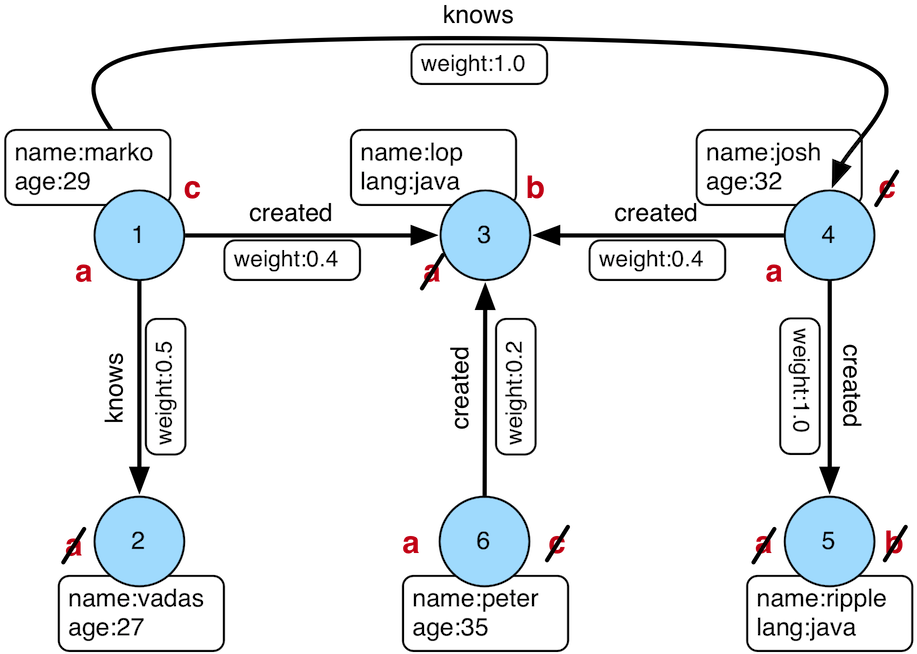``````gremlin> g.V().match(
__.as('a').out('created').as('b'),
__.as('b').has('name', 'lop'),
__.as('b').in('created').as('c'),
__.as('c').has('age', 29)).
select('a','c').by('name')
==>[a:marko,c:marko]
==>[a:josh,c:marko]
==>[a:peter,c:marko]``````
``````g.V().match(
__.as('a').out('created').as('b'),
__.as('b').has('name', 'lop'),
__.as('b').in('created').as('c'),
__.as('c').has('age', 29)).
select('a','c').by('name')``````

Note that the above can also be more concisely written as below which demonstrates that standard inner-traversals can be arbitrarily defined.

``````gremlin> g.V().match(
__.as('a').out('created').has('name', 'lop').as('b'),
__.as('b').in('created').has('age', 29).as('c')).
select('a','c').by('name')
==>[a:marko,c:marko]
==>[a:josh,c:marko]
==>[a:peter,c:marko]``````
``````g.V().match(
__.as('a').out('created').has('name', 'lop').as('b'),
__.as('b').in('created').has('age', 29).as('c')).
select('a','c').by('name')``````

In order to improve readability, `as()`-steps can be given meaningful labels which better reflect your domain. The previous query can thus be written in a more expressive way as shown below.

``````gremlin> g.V().match(
__.as('creators').out('created').has('name', 'lop').as('projects'), //1\
__.as('projects').in('created').has('age', 29).as('cocreators')). //2\
select('creators','cocreators').by('name') //3\
==>[creators:marko,cocreators:marko]
==>[creators:josh,cocreators:marko]
==>[creators:peter,cocreators:marko]``````
``````g.V().match(
__.as('creators').out('created').has('name', 'lop').as('projects'), //1\
__.as('projects').in('created').has('age', 29).as('cocreators')). //2\
select('creators','cocreators').by('name') //3``````
1. Find vertices that created something and match them as 'creators', then find out what they created which is named 'lop' and match these vertices as 'projects'.

2. Using these 'projects' vertices, find out their creators aged 29 and remember these as 'cocreators'.

3. Return the name of both 'creators' and 'cocreators'.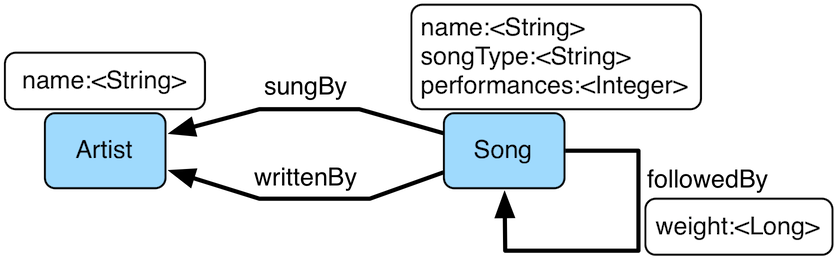`MatchStep` brings functionality similar to SPARQL to Gremlin. Like SPARQL, MatchStep conjoins a set of patterns applied to a graph. For example, the following traversal finds exactly those songs which Jerry Garcia has both sung and written (using the Grateful Dead graph distributed in the `data/` directory):

``````gremlin> graph.io(graphml()).readGraph('data/grateful-dead.xml')
gremlin> g = graph.traversal()
==>graphtraversalsource[tinkergraph[vertices:808 edges:8049], standard]
gremlin> g.V().match(
__.as('a').has('name', 'Garcia'),
__.as('a').in('writtenBy').as('b'),
__.as('a').in('sungBy').as('b')).
select('b').values('name')
==>CREAM PUFF WAR
==>CRYPTICAL ENVELOPMENT``````
``````graph.io(graphml()).readGraph('data/grateful-dead.xml')
g = graph.traversal()
g.V().match(
__.as('a').has('name', 'Garcia'),
__.as('a').in('writtenBy').as('b'),
__.as('a').in('sungBy').as('b')).
select('b').values('name')``````

Among the features which differentiate `match()` from SPARQL are:

``````gremlin> g.V().match(
__.as('a').out('created').has('name','lop').as('b'), //1\
__.as('b').in('created').has('age', 29).as('c'),
__.as('c').repeat(out()).times(2)). //2\
select('c').out('knows').dedup().values('name') //3\
==>josh``````
``````g.V().match(
__.as('a').out('created').has('name','lop').as('b'), //1\
__.as('b').in('created').has('age', 29).as('c'),
__.as('c').repeat(out()).times(2)). //2\
select('c').out('knows').dedup().values('name') //3``````
1. Patterns of arbitrary complexity: `match()` is not restricted to triple patterns or property paths.

2. Recursion support: `match()` supports the branch-based steps within a pattern, including `repeat()`.

3. Imperative/declarative hybrid: Before and after a `match()`, it is possible to leverage classic Gremlin traversals.

To extend point #3, it is possible to support going from imperative, to declarative, to imperative, ad infinitum.

``````gremlin> g.V().match(
__.as('a').out('knows').as('b'),
__.as('b').out('created').has('name','lop')).
select('b').out('created').
match(
__.as('x').in('created').as('y'),
__.as('y').out('knows').as('z')).
select('z').values('name')
==>josh``````
``````g.V().match(
__.as('a').out('knows').as('b'),
__.as('b').out('created').has('name','lop')).
select('b').out('created').
match(
__.as('x').in('created').as('y'),
__.as('y').out('knows').as('z')).
select('z').values('name')``````
 Important The `match()`-step is stateless. The variable bindings of the traversal patterns are stored in the path history of the traverser. As such, the variables used over all `match()`-steps within a traversal are globally unique. A benefit of this is that subsequent `where()`, `select()`, `match()`, etc. steps can leverage the same variables in their analysis.

Like all other steps in Gremlin, `match()` is a function and thus, `match()` within `match()` is a natural consequence of Gremlin’s functional foundation (i.e. recursive matching).

``````gremlin> g.V().match(
__.as('a').out('knows').as('b'),
__.as('b').out('created').has('name','lop'),
__.as('b').match(
__.as('b').out('created').as('c'),
__.as('c').has('name','ripple')).
select('c').as('c')).
select('a','c').by('name')
==>[a:marko,c:ripple]``````
``````g.V().match(
__.as('a').out('knows').as('b'),
__.as('b').out('created').has('name','lop'),
__.as('b').match(
__.as('b').out('created').as('c'),
__.as('c').has('name','ripple')).
select('c').as('c')).
select('a','c').by('name')``````

If a step-labeled traversal proceeds the `match()`-step and the traverser entering the `match()` is destined to bind to a particular variable, then the previous step should be labeled accordingly.

``````gremlin> g.V().as('a').out('knows').as('b').
match(
__.as('b').out('created').as('c'),
__.not(__.as('c').in('created').as('a'))).
select('a','b','c').by('name')
==>[a:marko,b:josh,c:ripple]``````
``````g.V().as('a').out('knows').as('b').
match(
__.as('b').out('created').as('c'),
__.not(__.as('c').in('created').as('a'))).
select('a','b','c').by('name')``````

There are three types of `match()` traversal patterns.

1. `as('a')…​as('b')`: both the start and end of the traversal have a declared variable.

2. `as('a')…​`: only the start of the traversal has a declared variable.

3. `…​`: there are no declared variables.

If a variable is at the start of a traversal pattern it must exist as a label in the path history of the traverser else the traverser can not go down that path. If a variable is at the end of a traversal pattern then if the variable exists in the path history of the traverser, the traverser’s current location must match (i.e. equal) its historic location at that same label. However, if the variable does not exist in the path history of the traverser, then the current location is labeled as the variable and thus, becomes a bound variable for subsequent traversal patterns. If a traversal pattern does not have an end label, then the traverser must simply "survive" the pattern (i.e. not be filtered) to continue to the next pattern. If a traversal pattern does not have a start label, then the traverser can go down that path at any point, but will only go down that pattern once as a traversal pattern is executed once and only once for the history of the traverser. Typically, traversal patterns that do not have a start and end label are used in conjunction with `and()`, `or()`, and `where()`. Once the traverser has "survived" all the patterns (or at least one for `or()`), `match()`-step analyzes the traverser’s path history and emits a `Map<String,Object>` of the variable bindings to the next step in the traversal.

``````gremlin> g.V().as('a').out().as('b'). //1\
match( //2\
__.as('a').out().count().as('c'), //3\
__.not(__.as('a').in().as('b')), //4\
or( //5\
__.as('a').out('knows').as('b'),
__.as('b').in().count().as('c').and().as('c').is(gt(2)))). //6\
dedup('a','c'). //7\
select('a','b','c').by('name').by('name').by() //8\
==>[a:marko,b:lop,c:3]``````
``````g.V().as('a').out().as('b'). //1\
match( //2\
__.as('a').out().count().as('c'), //3\
__.not(__.as('a').in().as('b')), //4\
or( //5\
__.as('a').out('knows').as('b'),
__.as('b').in().count().as('c').and().as('c').is(gt(2)))). //6\
dedup('a','c'). //7\
select('a','b','c').by('name').by('name').by() //8``````
1. A standard, step-labeled traversal can come prior to `match()`.

2. If the traverser’s path prior to entering `match()` has requisite label values, then those historic values are bound.

3. It is possible to use barrier steps though they are computed locally to the pattern (as one would expect).

4. It is possible to `not()` a pattern.

5. It is possible to nest `and()`- and `or()`-steps for conjunction matching.

6. Both infix and prefix conjunction notation is supported.

7. It is possible to "distinct" the specified label combination.

8. The bound values are of different types — vertex ("a"), vertex ("b"), long ("c").

#### Using Where with Match

Match is typically used in conjunction with both `select()` (demonstrated previously) and `where()` (presented here). A `where()`-step allows the user to further constrain the result set provided by `match()`.

``````gremlin> g.V().match(
__.as('a').out('created').as('b'),
__.as('b').in('created').as('c')).
where('a', neq('c')).
select('a','c').by('name')
==>[a:marko,c:josh]
==>[a:marko,c:peter]
==>[a:josh,c:marko]
==>[a:josh,c:peter]
==>[a:peter,c:marko]
==>[a:peter,c:josh]``````
``````g.V().match(
__.as('a').out('created').as('b'),
__.as('b').in('created').as('c')).
where('a', neq('c')).
select('a','c').by('name')``````

The `where()`-step can take either a `P`-predicate (example above) or a `Traversal` (example below). Using `MatchPredicateStrategy`, `where()`-clauses are automatically folded into `match()` and thus, subject to the query optimizer within `match()`-step.

``````gremlin> traversal = g.V().match(
__.as('a').has(label,'person'), //1\
__.as('a').out('created').as('b'),
__.as('b').in('created').as('c')).
where(__.as('a').out('knows').as('c')). //2\
select('a','c').by('name'); null //3\
gremlin> traversal.toString() //4\
==>[GraphStep(vertex,[]), MatchStep(AND,[[MatchStartStep(a), HasStep([~label.eq(person)]), MatchEndStep], [MatchStartStep(a), VertexStep(OUT,[created],vertex), MatchEndStep(b)], [MatchStartStep(b), VertexStep(IN,[created],vertex), MatchEndStep(c)]]), WhereTraversalStep([WhereStartStep(a), VertexStep(OUT,[knows],vertex), WhereEndStep(c)]), SelectStep(last,[a, c],[value(name)])]
gremlin> traversal // (5) (6)
==>[a:marko,c:josh]
gremlin> traversal.toString() //7\
==>[TinkerGraphStep(vertex,[~label.eq(person)])@[a], MatchStep(AND,[[MatchStartStep(a), VertexStep(OUT,[created],vertex), MatchEndStep(b)], [MatchStartStep(b), VertexStep(IN,[created],vertex), MatchEndStep(c)], [MatchStartStep(a), WhereTraversalStep([WhereStartStep, VertexStep(OUT,[knows],vertex), WhereEndStep(c)]), MatchEndStep]]), SelectStep(last,[a, c],[value(name)])]``````
``````traversal = g.V().match(
__.as('a').has(label,'person'), //1\
__.as('a').out('created').as('b'),
__.as('b').in('created').as('c')).
where(__.as('a').out('knows').as('c')). //2\
select('a','c').by('name'); null //3\
traversal.toString() //4\
traversal // (5) (6) (5)
traversal.toString() //7``````
1. Any `has()`-step traversal patterns that start with the match-key are pulled out of `match()` to enable the graph system to leverage the filter for index lookups.

2. A `where()`-step with a traversal containing variable bindings declared in `match()`.

3. A useful trick to ensure that the traversal is not iterated by Gremlin Console.

4. The string representation of the traversal prior to its strategies being applied.

5. The Gremlin Console will automatically iterate anything that is an iterator or is iterable.

6. Both marko and josh are co-developers and marko knows josh.

7. The string representation of the traversal after the strategies have been applied (and thus, `where()` is folded into `match()`)

 Important A `where()`-step is a filter and thus, variables within a `where()` clause are not globally bound to the path of the traverser in `match()`. As such, `where()`-steps in `match()` are used for filtering, not binding.

### Math Step

The `math()`-step (math) enables scientific calculator functionality within Gremlin. This step deviates from the common function composition and nesting formalisms to provide an easy to read string-based math processor. Variables within the equation map to scopes in Gremlin — e.g. path labels, side-effects, or incoming map keys. This step supports `by()`-modulation where the `by()`-modulators are applied in the order in which the variables are first referenced within the equation. Note that the reserved variable `_` refers to the current numeric traverser object incoming to the `math()`-step.

``````gremlin> g.V().as('a').out('knows').as('b').math('a + b').by('age')
==>56.0
==>61.0
gremlin> g.V().as('a').out('created').as('b').
math('b + a').
by(both().count().math('_ + 100')).
by('age')
==>132.0
==>133.0
==>135.0
==>138.0
gremlin> g.withSideEffect('x',10).V().values('age').math('_ / x')
==>2.9
==>2.7
==>3.2
==>3.5
gremlin> g.withSack(1).V(1).repeat(sack(sum).by(constant(1))).times(10).emit().sack().math('sin _')
==>0.9092974268256817
==>0.1411200080598672
==>-0.7568024953079282
==>-0.9589242746631385
==>-0.27941549819892586
==>0.6569865987187891
==>0.9893582466233818
==>0.4121184852417566
==>-0.5440211108893698
==>-0.9999902065507035``````
``````g.V().as('a').out('knows').as('b').math('a + b').by('age')
g.V().as('a').out('created').as('b').
math('b + a').
by(both().count().math('_ + 100')).
by('age')
g.withSideEffect('x',10).V().values('age').math('_ / x')
g.withSack(1).V(1).repeat(sack(sum).by(constant(1))).times(10).emit().sack().math('sin _')``````

The operators supported by the calculator include: `*`, `+`, `\`, `^`, and `%`. Furthermore, the following built in functions are provided:

• `abs`: absolute value

• `acos`: arc cosine

• `asin`: arc sine

• `atan`: arc tangent

• `cbrt`: cubic root

• `ceil`: nearest upper integer

• `cos`: cosine

• `cosh`: hyperbolic cosine

• `exp`: euler’s number raised to the power (`e^x`)

• `floor`: nearest lower integer

• `log`: logarithmus naturalis (base e)

• `log10`: logarithm (base 10)

• `log2`: logarithm (base 2)

• `sin`: sine

• `sinh`: hyperbolic sine

• `sqrt`: square root

• `tan`: tangent

• `tanh`: hyperbolic tangent

• `signum`: signum function

### Max Step

The `max()`-step (map) operates on a stream of numbers and determines which is the largest number in the stream.

``````gremlin> g.V().values('age').max()
==>35
gremlin> g.V().repeat(both()).times(3).values('age').max()
==>35``````
``````g.V().values('age').max()
g.V().repeat(both()).times(3).values('age').max()``````
 Important `max(local)` determines the max of the current, local object (not the objects in the traversal stream). This works for `Collection` and `Number`-type objects. For any other object, a max of `Double.NaN` is returned.

### Mean Step

The `mean()`-step (map) operates on a stream of numbers and determines the average of those numbers.

``````gremlin> g.V().values('age').mean()
==>30.75
gremlin> g.V().repeat(both()).times(3).values('age').mean() //1\
==>30.645833333333332
gremlin> g.V().repeat(both()).times(3).values('age').dedup().mean()
==>30.75``````
``````g.V().values('age').mean()
g.V().repeat(both()).times(3).values('age').mean() //1\
g.V().repeat(both()).times(3).values('age').dedup().mean()``````
1. Realize that traversers are being bulked by `repeat()`. There may be more of a particular number than another, thus altering the average.

 Important `mean(local)` determines the mean of the current, local object (not the objects in the traversal stream). This works for `Collection` and `Number`-type objects. For any other object, a mean of `Double.NaN` is returned.

### Min Step

The `min()`-step (map) operates on a stream of numbers and determines which is the smallest number in the stream.

``````gremlin> g.V().values('age').min()
==>27
gremlin> g.V().repeat(both()).times(3).values('age').min()
==>27``````
``````g.V().values('age').min()
g.V().repeat(both()).times(3).values('age').min()``````
 Important `min(local)` determines the min of the current, local object (not the objects in the traversal stream). This works for `Collection` and `Number`-type objects. For any other object, a min of `Double.NaN` is returned.

### None Step

The `none()`-step (filter) filters all objects from a traversal stream. It is especially useful for to traversals that are executed remotely where returning results is not useful and the traversal is only meant to generate side-effects. Choosing not to return results saves in serialization and network costs as the objects are filtered on the remote end and not returned to the client side. Typically, this step does not need to be used directly and is quietly used by the `iterate()` terminal step which appends `none()` to the traversal before actually cycling through results.

### Not Step

The `not()`-step (filter) removes objects from the traversal stream when the traversal provided as an argument returns an object.

 Groovy The term `not` is a reserved word in Groovy, and when therefore used as part of an anonymous traversal must be referred to in Gremlin with the double underscore `__.not()`.
 Python The term `not` is a reserved word in Python, and therefore must be referred to in Gremlin with `not_()`.
``````gremlin> g.V().not(hasLabel('person')).valueMap(true)
==>[id:3,label:software,name:[lop],lang:[java]]
==>[id:5,label:software,name:[ripple],lang:[java]]
gremlin> g.V().hasLabel('person').
not(out('created').count().is(gt(1))).values('name') //1\
==>marko
==>peter``````
``````g.V().not(hasLabel('person')).valueMap(true)
g.V().hasLabel('person').
not(out('created').count().is(gt(1))).values('name')   //1``````
1. josh created two projects and vadas none

### Optional Step

The `optional()`-step (branch/flatMap) returns the result of the specified traversal if it yields a result else it returns the calling element, i.e. the `identity()`.

``````gremlin> g.V(2).optional(out('knows')) //1\
==>v
gremlin> g.V(2).optional(__.in('knows')) //2\
==>v``````
``````g.V(2).optional(out('knows')) //1\
g.V(2).optional(__.in('knows')) //2``````
1. vadas does not have an outgoing knows-edge so vadas is returned.

2. vadas does have an incoming knows-edge so marko is returned.

`optional` is particularly useful for lifting entire graphs when used in conjunction with `path` or `tree`.

``````gremlin> g.V().hasLabel('person').optional(out('knows').optional(out('created'))).path() //1\
==>[v,v]
==>[v,v,v]
==>[v,v,v]
==>[v]
==>[v]
==>[v]``````
``g.V().hasLabel('person').optional(out('knows').optional(out('created'))).path() //1``
1. Returns the paths of everybody followed by who they know followed by what they created.

### Or Step

The `or()`-step ensures that at least one of the provided traversals yield a result (filter). Please see `and()` for and-semantics.

 Python The term `or` is a reserved word in Python, and therefore must be referred to in Gremlin with `or_()`.
``````gremlin> g.V().or(
__.outE('created'),
__.inE('created').count().is(gt(1))).
values('name')
==>marko
==>lop
==>josh
==>peter``````
``````g.V().or(
__.outE('created'),
__.inE('created').count().is(gt(1))).
values('name')``````

The `or()`-step can take an arbitrary number of traversals. At least one of the traversals must produce at least one output for the original traverser to pass to the next step.

An infix notation can be used as well. Though, with infix notation, only two traversals can be or’d together.

``````gremlin> g.V().where(outE('created').or().outE('knows')).values('name')
==>marko
==>josh
==>peter``````
``g.V().where(outE('created').or().outE('knows')).values('name')``

### Order Step

When the objects of the traversal stream need to be sorted, `order()`-step (map) can be leveraged.

``````gremlin> g.V().values('name').order()
==>josh
==>lop
==>marko
==>peter
==>ripple
gremlin> g.V().values('name').order().by(desc)
==>ripple
==>peter
==>marko
==>lop
==>josh
gremlin> g.V().hasLabel('person').order().by('age', asc).values('name')
==>marko
==>josh
==>peter``````
``````g.V().values('name').order()
g.V().values('name').order().by(desc)
g.V().hasLabel('person').order().by('age', asc).values('name')``````

One of the most traversed objects in a traversal is an `Element`. An element can have properties associated with it (i.e. key/value pairs). In many situations, it is desirable to sort an element traversal stream according to a comparison of their properties.

``````gremlin> g.V().values('name')
==>marko
==>lop
==>josh
==>ripple
==>peter
gremlin> g.V().order().by('name',asc).values('name')
==>josh
==>lop
==>marko
==>peter
==>ripple
gremlin> g.V().order().by('name',desc).values('name')
==>ripple
==>peter
==>marko
==>lop
==>josh``````
``````g.V().values('name')
g.V().order().by('name',asc).values('name')
g.V().order().by('name',desc).values('name')``````

The `order()`-step allows the user to provide an arbitrary number of comparators for primary, secondary, etc. sorting. In the example below, the primary ordering is based on the outgoing created-edge count. The secondary ordering is based on the age of the person.

``````gremlin> g.V().hasLabel('person').order().by(outE('created').count(), asc).
by('age', asc).values('name')
==>marko
==>peter
==>josh
gremlin> g.V().hasLabel('person').order().by(outE('created').count(), asc).
by('age', desc).values('name')
==>peter
==>marko
==>josh``````
``````g.V().hasLabel('person').order().by(outE('created').count(), asc).
by('age', asc).values('name')
g.V().hasLabel('person').order().by(outE('created').count(), asc).
by('age', desc).values('name')``````

Randomizing the order of the traversers at a particular point in the traversal is possible with `Order.shuffle`.

``````gremlin> g.V().hasLabel('person').order().by(shuffle)
==>v
==>v
==>v
==>v
gremlin> g.V().hasLabel('person').order().by(shuffle)
==>v
==>v
==>v
==>v``````
``````g.V().hasLabel('person').order().by(shuffle)
g.V().hasLabel('person').order().by(shuffle)``````

It is possible to use `order(local)` to order the current local object and not the entire traversal stream. This works for `Collection`- and `Map`-type objects. For any other object, the object is returned unchanged.

``````gremlin> g.V().values('age').fold().order(local).by(desc) //1\
==>[35,32,29,27]
gremlin> g.V().values('age').order(local).by(desc) //2\
==>29
==>27
==>32
==>35
gremlin> g.V().groupCount().by(inE().count()).order(local).by(values, desc) //3\
==>[1:3,0:2,3:1]
gremlin> g.V().groupCount().by(inE().count()).order(local).by(keys, asc) //4\
==>[0:2,1:3,3:1]``````
``````g.V().values('age').fold().order(local).by(desc) //1\
g.V().values('age').order(local).by(desc) //2\
g.V().groupCount().by(inE().count()).order(local).by(values, desc) //3\
g.V().groupCount().by(inE().count()).order(local).by(keys, asc) //4``````
1. The ages are gathered into a list and then that list is sorted in decreasing order.

2. The ages are not gathered and thus `order(local)` is "ordering" single integers and thus, does nothing.

3. The `groupCount()` map is ordered by its values in decreasing order.

4. The `groupCount()` map is ordered by its keys in increasing order.

 Note The `values` and `keys` enums are from `Column` which is used to select "columns" from a `Map`, `Map.Entry`, or `Path`.
 Note Prior to version 3.3.4, ordering was defined by `Order.incr` for ascending order and `Order.decr` for descending order. That approach is now deprecated with the preferred method shown in the examples which uses the more common forms for query languages in `Order.asc` and Order.desc.

### PageRank Step

The `pageRank()`-step (map/sideEffect) calculates PageRank using `PageRankVertexProgram`.

 Important The `pageRank()`-step is a `VertexComputing`-step and as such, can only be used against a graph that supports `GraphComputer` (OLAP).
``````gremlin> g = graph.traversal().withComputer()
==>graphtraversalsource[tinkergraph[vertices:6 edges:6], graphcomputer]
gremlin> g.V().pageRank().by('pageRank').values('pageRank')
==>0.11375510357865541
==>0.11375510357865541
==>0.14598540152719106
==>0.14598540152719106
==>0.3047200907912249
==>0.17579889899708231
gremlin> g.V().hasLabel('person').
pageRank().
by(outE('knows')).
by('friendRank').
order().by('friendRank',desc).valueMap('name','friendRank')
==>[friendRank:[0.8321166533236799],name:[josh]]
==>[friendRank:[0.5839416733381598],name:[marko]]
==>[friendRank:[0.5839416733381598],name:[peter]]``````
``````g = graph.traversal().withComputer()
g.V().pageRank().by('pageRank').values('pageRank')
g.V().hasLabel('person').
pageRank().
by(outE('knows')).
by('friendRank').
order().by('friendRank',desc).valueMap('name','friendRank')``````

The `explain()`-step can be used to understand how the traversal is compiled into multiple `GraphComputer` jobs.

``````gremlin> g = graph.traversal().withComputer()
==>graphtraversalsource[tinkergraph[vertices:6 edges:6], graphcomputer]
gremlin> g.V().hasLabel('person').
pageRank().
by(outE('knows')).
by('friendRank').
order().by('friendRank',desc).valueMap('name','friendRank').explain()
==>Traversal Explanation
=============================================================================================================================================================================================================================================
Original Traversal                    [GraphStep(vertex,[]), HasStep([~label.eq(person)]), PageRankVertexProgramStep([VertexStep(OUT,[knows],edge)],friendRank,20,graphfilter[none]), OrderGlobalStep([[value(friendRank), desc]]), PropertyM
apStep([name, friendRank],value)]

ConnectiveStrategy              [D]   [GraphStep(vertex,[]), HasStep([~label.eq(person)]), PageRankVertexProgramStep([VertexStep(OUT,[knows],edge)],friendRank,20,graphfilter[none]), OrderGlobalStep([[value(friendRank), desc]]), PropertyM
apStep([name, friendRank],value)]
VertexProgramStrategy           [D]   [TraversalVertexProgramStep([GraphStep(vertex,[]), HasStep([~label.eq(person)])],graphfilter[none]), PageRankVertexProgramStep([VertexStep(OUT,[knows],edge)],friendRank,20,graphfilter[none]), Travers
alVertexProgramStep([OrderGlobalStep([[value(friendRank), desc]]), PropertyMapStep([name, friendRank],value)],graphfilter[none]), ComputerResultStep]
EarlyLimitStrategy              [O]   [TraversalVertexProgramStep([GraphStep(vertex,[]), HasStep([~label.eq(person)])],graphfilter[none]), PageRankVertexProgramStep([VertexStep(OUT,[knows],edge)],friendRank,20,graphfilter[none]), Travers
alVertexProgramStep([OrderGlobalStep([[value(friendRank), desc]]), PropertyMapStep([name, friendRank],value)],graphfilter[none]), ComputerResultStep]
MatchPredicateStrategy          [O]   [TraversalVertexProgramStep([GraphStep(vertex,[]), HasStep([~label.eq(person)])],graphfilter[none]), PageRankVertexProgramStep([VertexStep(OUT,[knows],edge)],friendRank,20,graphfilter[none]), Travers
alVertexProgramStep([OrderGlobalStep([[value(friendRank), desc]]), PropertyMapStep([name, friendRank],value)],graphfilter[none]), ComputerResultStep]
FilterRankingStrategy           [O]   [TraversalVertexProgramStep([GraphStep(vertex,[]), HasStep([~label.eq(person)])],graphfilter[none]), PageRankVertexProgramStep([VertexStep(OUT,[knows],edge)],friendRank,20,graphfilter[none]), Travers
alVertexProgramStep([OrderGlobalStep([[value(friendRank), desc]]), PropertyMapStep([name, friendRank],value)],graphfilter[none]), ComputerResultStep]
PathProcessorStrategy           [O]   [TraversalVertexProgramStep([GraphStep(vertex,[]), HasStep([~label.eq(person)])],graphfilter[none]), PageRankVertexProgramStep([VertexStep(OUT,[knows],edge)],friendRank,20,graphfilter[none]), Travers
alVertexProgramStep([OrderGlobalStep([[value(friendRank), desc]]), PropertyMapStep([name, friendRank],value)],graphfilter[none]), ComputerResultStep]
InlineFilterStrategy            [O]   [TraversalVertexProgramStep([GraphStep(vertex,[]), HasStep([~label.eq(person)])],graphfilter[none]), PageRankVertexProgramStep([VertexStep(OUT,[knows],edge)],friendRank,20,graphfilter[none]), Travers
alVertexProgramStep([OrderGlobalStep([[value(friendRank), desc]]), PropertyMapStep([name, friendRank],value)],graphfilter[none]), ComputerResultStep]
IncidentToAdjacentStrategy      [O]   [TraversalVertexProgramStep([GraphStep(vertex,[]), HasStep([~label.eq(person)])],graphfilter[none]), PageRankVertexProgramStep([VertexStep(OUT,[knows],edge)],friendRank,20,graphfilter[none]), Travers
alVertexProgramStep([OrderGlobalStep([[value(friendRank), desc]]), PropertyMapStep([name, friendRank],value)],graphfilter[none]), ComputerResultStep]
AdjacentToIncidentStrategy      [O]   [TraversalVertexProgramStep([GraphStep(vertex,[]), HasStep([~label.eq(person)])],graphfilter[none]), PageRankVertexProgramStep([VertexStep(OUT,[knows],edge)],friendRank,20,graphfilter[none]), Travers
alVertexProgramStep([OrderGlobalStep([[value(friendRank), desc]]), PropertyMapStep([name, friendRank],value)],graphfilter[none]), ComputerResultStep]
RepeatUnrollStrategy            [O]   [TraversalVertexProgramStep([GraphStep(vertex,[]), HasStep([~label.eq(person)])],graphfilter[none]), PageRankVertexProgramStep([VertexStep(OUT,[knows],edge)],friendRank,20,graphfilter[none]), Travers
alVertexProgramStep([OrderGlobalStep([[value(friendRank), desc]]), PropertyMapStep([name, friendRank],value)],graphfilter[none]), ComputerResultStep]
CountStrategy                   [O]   [TraversalVertexProgramStep([GraphStep(vertex,[]), HasStep([~label.eq(person)])],graphfilter[none]), PageRankVertexProgramStep([VertexStep(OUT,[knows],edge)],friendRank,20,graphfilter[none]), Travers
alVertexProgramStep([OrderGlobalStep([[value(friendRank), desc]]), PropertyMapStep([name, friendRank],value)],graphfilter[none]), ComputerResultStep]
PathRetractionStrategy          [O]   [TraversalVertexProgramStep([GraphStep(vertex,[]), HasStep([~label.eq(person)])],graphfilter[none]), PageRankVertexProgramStep([VertexStep(OUT,[knows],edge)],friendRank,20,graphfilter[none]), Travers
alVertexProgramStep([OrderGlobalStep([[value(friendRank), desc]]), PropertyMapStep([name, friendRank],value)],graphfilter[none]), ComputerResultStep]
LazyBarrierStrategy             [O]   [TraversalVertexProgramStep([GraphStep(vertex,[]), HasStep([~label.eq(person)])],graphfilter[none]), PageRankVertexProgramStep([VertexStep(OUT,[knows],edge)],friendRank,20,graphfilter[none]), Travers
alVertexProgramStep([OrderGlobalStep([[value(friendRank), desc]]), PropertyMapStep([name, friendRank],value)],graphfilter[none]), ComputerResultStep]
MessagePassingReductionStrategy [O]   [TraversalVertexProgramStep([GraphStep(vertex,[]), HasStep([~label.eq(person)])],graphfilter[none]), PageRankVertexProgramStep([VertexStep(OUT,[knows],edge)],friendRank,20,graphfilter[none]), Travers
alVertexProgramStep([OrderGlobalStep([[value(friendRank), desc]]), PropertyMapStep([name, friendRank],value)],graphfilter[none]), ComputerResultStep]
OrderLimitStrategy              [O]   [TraversalVertexProgramStep([GraphStep(vertex,[]), HasStep([~label.eq(person)])],graphfilter[none]), PageRankVertexProgramStep([VertexStep(OUT,[knows],edge)],friendRank,20,graphfilter[none]), Travers
alVertexProgramStep([OrderGlobalStep([[value(friendRank), desc]]), PropertyMapStep([name, friendRank],value)],graphfilter[none]), ComputerResultStep]
TinkerGraphCountStrategy        [P]   [TraversalVertexProgramStep([GraphStep(vertex,[]), HasStep([~label.eq(person)])],graphfilter[none]), PageRankVertexProgramStep([VertexStep(OUT,[knows],edge)],friendRank,20,graphfilter[none]), Travers
alVertexProgramStep([OrderGlobalStep([[value(friendRank), desc]]), PropertyMapStep([name, friendRank],value)],graphfilter[none]), ComputerResultStep]
TinkerGraphStepStrategy         [P]   [TraversalVertexProgramStep([GraphStep(vertex,[]), HasStep([~label.eq(person)])],graphfilter[none]), PageRankVertexProgramStep([VertexStep(OUT,[knows],edge)],friendRank,20,graphfilter[none]), Travers
alVertexProgramStep([OrderGlobalStep([[value(friendRank), desc]]), PropertyMapStep([name, friendRank],value)],graphfilter[none]), ComputerResultStep]
ProfileStrategy                 [F]   [TraversalVertexProgramStep([GraphStep(vertex,[]), HasStep([~label.eq(person)])],graphfilter[none]), PageRankVertexProgramStep([VertexStep(OUT,[knows],edge)],friendRank,20,graphfilter[none]), Travers
alVertexProgramStep([OrderGlobalStep([[value(friendRank), desc]]), PropertyMapStep([name, friendRank],value)],graphfilter[none]), ComputerResultStep]
ComputerFinalizationStrategy    [T]   [TraversalVertexProgramStep([GraphStep(vertex,[]), HasStep([~label.eq(person)])],graphfilter[none]), PageRankVertexProgramStep([VertexStep(OUT,[knows],edge)],friendRank,20,graphfilter[none]), Travers
alVertexProgramStep([OrderGlobalStep([[value(friendRank), desc]]), PropertyMapStep([name, friendRank],value)],graphfilter[none]), ComputerResultStep]
ComputerVerificationStrategy    [V]   [TraversalVertexProgramStep([GraphStep(vertex,[]), HasStep([~label.eq(person)])],graphfilter[none]), PageRankVertexProgramStep([VertexStep(OUT,[knows],edge)],friendRank,20,graphfilter[none]), Travers
alVertexProgramStep([OrderGlobalStep([[value(friendRank), desc]]), PropertyMapStep([name, friendRank],value)],graphfilter[none]), ComputerResultStep]
StandardVerificationStrategy    [V]   [TraversalVertexProgramStep([GraphStep(vertex,[]), HasStep([~label.eq(person)])],graphfilter[none]), PageRankVertexProgramStep([VertexStep(OUT,[knows],edge)],friendRank,20,graphfilter[none]), Travers
alVertexProgramStep([OrderGlobalStep([[value(friendRank), desc]]), PropertyMapStep([name, friendRank],value)],graphfilter[none]), ComputerResultStep]

Final Traversal                       [TraversalVertexProgramStep([GraphStep(vertex,[]), HasStep([~label.eq(person)])],graphfilter[none]), PageRankVertexProgramStep([VertexStep(OUT,[knows],edge)],friendRank,20,graphfilter[none]), Travers
alVertexProgramStep([OrderGlobalStep([[value(friendRank), desc]]), PropertyMapStep([name, friendRank],value)],graphfilter[none]), ComputerResultStep]``````
``````g = graph.traversal().withComputer()
g.V().hasLabel('person').
pageRank().
by(outE('knows')).
by('friendRank').
order().by('friendRank',desc).valueMap('name','friendRank').explain()``````

### Path Step

A traverser is transformed as it moves through a series of steps within a traversal. The history of the traverser is realized by examining its path with `path()`-step (map).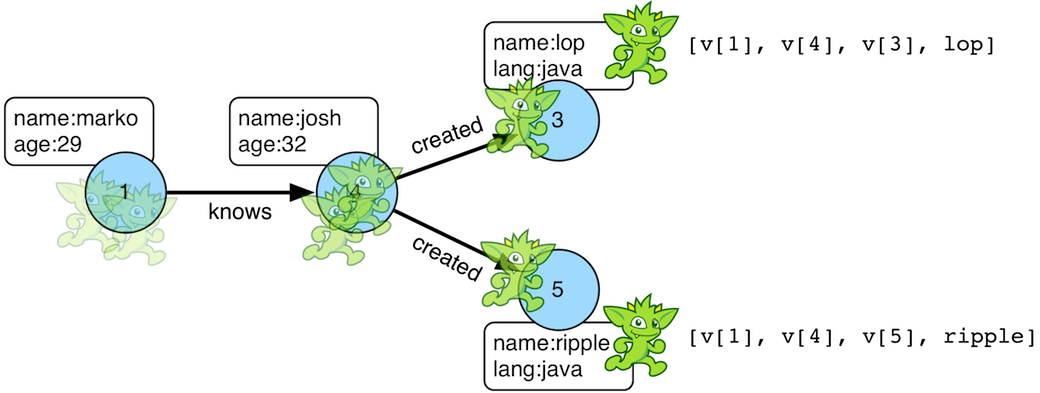``````gremlin> g.V().out().out().values('name')
==>ripple
==>lop
gremlin> g.V().out().out().values('name').path()
==>[v,v,v,ripple]
==>[v,v,v,lop]``````
``````g.V().out().out().values('name')
g.V().out().out().values('name').path()``````

If edges are required in the path, then be sure to traverser those edges explicitly.

``````gremlin> g.V().outE().inV().outE().inV().path()
==>[v,e[1-knows->4],v,e[4-created->5],v]
==>[v,e[1-knows->4],v,e[4-created->3],v]``````
``g.V().outE().inV().outE().inV().path()``

It is possible to post-process the elements of the path in a round-robin fashion via `by()`.

``````gremlin> g.V().out().out().path().by('name').by('age')
==>[marko,32,ripple]
==>[marko,32,lop]``````
``g.V().out().out().path().by('name').by('age')``

Finally, because `by()`-based post-processing, nothing prevents triggering yet another traversal. In the traversal below, for each element of the path traversed thus far, if its a person (as determined by having an `age`-property), then get all of their creations, else if its a creation, get all the people that created it.

``````gremlin> g.V().out().out().path().by(
choose(hasLabel('person'),
out('created').values('name'),
__.in('created').values('name')).fold())
==>[[lop],[ripple,lop],[josh]]
==>[[lop],[ripple,lop],[marko,josh,peter]]``````
``````g.V().out().out().path().by(
choose(hasLabel('person'),
out('created').values('name'),
__.in('created').values('name')).fold())``````
 Warning Generating path information is expensive as the history of the traverser is stored into a Java list. With numerous traversers, there are numerous lists. Moreover, in an OLAP `GraphComputer` environment this becomes exceedingly prohibitive as there are traversers emanating from all vertices in the graph in parallel. In OLAP there are optimizations provided for traverser populations, but when paths are calculated (and each traverser is unique due to its history), then these optimizations are no longer possible.

#### Path Data Structure

The `Path` data structure is an ordered list of objects, where each object is associated to a `Set<String>` of labels. An example is presented below to demonstrate both the `Path` API as well as how a traversal yields labeled paths.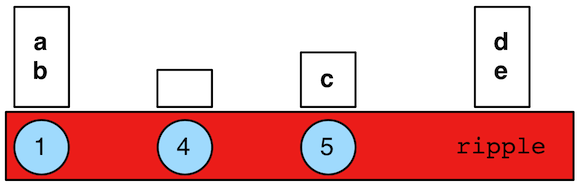``````gremlin> path = g.V(1).as('a').has('name').as('b').
out('knows').out('created').as('c').
has('name','ripple').values('name').as('d').
identity().as('e').path().next()
==>v
==>v
==>v
==>ripple
gremlin> path.size()
==>4
gremlin> path.objects()
==>v
==>v
==>v
==>ripple
gremlin> path.labels()
==>[b,a]
==>[]
==>[c]
==>[d,e]
gremlin> path.a
==>v
gremlin> path.b
==>v
gremlin> path.c
==>v
gremlin> path.d == path.e
==>true``````
``````path = g.V(1).as('a').has('name').as('b').
out('knows').out('created').as('c').
has('name','ripple').values('name').as('d').
identity().as('e').path().next()
path.size()
path.objects()
path.labels()
path.a
path.b
path.c
path.d == path.e``````

### PeerPressure Step

The `peerPressure()`-step (map/sideEffect) clusters vertices using `PeerPressureVertexProgram`.

 Important The `peerPressure()`-step is a `VertexComputing`-step and as such, can only be used against a graph that supports `GraphComputer` (OLAP).
``````gremlin> g = graph.traversal().withComputer()
==>graphtraversalsource[tinkergraph[vertices:6 edges:6], graphcomputer]
gremlin> g.V().peerPressure().by('cluster').values('cluster')
==>1
==>1
==>1
==>1
==>1
==>6
gremlin> g.V().hasLabel('person').
peerPressure().by('cluster').
group().by('cluster').by('name')
``````g = graph.traversal().withComputer()
g.V().peerPressure().by('cluster').values('cluster')
g.V().hasLabel('person').
peerPressure().by('cluster').
group().by('cluster').by('name')``````

### Profile Step

The `profile()`-step (sideEffect) exists to allow developers to profile their traversals to determine statistical information like step runtime, counts, etc.

 Warning Profiling a Traversal will impede the Traversal’s performance. This overhead is mostly excluded from the profile results, but durations are not exact. Thus, durations are best considered in relation to each other.
``````gremlin> g.V().out('created').repeat(both()).times(3).hasLabel('person').values('age').sum().profile()
==>Traversal Metrics
Step                                                               Count  Traversers       Time (ms)    % Dur
=============================================================================================================
TinkerGraphStep(vertex,[])                                             6           6           0.085    10.09
VertexStep(OUT,[created],vertex)                                       4           4           0.136    16.10
NoOpBarrierStep(2500)                                                  4           2           0.056     6.64
VertexStep(BOTH,vertex)                                               10           4           0.054     6.46
NoOpBarrierStep(2500)                                                 10           3           0.069     8.17
VertexStep(BOTH,vertex)                                               24           7           0.038     4.57
NoOpBarrierStep(2500)                                                 24           5           0.047     5.59
VertexStep(BOTH,vertex)                                               58          11           0.045     5.34
NoOpBarrierStep(2500)                                                 58           6           0.050     5.94
HasStep([~label.eq(person)])                                          48           4           0.038     4.56
PropertiesStep([age],value)                                           48           4           0.045     5.39
SumGlobalStep                                                          1           1           0.179    21.14
>TOTAL                     -           -           0.850        -``````
``g.V().out('created').repeat(both()).times(3).hasLabel('person').values('age').sum().profile()``

The `profile()`-step generates a `TraversalMetrics` sideEffect object that contains the following information:

• `Step`: A step within the traversal being profiled.

• `Count`: The number of represented traversers that passed through the step.

• `Traversers`: The number of traversers that passed through the step.

• `Time (ms)`: The total time the step was actively executing its behavior.

• `% Dur`: The percentage of total time spent in the step.It is important to understand the difference between `Count` and `Traversers`. Traversers can be merged and as such, when two traversers are "the same" they may be aggregated into a single traverser. That new traverser has a `Traverser.bulk()` that is the sum of the two merged traverser bulks. On the other hand, the `Count` represents the sum of all `Traverser.bulk()` results and thus, expresses the number of "represented" (not enumerated) traversers. `Traversers` will always be less than or equal to `Count`.

A side effect key can also be passed to the `profile()`-step for situations when it is important to iterate the normal results of the Traversal and retrieve the `TraversalMetrics` afterwards, as shown here:

``````gremlin> t = g.V().out('created').profile('metrics')
==>v
==>v
==>v
==>v
gremlin> t.iterate()
gremlin> metrics = t.getSideEffects().get('metrics')
==>Traversal Metrics
Step                                                               Count  Traversers       Time (ms)    % Dur
=============================================================================================================
TinkerGraphStep(vertex,[])                                             6           6           0.055    31.36
VertexStep(OUT,[created],vertex)                                       4           4           0.053    30.44
NoOpBarrierStep(2500)                                                  4           2           0.067    38.20
>TOTAL                     -           -           0.176        -``````
``````t = g.V().out('created').profile('metrics')
t.iterate()
metrics = t.getSideEffects().get('metrics')``````

For traversal compilation information, please see `explain()`-step.

### Project Step

The `project()`-step (map) projects the current object into a `Map<String,Object>` keyed by provided labels. It is similar to `select()`-step, save that instead of retrieving and modulating historic traverser state, it modulates the current state of the traverser.

``````gremlin> g.V().out('created').
project('a','b').
by('name').
by(__.in('created').count()).
order().by(select('b'),desc).
select('a')
==>lop
==>lop
==>lop
==>ripple
gremlin> g.V().has('name','marko').
project('out','in').
by(outE().count()).
by(inE().count())
==>[out:3,in:0]``````
``````g.V().out('created').
project('a','b').
by('name').
by(__.in('created').count()).
order().by(select('b'),desc).
select('a')
g.V().has('name','marko').
project('out','in').
by(outE().count()).
by(inE().count())``````

### Program Step

The `program()`-step (map/sideEffect) is the "lambda" step for `GraphComputer` jobs. The step takes a `VertexProgram` as an argument and will process the incoming graph accordingly. Thus, the user can create their own `VertexProgram` and have it execute within a traversal. The configuration provided to the vertex program includes:

• `gremlin.vertexProgramStep.rootTraversal` is a serialization of a `PureTraversal` form of the root traversal.

• `gremlin.vertexProgramStep.stepId` is the step string id of the `program()`-step being executed.

The user supplied `VertexProgram` can leverage that information accordingly within their vertex program. Example uses are provided below.

 Warning Developing a `VertexProgram` is for expert users. Moreover, developing one that can be used effectively within a traversal requires yet more expertise. This information is recommended to advanced users with a deep understanding of the mechanics of Gremlin OLAP (`GraphComputer`).
``````private TraverserSet<Object> haltedTraversers;

public void loadState(Graph graph, Configuration configuration) {
this.programStep = new TraversalMatrix<>(this.traversal.get()).getStepById(configuration.getString(ProgramVertexProgramStep.STEP_ID));
// if the traversal sideEffects will be used in the computation, add them as memory compute keys
// if master-traversal traversers may be propagated, create a memory compute key
// returns an empty traverser set if there are no halted traversers
}

public void storeState(Configuration configuration) {
VertexProgram.super.storeState(configuration);
// if halted traversers is null or empty, it does nothing
TraversalVertexProgram.storeHaltedTraversers(configuration, this.haltedTraversers);
}

public void setup(Memory memory) {
if(!this.haltedTraversers.isEmpty()) {
// do what you like with the halted master traversal traversers
}
// once used, no need to keep that information around (master)
this.haltedTraversers = null;
}

public void execute(Vertex vertex, Messenger messenger, Memory memory) {
// once used, no need to keep that information around (workers)
if(null != this.haltedTraversers)
this.haltedTraversers = null;
if(vertex.property(TraversalVertexProgram.HALTED_TRAVERSERS).isPresent()) {
// haltedTraversers in execute() represent worker-traversal traversers
// for example, from a traversal of the form g.V().out().program(...)
TraverserSet<Object> haltedTraversers = vertex.value(TraversalVertexProgram.HALTED_TRAVERSERS);
// create a new halted traverser set that can be used by the next OLAP job in the chain
// these are worker-traversers that are distributed throughout the graph
TraverserSet<Object> newHaltedTraversers = new TraverserSet<>();
haltedTraversers.forEach(traverser -> {
});
vertex.property(VertexProperty.Cardinality.single, TraversalVertexProgram.HALTED_TRAVERSERS, newHaltedTraversers);
// it is possible to create master-traversers that are localized to the master traversal (this is how results are ultimately delivered back to the user)
new TraverserSet<>(this.traversal().get().getTraverserGenerator().generate("an example", this.programStep, 1l)));
}

public boolean terminate(Memory memory) {
// the master-traversal will have halted traversers
assert memory.exists(TraversalVertexProgram.HALTED_TRAVERSERS);
TraverserSet<String> haltedTraversers = memory.get(TraversalVertexProgram.HALTED_TRAVERSERS);
// it will only have the traversers sent to the master traversal via memory
assert haltedTraversers.stream().map(Traverser::get).filter(s -> s.equals("an example")).findAny().isPresent();
// it will not contain the worker traversers distributed throughout the vertices
assert !haltedTraversers.stream().map(Traverser::get).filter(s -> !s.equals("an example")).findAny().isPresent();
return true;
}``````
 Note The test case `ProgramTest` in `gremlin-test` has an example vertex program called `TestProgram` that demonstrates all the various ways in which traversal and traverser information is propagated within a vertex program and ultimately usable by other vertex programs (including `TraversalVertexProgram`) down the line in an OLAP compute chain.

Finally, an example is provided using `PageRankVertexProgram` which doesn’t use `pageRank()`-step.

``````gremlin> g = graph.traversal().withComputer()
==>graphtraversalsource[tinkergraph[vertices:6 edges:6], graphcomputer]
gremlin> g.V().hasLabel('person').
program(PageRankVertexProgram.build().property('rank').create(graph)).
order().by('rank', asc).
valueMap('name', 'rank')
==>[name:[marko],rank:[0.11375510357865541]]
==>[name:[peter],rank:[0.11375510357865541]]
==>[name:[josh],rank:[0.14598540152719106]]``````
``````g = graph.traversal().withComputer()
g.V().hasLabel('person').
program(PageRankVertexProgram.build().property('rank').create(graph)).
order().by('rank', asc).
valueMap('name', 'rank')``````

### Properties Step

The `properties()`-step (map) extracts properties from an `Element` in the traversal stream.

``````gremlin> g.V(1).properties()
==>vp[name->marko]
==>vp[location->san diego]
==>vp[location->santa cruz]
==>vp[location->brussels]
==>vp[location->santa fe]
gremlin> g.V(1).properties('location').valueMap()
==>[startTime:1997,endTime:2001]
==>[startTime:2001,endTime:2004]
==>[startTime:2004,endTime:2005]
==>[startTime:2005]
gremlin> g.V(1).properties('location').has('endTime').valueMap()
==>[startTime:1997,endTime:2001]
==>[startTime:2001,endTime:2004]
==>[startTime:2004,endTime:2005]``````
``````g.V(1).properties()
g.V(1).properties('location').valueMap()
g.V(1).properties('location').has('endTime').valueMap()``````

### PropertyMap Step

The `propertiesMap()`-step yields a Map representation of the properties of an element.

``````gremlin> g.V().propertyMap()
==>[name:[vp[name->marko]],age:[vp[age->29]]]
==>[name:[vp[name->lop]],lang:[vp[lang->java]]]
==>[name:[vp[name->josh]],age:[vp[age->32]]]
==>[name:[vp[name->ripple]],lang:[vp[lang->java]]]
==>[name:[vp[name->peter]],age:[vp[age->35]]]
gremlin> g.V().propertyMap('age')
==>[age:[vp[age->29]]]
==>[age:[vp[age->27]]]
==>[]
==>[age:[vp[age->32]]]
==>[]
==>[age:[vp[age->35]]]
gremlin> g.V().propertyMap('age','blah')
==>[age:[vp[age->29]]]
==>[age:[vp[age->27]]]
==>[]
==>[age:[vp[age->32]]]
==>[]
==>[age:[vp[age->35]]]
gremlin> g.E().propertyMap()
==>[weight:p[weight->0.5]]
==>[weight:p[weight->1.0]]
==>[weight:p[weight->0.4]]
==>[weight:p[weight->1.0]]
==>[weight:p[weight->0.4]]
==>[weight:p[weight->0.2]]``````
``````g.V().propertyMap()
g.V().propertyMap('age')
g.V().propertyMap('age','blah')
g.E().propertyMap()``````

### Range Step

As traversers propagate through the traversal, it is possible to only allow a certain number of them to pass through with `range()`-step (filter). When the low-end of the range is not met, objects are continued to be iterated. When within the low (inclusive) and high (exclusive) range, traversers are emitted. When above the high range, the traversal breaks out of iteration. Finally, the use of `-1` on the high range will emit remaining traversers after the low range begins.

``````gremlin> g.V().range(0,3)
==>v
==>v
==>v
gremlin> g.V().range(1,3)
==>v
==>v
gremlin> g.V().range(1, -1)
==>v
==>v
==>v
==>v
==>v
gremlin> g.V().repeat(both()).times(1000000).emit().range(6,10)
==>v
==>v
==>v
==>v``````
``````g.V().range(0,3)
g.V().range(1,3)
g.V().range(1, -1)
g.V().repeat(both()).times(1000000).emit().range(6,10)``````

The `range()`-step can also be applied with `Scope.local`, in which case it operates on the incoming collection. For example, it is possible to produce a `Map<String, String>` for each traversed path, but containing only the second property value (the "b" step).

``````gremlin> g.V().as('a').out().as('b').in().as('c').select('a','b','c').by('name').range(local,1,2)
==>[b:lop]
==>[b:lop]
==>[b:lop]
==>[b:josh]
==>[b:ripple]
==>[b:lop]
==>[b:lop]
==>[b:lop]
==>[b:lop]
==>[b:lop]
==>[b:lop]``````
``g.V().as('a').out().as('b').in().as('c').select('a','b','c').by('name').range(local,1,2)``

The next example uses the The Crew toy data set. It produces a `List<String>` containing the second and third location for each vertex.

``````gremlin> g.V().valueMap().select('location').range(local, 1, 3)
==>[santa cruz,brussels]
==>[dulles,purcellville]
==>[baltimore,oakland]
==>[kaiserslautern,aachen]``````
``g.V().valueMap().select('location').range(local, 1, 3)``

### Repeat StepThe `repeat()`-step (branch) is used for looping over a traversal given some break predicate. Below are some examples of `repeat()`-step in action.

``````gremlin> g.V(1).repeat(out()).times(2).path().by('name') //1\
==>[marko,josh,ripple]
==>[marko,josh,lop]
gremlin> g.V().until(has('name','ripple')).
repeat(out()).path().by('name') //2\
==>[marko,josh,ripple]
==>[josh,ripple]
==>[ripple]``````
``````g.V(1).repeat(out()).times(2).path().by('name') //1\
g.V().until(has('name','ripple')).
repeat(out()).path().by('name') //2``````
1. do-while semantics stating to do `out()` 2 times.

2. while-do semantics stating to break if the traverser is at a vertex named "ripple".

 Important There are two modulators for `repeat()`: `until()` and `emit()`. If `until()` comes after `repeat()` it is do/while looping. If `until()` comes before `repeat()` it is while/do looping. If `emit()` is placed after `repeat()`, it is evaluated on the traversers leaving the repeat-traversal. If `emit()` is placed before `repeat()`, it is evaluated on the traversers prior to entering the repeat-traversal.

The `repeat()`-step also supports an "emit predicate", where the predicate for an empty argument `emit()` is `true` (i.e. `emit() == emit{true}`). With `emit()`, the traverser is split in two — the traverser exits the code block as well as continues back within the code block (assuming `until()` holds true).

``````gremlin> g.V(1).repeat(out()).times(2).emit().path().by('name') //1\
==>[marko,lop]
==>[marko,josh]
==>[marko,josh,ripple]
==>[marko,josh,lop]
gremlin> g.V(1).emit().repeat(out()).times(2).path().by('name') //2\
==>[marko]
==>[marko,lop]
==>[marko,josh]
==>[marko,josh,ripple]
==>[marko,josh,lop]``````
``````g.V(1).repeat(out()).times(2).emit().path().by('name') //1\
g.V(1).emit().repeat(out()).times(2).path().by('name') //2``````
1. The `emit()` comes after `repeat()` and thus, emission happens after the `repeat()` traversal is executed. Thus, no one vertex paths exist.

2. The `emit()` comes before `repeat()` and thus, emission happens prior to the `repeat()` traversal being executed. Thus, one vertex paths exist.

The `emit()`-modulator can take an arbitrary predicate.

``````gremlin> g.V(1).repeat(out()).times(2).emit(has('lang')).path().by('name')
==>[marko,lop]
==>[marko,josh,ripple]
==>[marko,josh,lop]``````
``g.V(1).repeat(out()).times(2).emit(has('lang')).path().by('name')``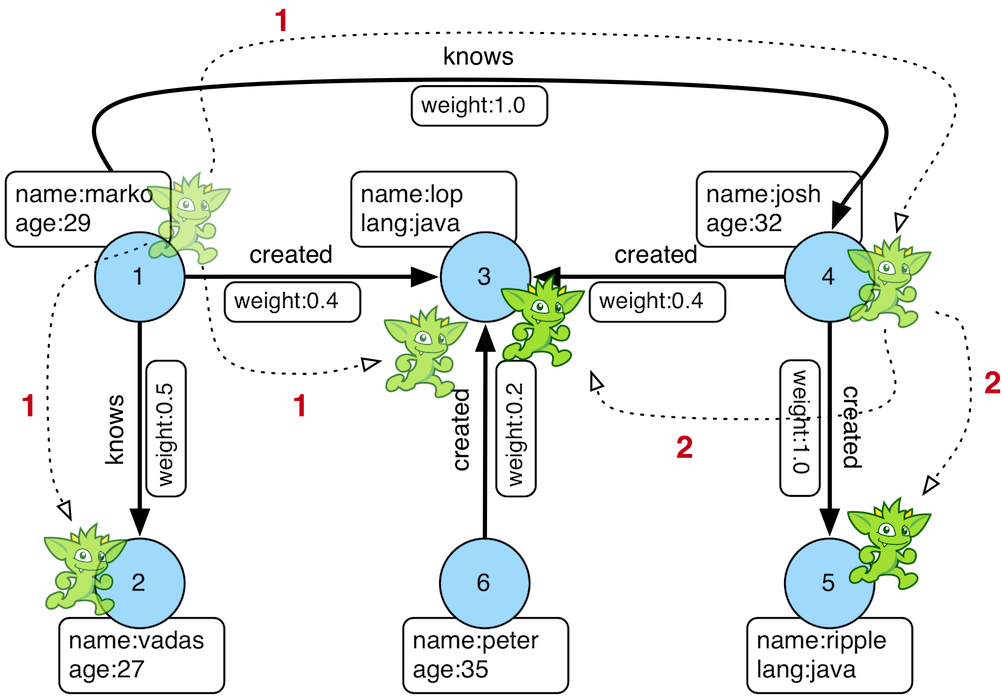``````gremlin> g.V(1).repeat(out()).times(2).emit().path().by('name')
==>[marko,lop]
==>[marko,josh]
==>[marko,josh,ripple]
==>[marko,josh,lop]``````
``g.V(1).repeat(out()).times(2).emit().path().by('name')``

The first time through the `repeat()`, the vertices lop, vadas, and josh are seen. Given that `loops==1`, the traverser repeats. However, because the emit-predicate is declared true, those vertices are emitted. The next time through `repeat()`, the vertices traversed are ripple and lop (Josh’s created projects, as lop and vadas have no out edges). Given that `loops==2`, the until-predicate fails and ripple and lop are emitted. Therefore, the traverser has seen the vertices: lop, vadas, josh, ripple, and lop.

Finally, note that both `emit()` and `until()` can take a traversal and in such, situations, the predicate is determined by `traversal.hasNext()`. A few examples are provided below.

``````gremlin> g.V(1).repeat(out()).until(hasLabel('software')).path().by('name') //1\
==>[marko,lop]
==>[marko,josh,ripple]
==>[marko,josh,lop]
gremlin> g.V(1).emit(hasLabel('person')).repeat(out()).path().by('name') //2\
==>[marko]
==>[marko,josh]
gremlin> g.V(1).repeat(out()).until(outE().count().is(0)).path().by('name') //3\
==>[marko,lop]
==>[marko,josh,ripple]
==>[marko,josh,lop]``````
``````g.V(1).repeat(out()).until(hasLabel('software')).path().by('name') //1\
g.V(1).emit(hasLabel('person')).repeat(out()).path().by('name') //2\
g.V(1).repeat(out()).until(outE().count().is(0)).path().by('name') //3``````
1. Starting from vertex 1, keep taking outgoing edges until a software vertex is reached.

2. Starting from vertex 1, and in an infinite loop, emit the vertex if it is a person and then traverser the outgoing edges.

3. Starting from vertex 1, keep taking outgoing edges until a vertex is reached that has no more outgoing edges.

 Warning The anonymous traversal of `emit()` and `until()` (not `repeat()`) process their current objects "locally." In OLAP, where the atomic unit of computing is the vertex and its local "star graph," it is important that the anonymous traversals do not leave the confines of the vertex’s star graph. In other words, they can not traverse to an adjacent vertex’s properties or edges.

### Sack StepA traverser can contain a local data structure called a "sack". The `sack()`-step is used to read and write sacks (sideEffect or map). Each sack of each traverser is created when using `GraphTraversal.withSack(initialValueSupplier,splitOperator?,mergeOperator?)`.

• Initial value supplier: A `Supplier` providing the initial value of each traverser’s sack.

• Split operator: a `UnaryOperator` that clones the traverser’s sack when the traverser splits. If no split operator is provided, then `UnaryOperator.identity()` is assumed.

• Merge operator: A `BinaryOperator` that unites two traverser’s sack when they are merged. If no merge operator is provided, then traversers with sacks can not be merged.

Two trivial examples are presented below to demonstrate the initial value supplier. In the first example below, a traverser is created at each vertex in the graph (`g.V()`), with a 1.0 sack (`withSack(1.0f)`), and then the sack value is accessed (`sack()`). In the second example, a random float supplier is used to generate sack values.

``````gremlin> g.withSack(1.0f).V().sack()
==>1.0
==>1.0
==>1.0
==>1.0
==>1.0
==>1.0
gremlin> rand = new Random()
==>java.util.Random@444456cb
gremlin> g.withSack {rand.nextFloat()}.V().sack()
==>0.02046609
==>0.0612424
==>0.45533913
==>0.41660023
==>0.81903714
==>0.10193539``````
``````g.withSack(1.0f).V().sack()
rand = new Random()
g.withSack {rand.nextFloat()}.V().sack()``````

A more complicated initial value supplier example is presented below where the sack values are used in a running computation and then emitted at the end of the traversal. When an edge is traversed, the edge weight is multiplied by the sack value (`sack(mult).by('weight')`). Note that the `by()`-modulator can be any arbitrary traversal.

``````gremlin> g.withSack(1.0f).V().repeat(outE().sack(mult).by('weight').inV()).times(2)
==>v
==>v
gremlin> g.withSack(1.0f).V().repeat(outE().sack(mult).by('weight').inV()).times(2).sack()
==>1.0
==>0.4
gremlin> g.withSack(1.0f).V().repeat(outE().sack(mult).by('weight').inV()).times(2).path().
by().by('weight')
==>[v,1.0,v,1.0,v]
==>[v,1.0,v,0.4,v]``````
``````g.withSack(1.0f).V().repeat(outE().sack(mult).by('weight').inV()).times(2)
g.withSack(1.0f).V().repeat(outE().sack(mult).by('weight').inV()).times(2).sack()
g.withSack(1.0f).V().repeat(outE().sack(mult).by('weight').inV()).times(2).path().
by().by('weight')``````When complex objects are used (i.e. non-primitives), then a split operator should be defined to ensure that each traverser gets a clone of its parent’s sack. The first example does not use a split operator and as such, the same map is propagated to all traversers (a global data structure). The second example, demonstrates how `Map.clone()` ensures that each traverser’s sack contains a unique, local sack.

``````gremlin> g.withSack {[:]}.V().out().out().
sack {m,v -> m[v.value('name')] = v.value('lang'); m}.sack() // BAD: single map
==>[ripple:java]
==>[ripple:java,lop:java]
gremlin> g.withSack {[:]}{it.clone()}.V().out().out().
sack {m,v -> m[v.value('name')] = v.value('lang'); m}.sack() // GOOD: cloned map
==>[ripple:java]
==>[lop:java]``````
``````g.withSack {[:]}.V().out().out().
sack {m,v -> m[v.value('name')] = v.value('lang'); m}.sack() // BAD: single map
g.withSack {[:]}{it.clone()}.V().out().out().
sack {m,v -> m[v.value('name')] = v.value('lang'); m}.sack() // GOOD: cloned map``````
 Note For primitives (i.e. integers, longs, floats, etc.), a split operator is not required as a primitives are encoded in the memory address of the sack, not as a reference to an object.

If a merge operator is not provided, then traversers with sacks can not be bulked. However, in many situations, merging the sacks of two traversers at the same location is algorithmically sound and good to provide so as to gain the bulking optimization. In the examples below, the binary merge operator is `Operator.sum`. Thus, when two traverser merge, their respective sacks are added together.

``````gremlin> g.withSack(1.0d).V(1).out('knows').in('knows') //1\
==>v
==>v
gremlin> g.withSack(1.0d).V(1).out('knows').in('knows').sack() //2\
==>1.0
==>1.0
gremlin> g.withSack(1.0d, sum).V(1).out('knows').in('knows').sack() //3\
==>2.0
==>2.0
gremlin> g.withSack(1.0d).V(1).local(outE('knows').barrier(normSack).inV()).in('knows').barrier() //4\
==>v
==>v
gremlin> g.withSack(1.0d).V(1).local(outE('knows').barrier(normSack).inV()).in('knows').barrier().sack() //5\
==>0.5
==>0.5
gremlin> g.withSack(1.0d,sum).V(1).local(outE('knows').barrier(normSack).inV()).in('knows').barrier().sack() //6\
==>1.0
==>1.0
gremlin> g.withBulk(false).withSack(1.0f,sum).V(1).local(outE('knows').barrier(normSack).inV()).in('knows').barrier().sack() //7\
==>1.0
gremlin> g.withBulk(false).withSack(1.0f).V(1).local(outE('knows').barrier(normSack).inV()).in('knows').barrier().sack() //8\
==>0.5
==>0.5
gremlin>``````
``````g.withSack(1.0d).V(1).out('knows').in('knows') //1\
g.withSack(1.0d).V(1).out('knows').in('knows').sack() //2\
g.withSack(1.0d, sum).V(1).out('knows').in('knows').sack() //3\
g.withSack(1.0d).V(1).local(outE('knows').barrier(normSack).inV()).in('knows').barrier() //4\
g.withSack(1.0d).V(1).local(outE('knows').barrier(normSack).inV()).in('knows').barrier().sack() //5\
g.withSack(1.0d,sum).V(1).local(outE('knows').barrier(normSack).inV()).in('knows').barrier().sack() //6\
g.withBulk(false).withSack(1.0f,sum).V(1).local(outE('knows').barrier(normSack).inV()).in('knows').barrier().sack() //7\
g.withBulk(false).withSack(1.0f).V(1).local(outE('knows').barrier(normSack).inV()).in('knows').barrier().sack() //8\``````
1. We find vertex 1 twice because he knows two other people

2. Without a merge operation the sack values are 1.0.

3. When specifying `sum` as the merge operation, the sack values are 2.0 because of bulking

4. Like 1, but using barrier internally

5. The `local(…​barrier(normSack)…​)` ensures that all traversers leaving vertex 1 have an evenly distributed amount of the initial 1.0 "energy" (50-50), i.e. the sack is 0.5 on each result

6. Like 3, but using `sum` as merge operator leads to the expected 1.0

7. There is now a single traverser with bulk of 2 and sack of 1.0 and thus, setting withBulk(false) yields the expected 1.0

8. Like 7, but without the sum operator

### Sample Step

The `sample()`-step is useful for sampling some number of traversers previous in the traversal.

``````gremlin> g.V().outE().sample(1).values('weight')
==>1.0
gremlin> g.V().outE().sample(1).by('weight').values('weight')
==>1.0
gremlin> g.V().outE().sample(2).by('weight').values('weight')
==>1.0
==>0.5``````
``````g.V().outE().sample(1).values('weight')
g.V().outE().sample(1).by('weight').values('weight')
g.V().outE().sample(2).by('weight').values('weight')``````

One of the more interesting use cases for `sample()` is when it is used in conjunction with `local()`. The combination of the two steps supports the execution of random walks. In the example below, the traversal starts are vertex 1 and selects one edge to traverse based on a probability distribution generated by the weights of the edges. The output is always a single path as by selecting a single edge, the traverser never splits and continues down a single path in the graph.

``````gremlin> g.V(1).repeat(local(
bothE().sample(1).by('weight').otherV()
)).times(5)
==>v
gremlin> g.V(1).repeat(local(
bothE().sample(1).by('weight').otherV()
)).times(5).path()
==>[v,e[1-knows->4],v,e[4-created->3],v,e[6-created->3],v,e[6-created->3],v,e[1-created->3],v]
gremlin> g.V(1).repeat(local(
bothE().sample(1).by('weight').otherV()
)).times(10).path()
==>[v,e[1-knows->2],v,e[1-knows->2],v,e[1-created->3],v,e[4-created->3],v,e[4-created->5],v,e[4-created->5],v,e[4-created->5],v,e[4-created->5],v,e[4-created->5],v,e[4-created->5],v]``````
``````g.V(1).repeat(local(
bothE().sample(1).by('weight').otherV()
)).times(5)
g.V(1).repeat(local(
bothE().sample(1).by('weight').otherV()
)).times(5).path()
g.V(1).repeat(local(
bothE().sample(1).by('weight').otherV()
)).times(10).path()``````

As a clarification, note that in the above example `local()` is not strictly required as it only does the random walk over a single vertex, but note what happens without it if multiple vertices are traversed:

``````gremlin> g.V().repeat(bothE().sample(1).by('weight').otherV()).times(5).path()
==>[v,e[1-knows->2],v,e[1-knows->4],v,e[4-created->5],v,e[4-created->5],v,e[1-knows->4],v]``````
``g.V().repeat(bothE().sample(1).by('weight').otherV()).times(5).path()``

The use of `local()` ensures that the traversal over `bothE()` occurs once per vertex traverser that passes through, thus allowing one random walk per vertex.

``````gremlin> g.V().repeat(local(bothE().sample(1).by('weight').otherV())).times(5).path()
==>[v,e[1-knows->4],v,e[4-created->3],v,e[1-created->3],v,e[1-knows->4],v,e[4-created->3],v]
==>[v,e[1-knows->2],v,e[1-knows->2],v,e[1-knows->2],v,e[1-knows->2],v,e[1-knows->2],v]
==>[v,e[4-created->3],v,e[4-created->3],v,e[1-created->3],v,e[1-knows->4],v,e[4-created->5],v]
==>[v,e[4-created->3],v,e[4-created->3],v,e[4-created->3],v,e[1-created->3],v,e[1-knows->2],v]
==>[v,e[4-created->5],v,e[4-created->3],v,e[1-created->3],v,e[1-created->3],v,e[4-created->3],v]
==>[v,e[6-created->3],v,e[1-created->3],v,e[1-knows->2],v,e[1-knows->2],v,e[1-created->3],v]``````
``g.V().repeat(local(bothE().sample(1).by('weight').otherV())).times(5).path()``

So, while not strictly required, it is likely better to be explicit with the use of `local()` so that the proper intent of the traversal is expressed.

### Select Step

Functional languages make use of function composition and lazy evaluation to create complex computations from primitive operations. This is exactly what `Traversal` does. One of the differentiating aspects of Gremlin’s data flow approach to graph processing is that the flow need not always go "forward," but in fact, can go back to a previously seen area of computation. Examples include `path()` as well as the `select()`-step (map). There are two general ways to use `select()`-step.

1. Select labeled steps within a path (as defined by `as()` in a traversal).

2. Select objects out of a `Map<String,Object>` flow (i.e. a sub-map).

The first use case is demonstrated via example below.

``````gremlin> g.V().as('a').out().as('b').out().as('c') // no select
==>v
==>v
gremlin> g.V().as('a').out().as('b').out().as('c').select('a','b','c')
==>[a:v,b:v,c:v]
==>[a:v,b:v,c:v]
gremlin> g.V().as('a').out().as('b').out().as('c').select('a','b')
==>[a:v,b:v]
==>[a:v,b:v]
gremlin> g.V().as('a').out().as('b').out().as('c').select('a','b').by('name')
==>[a:marko,b:josh]
==>[a:marko,b:josh]
gremlin> g.V().as('a').out().as('b').out().as('c').select('a') //1\
==>v
==>v``````
``````g.V().as('a').out().as('b').out().as('c') // no select
g.V().as('a').out().as('b').out().as('c').select('a','b','c')
g.V().as('a').out().as('b').out().as('c').select('a','b')
g.V().as('a').out().as('b').out().as('c').select('a','b').by('name')
g.V().as('a').out().as('b').out().as('c').select('a') //1``````
1. If the selection is one step, no map is returned.

When there is only one label selected, then a single object is returned. This is useful for stepping back in a computation and easily moving forward again on the object reverted to.

``````gremlin> g.V().out().out()
==>v
==>v
gremlin> g.V().out().out().path()
==>[v,v,v]
==>[v,v,v]
gremlin> g.V().as('x').out().out().select('x')
==>v
==>v
gremlin> g.V().out().as('x').out().select('x')
==>v
==>v
gremlin> g.V().out().out().as('x').select('x') // pointless
==>v
==>v``````
``````g.V().out().out()
g.V().out().out().path()
g.V().as('x').out().out().select('x')
g.V().out().as('x').out().select('x')
g.V().out().out().as('x').select('x') // pointless``````
 Note When executing a traversal with `select()` on a standard traversal engine (i.e. OLTP), `select()` will do its best to avoid calculating the path history and instead, will rely on a global data structure for storing the currently selected object. As such, if only a subset of the path walked is required, `select()` should be used over the more resource intensive `path()`-step.

When the set of keys or values (i.e. columns) of a path or map are needed, use `select(keys)` and `select(values)`, respectively. This is especially useful when one is only interested in the top N elements in a `groupCount()` ranking.

``````gremlin> graph.io(graphml()).readGraph('data/grateful-dead.xml')
gremlin> g = graph.traversal()
==>graphtraversalsource[tinkergraph[vertices:808 edges:8049], standard]
gremlin> g.V().hasLabel('song').out('followedBy').groupCount().by('name').
order(local).by(values,desc).limit(local, 5)
==>[PLAYING IN THE BAND:107,JACK STRAW:99,TRUCKING:94,DRUMS:92,ME AND MY UNCLE:86]
gremlin> g.V().hasLabel('song').out('followedBy').groupCount().by('name').
order(local).by(values,desc).limit(local, 5).select(keys)
==>[PLAYING IN THE BAND,JACK STRAW,TRUCKING,DRUMS,ME AND MY UNCLE]
gremlin> g.V().hasLabel('song').out('followedBy').groupCount().by('name').
order(local).by(values,desc).limit(local, 5).select(keys).unfold()
==>PLAYING IN THE BAND
==>JACK STRAW
==>TRUCKING
==>DRUMS
==>ME AND MY UNCLE``````
``````graph.io(graphml()).readGraph('data/grateful-dead.xml')
g = graph.traversal()
g.V().hasLabel('song').out('followedBy').groupCount().by('name').
order(local).by(values,desc).limit(local, 5)
g.V().hasLabel('song').out('followedBy').groupCount().by('name').
order(local).by(values,desc).limit(local, 5).select(keys)
g.V().hasLabel('song').out('followedBy').groupCount().by('name').
order(local).by(values,desc).limit(local, 5).select(keys).unfold()``````

Similarly, for extracting the values from a path or map.

``````gremlin> graph.io(graphml()).readGraph('data/grateful-dead.xml')
gremlin> g = graph.traversal()
==>graphtraversalsource[tinkergraph[vertices:808 edges:8049], standard]
gremlin> g.V().hasLabel('song').out('sungBy').groupCount().by('name') //1\
gremlin> g.V().hasLabel('song').out('sungBy').groupCount().by('name').select(values) //2\
==>[9,1,19,1,1,36,6,1,10,2,10,1,4,1,1,3,4,2,3,1,1,1,146,2,1,99,1,2,4,2,1,3,1,1,1,1,1,2,17,2,3,3,4,7,22,2,3,1,2,18,2,1,1,3,1,5,2,8,2,6]
gremlin> g.V().hasLabel('song').out('sungBy').groupCount().by('name').select(values).unfold().
groupCount().order(local).by(values,desc).limit(local, 5) //3\
==>[1:22,2:12,3:7,4:4,6:2]``````
``````graph.io(graphml()).readGraph('data/grateful-dead.xml')
g = graph.traversal()
g.V().hasLabel('song').out('sungBy').groupCount().by('name') //1\
g.V().hasLabel('song').out('sungBy').groupCount().by('name').select(values) //2\
g.V().hasLabel('song').out('sungBy').groupCount().by('name').select(values).unfold().
groupCount().order(local).by(values,desc).limit(local, 5) //3``````
1. Which artist sung how many songs?

2. Get an anonymized set of song repertoire sizes.

3. What are the 5 most common song repertoire sizes?

 Warning Note that `by()`-modulation is not supported with `select(keys)` and `select(values)`.

There is also an option to supply a `Pop` operation to `select()` to manipulate `List` objects in the `Traverser`:

``````gremlin> g.V(1).as("a").repeat(out().as("a")).times(2).select(first, "a")
==>v
==>v
gremlin> g.V(1).as("a").repeat(out().as("a")).times(2).select(last, "a")
==>v
==>v
gremlin> g.V(1).as("a").repeat(out().as("a")).times(2).select(all, "a")
==>[v,v,v]
==>[v,v,v]``````
``````g.V(1).as("a").repeat(out().as("a")).times(2).select(first, "a")
g.V(1).as("a").repeat(out().as("a")).times(2).select(last, "a")
g.V(1).as("a").repeat(out().as("a")).times(2).select(all, "a")``````

In addition to the previously shown examples, where `select()` was used to select an element based on a static key, `select()` can also accept a traversal that emits a key.

 Warning Since the key used by `select()` cannot be determined at compile time, the `TraversalSelectStep` enables full path tracking.
``````gremlin> g.withSideEffect("alias", ["marko":"okram"]).V(). //1\
values("name").sack(assign). //2\
optional(select("alias").select(sack())) //3\
==>okram
==>lop
==>josh
==>ripple
==>peter``````
``````g.withSideEffect("alias", ["marko":"okram"]).V(). //1\
values("name").sack(assign). //2\
optional(select("alias").select(sack()))         //3``````
1. Inject a name alias map and start the traversal from all vertices.

2. Select all `name` values and store them as the current traverser’s sack value.

3. Optionally select the alias for the current name from the injected map.

#### Using Where with Select

Like `match()`-step, it is possible to use `where()`, as where is a filter that processes `Map<String,Object>` streams.

``````gremlin> g.V().as('a').out('created').in('created').as('b').select('a','b').by('name') //1\
==>[a:marko,b:marko]
==>[a:marko,b:josh]
==>[a:marko,b:peter]
==>[a:josh,b:josh]
==>[a:josh,b:marko]
==>[a:josh,b:josh]
==>[a:josh,b:peter]
==>[a:peter,b:marko]
==>[a:peter,b:josh]
==>[a:peter,b:peter]
gremlin> g.V().as('a').out('created').in('created').as('b').
select('a','b').by('name').where('a',neq('b')) //2\
==>[a:marko,b:josh]
==>[a:marko,b:peter]
==>[a:josh,b:marko]
==>[a:josh,b:peter]
==>[a:peter,b:marko]
==>[a:peter,b:josh]
gremlin> g.V().as('a').out('created').in('created').as('b').
select('a','b'). //3\
where('a',neq('b')).
where(__.as('a').out('knows').as('b')).
select('a','b').by('name')
==>[a:marko,b:josh]``````
``````g.V().as('a').out('created').in('created').as('b').select('a','b').by('name') //1\
g.V().as('a').out('created').in('created').as('b').
select('a','b').by('name').where('a',neq('b')) //2\
g.V().as('a').out('created').in('created').as('b').
select('a','b'). //3\
where('a',neq('b')).
where(__.as('a').out('knows').as('b')).
select('a','b').by('name')``````
1. A standard `select()` that generates a `Map<String,Object>` of variables bindings in the path (i.e. `a` and `b`) for the sake of a running example.

2. The `select().by('name')` projects each binding vertex to their name property value and `where()` operates to ensure respective `a` and `b` strings are not the same.

3. The first `select()` projects a vertex binding set. A binding is filtered if `a` vertex equals `b` vertex. A binding is filtered if `a` doesn’t know `b`. The second and final `select()` projects the name of the vertices.

### SimplePath Step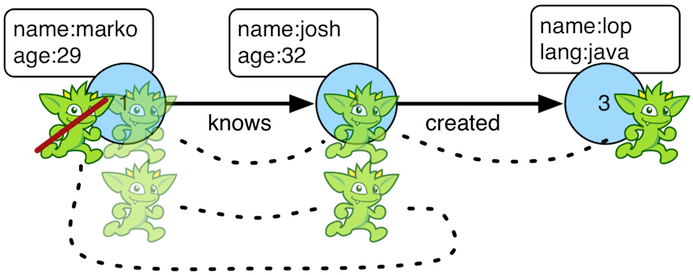When it is important that a traverser not repeat its path through the graph, `simplePath()`-step should be used (filter). The path information of the traverser is analyzed and if the path has repeated objects in it, the traverser is filtered. If cyclic behavior is desired, see `cyclicPath()`.

``````gremlin> g.V(1).both().both()
==>v
==>v
==>v
==>v
==>v
==>v
==>v
gremlin> g.V(1).both().both().simplePath()
==>v
==>v
==>v
==>v
gremlin> g.V(1).both().both().simplePath().path()
==>[v,v,v]
==>[v,v,v]
==>[v,v,v]
==>[v,v,v]
gremlin> g.V().out().as('a').out().as('b').out().as('c').
simplePath().by(label).
path()
gremlin> g.V().out().as('a').out().as('b').out().as('c').
simplePath().
by(label).
from('b').
to('c').
path().
by('name')``````
``````g.V(1).both().both()
g.V(1).both().both().simplePath()
g.V(1).both().both().simplePath().path()
g.V().out().as('a').out().as('b').out().as('c').
simplePath().by(label).
path()
g.V().out().as('a').out().as('b').out().as('c').
simplePath().
by(label).
from('b').
to('c').
path().
by('name')``````

By using the `from()` and `to()` modulators traversers can ensure that only certain sections of the path are acyclic.

``````gremlin> g.addV().property(id, 'A').as('a').
gremlin> g.V('A').repeat(both().simplePath()).times(3).path() //1\
==>[v[A],v[B],v[C],v[D]]
gremlin> g.V('D').repeat(both().simplePath()).times(3).path() //2\
==>[v[D],v[C],v[B],v[A]]
gremlin> g.V('A').as('a').
repeat(both().simplePath().from('a')).times(3).as('b').
repeat(both().simplePath().from('b')).times(3).path() //3\
==>[v[A],v[B],v[C],v[D],v[C],v[B],v[A]]``````
``````g.addV().property(id, 'A').as('a').
g.V('A').repeat(both().simplePath()).times(3).path() //1\
g.V('D').repeat(both().simplePath()).times(3).path() //2\
g.V('A').as('a').
repeat(both().simplePath().from('a')).times(3).as('b').
repeat(both().simplePath().from('b')).times(3).path()  //3``````
1. Traverse all acyclic 3-hop paths starting from vertex `A`

2. Traverse all acyclic 3-hop paths starting from vertex `D`

3. Traverse all acyclic 3-hop paths starting from vertex `A` and from there again all 3-hop paths. The second path may cross the vertices from the first path.

### Skip Step

The `skip()`-step is analogous to `range()`-step save that the higher end range is set to -1.

``````gremlin> g.V().values('age').order()
==>27
==>29
==>32
==>35
gremlin> g.V().values('age').order().skip(2)
==>32
==>35
gremlin> g.V().values('age').order().range(2, -1)
==>32
==>35``````
``````g.V().values('age').order()
g.V().values('age').order().skip(2)
g.V().values('age').order().range(2, -1)``````

The `skip()`-step can also be applied with `Scope.local`, in which case it operates on the incoming collection.

``````gremlin> g.V().hasLabel('person').filter(outE('created')).as('p'). //1\
map(out('created').values('name').fold()).
project('person','primary','other').
by(select('p').by('name')).
by(limit(local, 1)). //2\
by(skip(local, 1)) //3\
==>[person:marko,primary:lop,other:[]]
==>[person:josh,primary:ripple,other:[lop]]
==>[person:peter,primary:lop,other:[]]``````
``````g.V().hasLabel('person').filter(outE('created')).as('p'). //1\
map(out('created').values('name').fold()).
project('person','primary','other').
by(select('p').by('name')).
by(limit(local, 1)). //2\
by(skip(local, 1)) //3``````
1. For each person who created something…​

2. …​select the first project (random order) as `primary` and…​

3. …​select all other projects as `other`.

### Store Step

When lazy aggregation is needed, `store()`-step (sideEffect) should be used over `aggregate()`. The two steps differ in that `store()` does not block and only stores objects in its side-effect collection as they pass through.

``````gremlin> g.V().aggregate('x').limit(1).cap('x')
==>[v,v,v,v,v,v]
gremlin> g.V().store('x').limit(1).cap('x')
==>[v]``````
``````g.V().aggregate('x').limit(1).cap('x')
g.V().store('x').limit(1).cap('x')``````

It is interesting to note that there are two results in the `store()` side-effect even though the interval selection is for 1 object. Realize that when the second object is on its way to the `range()` filter (i.e. `[0..1]`), it passes through `store()` and thus, stored before filtered.

``````gremlin> g.E().store('x').by('weight').cap('x')
==>[0.5,1.0,1.0,0.4,0.4,0.2]``````
``g.E().store('x').by('weight').cap('x')``

### Subgraph Step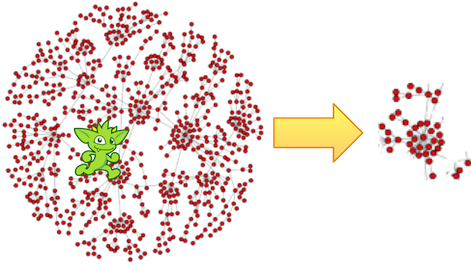Extracting a portion of a graph from a larger one for analysis, visualization or other purposes is a fairly common use case for graph analysts and developers. The `subgraph()`-step (sideEffect) provides a way to produce an edge-induced subgraph from virtually any traversal. The following example demonstrates how to produce the "knows" subgraph:

``````gremlin> subGraph = g.E().hasLabel('knows').subgraph('subGraph').cap('subGraph').next() //1\
==>tinkergraph[vertices:3 edges:2]
gremlin> sg = subGraph.traversal()
==>graphtraversalsource[tinkergraph[vertices:3 edges:2], standard]
gremlin> sg.E() //2\
==>e[1-knows->2]
==>e[1-knows->4]``````
``````subGraph = g.E().hasLabel('knows').subgraph('subGraph').cap('subGraph').next() //1\
sg = subGraph.traversal()
sg.E() //2``````
1. As this function produces "edge-induced" subgraphs, `subgraph()` must be called at edge steps.

2. The subgraph contains only "knows" edges.

A more common subgraphing use case is to get all of the graph structure surrounding a single vertex:

``````gremlin> subGraph = g.V(3).repeat(__.inE().subgraph('subGraph').outV()).times(3).cap('subGraph').next() //1\
==>tinkergraph[vertices:4 edges:4]
gremlin> sg = subGraph.traversal()
==>graphtraversalsource[tinkergraph[vertices:4 edges:4], standard]
gremlin> sg.E()
==>e[1-knows->4]
==>e[1-created->3]
==>e[4-created->3]
==>e[6-created->3]``````
``````subGraph = g.V(3).repeat(__.inE().subgraph('subGraph').outV()).times(3).cap('subGraph').next() //1\
sg = subGraph.traversal()
sg.E()``````
1. Starting at vertex `3`, traverse 3 steps away on in-edges, outputting all of that into the subgraph.

There can be multiple `subgraph()` calls within the same traversal. Each operating against either the same graph (i.e. same side-effect key) or different graphs (i.e. different side-effect keys).

``````gremlin> t = g.V().outE('knows').subgraph('knowsG').inV().outE('created').subgraph('createdG').
inV().inE('created').subgraph('createdG').iterate()
gremlin> t.sideEffects.get('knowsG').traversal().E()
==>e[1-knows->2]
==>e[1-knows->4]
gremlin> t.sideEffects.get('createdG').traversal().E()
==>e[1-created->3]
==>e[4-created->5]
==>e[4-created->3]
==>e[6-created->3]``````
``````t = g.V().outE('knows').subgraph('knowsG').inV().outE('created').subgraph('createdG').
inV().inE('created').subgraph('createdG').iterate()
t.sideEffects.get('knowsG').traversal().E()
t.sideEffects.get('createdG').traversal().E()``````

TinkerGraph is the ideal (and default) `Graph` into which a subgraph is extracted as it’s fast, in-memory, and supports user-supplied identifiers which can be any Java object. It is this last feature that needs some focus as many TinkerPop-enabled graphs have complex identifier types and TinkerGraph’s ability to consume those makes it a perfect host for an incoming subgraph. However care needs to be taken when using the elements of the TinkerGraph subgraph. The original graph’s identifiers may be preserved, but the elements of the graph are now TinkerGraph objects like, `TinkerVertex` and `TinkerEdge`. As a result, they can not be used directly in Gremlin running against the original graph. For example, the following traversal would likely return an error:

``````Vertex v = sg.V().has('name','marko').next();  //1
List<Vertex> vertices = g.V(v).out().toList(); //2``````
1. Here "sg" is a reference to a TinkerGraph subgraph and "v" is a `TinkerVertex`.

2. The `g.V(v)` has the potential to fail as "g" is the original `Graph` instance and not a TinkerGraph - it could reject the `TinkerVertex` instance as it will not recognize it.

It is safer to wrap the `TinkerVertex` in a `ReferenceVertex` or simply reference the `id()` as follows:

``````Vertex v = sg.V().has('name','marko').next();
List<Vertex> vertices = g.V(v.id()).out().toList();

// OR

Vertex v = new ReferenceVertex(sg.V().has('name','marko').next());
List<Vertex> vertices = g.V(v).out().toList();``````

### Sum Step

The `sum()`-step (map) operates on a stream of numbers and sums the numbers together to yield a double. Note that the current traverser number is multiplied by the traverser bulk to determine how many such numbers are being represented.

``````gremlin> g.V().values('age').sum()
==>123
gremlin> g.V().repeat(both()).times(3).values('age').sum()
==>1471``````
``````g.V().values('age').sum()
g.V().repeat(both()).times(3).values('age').sum()``````
 Important `sum(local)` determines the sum of the current, local object (not the objects in the traversal stream). This works for `Collection`-type objects. For any other object, a sum of `Double.NaN` is returned.

### Tail StepThe `tail()`-step is analogous to `limit()`-step, except that it emits the last `n`-objects instead of the first `n`-objects.

``````gremlin> g.V().values('name').order()
==>josh
==>lop
==>marko
==>peter
==>ripple
gremlin> g.V().values('name').order().tail() //1\
gremlin> g.V().values('name').order().tail(1) //2\
gremlin> g.V().values('name').order().tail(3) //3\
==>peter
==>ripple
``````g.V().values('name').order()
g.V().values('name').order().tail() //1\
g.V().values('name').order().tail(1) //2\
g.V().values('name').order().tail(3) //3``````
1. Last name (alphabetically).

2. Same as statement 1.

3. Last three names.

The `tail()`-step can also be applied with `Scope.local`, in which case it operates on the incoming collection.

``````gremlin> g.V().as('a').out().as('a').out().as('a').select('a').by(tail(local)).values('name') //1\
==>ripple
==>lop
gremlin> g.V().as('a').out().as('a').out().as('a').select('a').by(unfold().values('name').fold()).tail(local) //2\
==>ripple
==>lop
gremlin> g.V().as('a').out().as('a').out().as('a').select('a').by(unfold().values('name').fold()).tail(local, 2) //3\
==>[ripple]
==>[lop]
gremlin> g.V().valueMap().tail(local) //4\
==>[age:]
==>[age:]
==>[lang:[java]]
==>[age:]
==>[lang:[java]]
==>[age:]``````
``````g.V().as('a').out().as('a').out().as('a').select('a').by(tail(local)).values('name') //1\
g.V().as('a').out().as('a').out().as('a').select('a').by(unfold().values('name').fold()).tail(local) //2\
g.V().as('a').out().as('a').out().as('a').select('a').by(unfold().values('name').fold()).tail(local, 2) //3\
g.V().valueMap().tail(local) //4``````
1. Only the most recent name from the "a" step (`List<Vertex>` becomes `Vertex`).

2. Same result as statement 1 (`List<String>` becomes `String`).

3. `List<String>` for each path containing the last two names from the 'a' step.

4. `Map<String, Object>` for each vertex, but containing only the last property value.

### TimeLimit Step

In many situations, a graph traversal is not about getting an exact answer as its about getting a relative ranking. A classic example is recommendation. What is desired is a relative ranking of vertices, not their absolute rank. Next, it may be desirable to have the traversal execute for no more than 2 milliseconds. In such situations, `timeLimit()`-step (filter) can be used.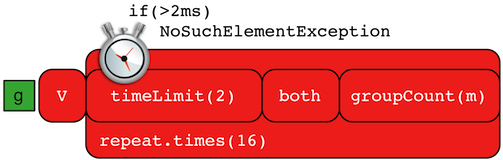Note The method `clock(int runs, Closure code)` is a utility preloaded in the Gremlin Console that can be used to time execution of a body of code.
``````gremlin> g.V().repeat(both().groupCount('m')).times(16).cap('m').order(local).by(values,desc).next()
==>v=2744208
==>v=2744208
==>v=2744208
==>v=1136688
==>v=1136688
==>v=1136688
gremlin> clock(1) {g.V().repeat(both().groupCount('m')).times(16).cap('m').order(local).by(values,desc).next()}
==>2.692198
gremlin> g.V().repeat(timeLimit(2).both().groupCount('m')).times(16).cap('m').order(local).by(values,desc).next()
==>v=2744208
==>v=2744208
==>v=2744208
==>v=1136688
==>v=1136688
==>v=1136688
gremlin> clock(1) {g.V().repeat(timeLimit(2).both().groupCount('m')).times(16).cap('m').order(local).by(values,desc).next()}
==>2.2087499999999998``````
``````g.V().repeat(both().groupCount('m')).times(16).cap('m').order(local).by(values,desc).next()
clock(1) {g.V().repeat(both().groupCount('m')).times(16).cap('m').order(local).by(values,desc).next()}
g.V().repeat(timeLimit(2).both().groupCount('m')).times(16).cap('m').order(local).by(values,desc).next()
clock(1) {g.V().repeat(timeLimit(2).both().groupCount('m')).times(16).cap('m').order(local).by(values,desc).next()}``````

In essence, the relative order is respected, even through the number of traversers at each vertex is not. The primary benefit being that the calculation is guaranteed to complete at the specified time limit (in milliseconds). Finally, note that the internal clock of `timeLimit()`-step starts when the first traverser enters it. When the time limit is reached, any `next()` evaluation of the step will yield a `NoSuchElementException` and any `hasNext()` evaluation will yield `false`.

### To Step

The `to()`-step is not an actual step, but instead is a "step-modulator" similar to `as()` and `by()`. If a step is able to accept traversals or strings then `to()` is the means by which they are added. The general pattern is `step().to()`. See `from()`-step.

The list of steps that support `to()`-modulation are: `simplePath()`, `cyclicPath()`, `path()`, and `addE()`.

### Tree Step

From any one element (i.e. vertex or edge), the emanating paths from that element can be aggregated to form a tree. Gremlin provides `tree()`-step (sideEffect) for such this situation.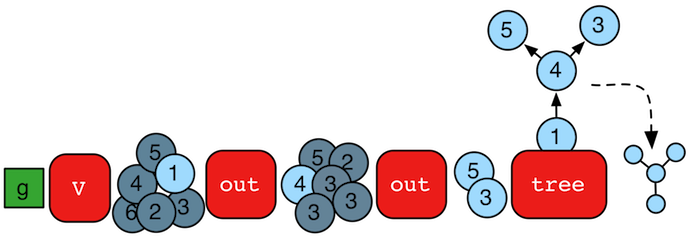``````gremlin> tree = g.V().out().out().tree().next()
==>v={v={v={}, v={}}}``````
``tree = g.V().out().out().tree().next()``

It is important to see how the paths of all the emanating traversers are united to form the tree.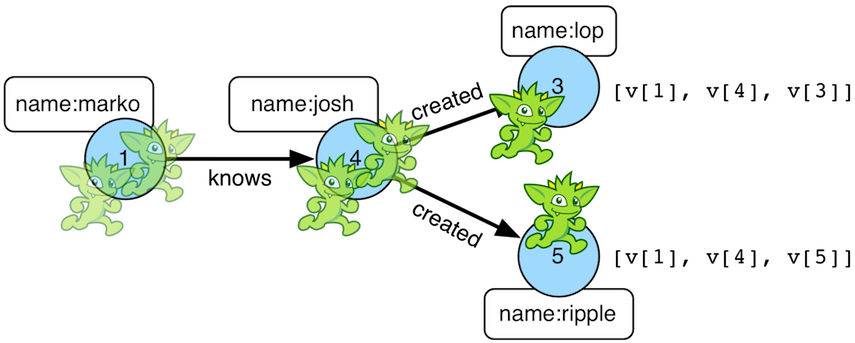The resultant tree data structure can then be manipulated (see `Tree` JavaDoc).

``````gremlin> tree = g.V().out().out().tree().by('name').next()
==>marko={josh={ripple={}, lop={}}}
gremlin> tree['marko']
==>josh={ripple={}, lop={}}
gremlin> tree['marko']['josh']
==>ripple={}
==>lop={}
gremlin> tree.getObjectsAtDepth(3)
==>ripple
==>lop``````
``````tree = g.V().out().out().tree().by('name').next()
tree['marko']
tree['marko']['josh']
tree.getObjectsAtDepth(3)``````

Note that when using `by()`-modulation, tree nodes are combined based on projection uniqueness, not on the uniqueness of the original objects being projected. For instance:

``````gremlin> g.V().has('name','josh').out('created').values('name').tree() //1\
==>[v:[v:[lop:[]],v:[ripple:[]]]]
gremlin> g.V().has('name','josh').out('created').values('name').
tree().by('name').by(label).by() //2\
==>[josh:[software:[ripple:[],lop:[]]]]``````
``````g.V().has('name','josh').out('created').values('name').tree() //1\
g.V().has('name','josh').out('created').values('name').
tree().by('name').by(label).by() //2``````
1. When the `tree()` is created, vertex 3 and 5 are unique and thus, form unique branches in the tree structure.

2. When the `tree()` is `by()`-modulated by `label`, then vertex 3 and 5 are both "software" and thus are merged to a single node in the tree.

### Unfold Step

If the object reaching `unfold()` (flatMap) is an iterator, iterable, or map, then it is unrolled into a linear form. If not, then the object is simply emitted. Please see `fold()` step for the inverse behavior.

``````gremlin> g.V(1).out().fold().inject('gremlin',[1.23,2.34])
==>gremlin
==>[1.23,2.34]
==>[v,v,v]
gremlin> g.V(1).out().fold().inject('gremlin',[1.23,2.34]).unfold()
==>gremlin
==>1.23
==>2.34
==>v
==>v
==>v``````
``````g.V(1).out().fold().inject('gremlin',[1.23,2.34])
g.V(1).out().fold().inject('gremlin',[1.23,2.34]).unfold()``````

Note that `unfold()` does not recursively unroll iterators. Instead, `repeat()` can be used to for recursive unrolling.

``````gremlin> inject(1,[2,3,[4,5,]])
==>1
==>[2,3,[4,5,]]
gremlin> inject(1,[2,3,[4,5,]]).unfold()
==>1
==>2
==>3
==>[4,5,]
gremlin> inject(1,[2,3,[4,5,]]).repeat(unfold()).until(count(local).is(1)).unfold()
==>1
==>2
==>3
==>4
==>5
==>6``````
``````inject(1,[2,3,[4,5,]])
inject(1,[2,3,[4,5,]]).unfold()
inject(1,[2,3,[4,5,]]).repeat(unfold()).until(count(local).is(1)).unfold()``````

### Union Step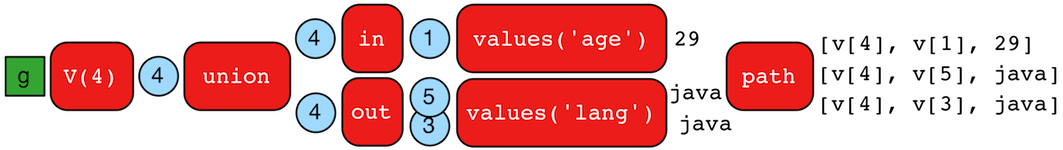The `union()`-step (branch) supports the merging of the results of an arbitrary number of traversals. When a traverser reaches a `union()`-step, it is copied to each of its internal steps. The traversers emitted from `union()` are the outputs of the respective internal traversals.

``````gremlin> g.V(4).union(
__.in().values('age'),
out().values('lang'))
==>29
==>java
==>java
gremlin> g.V(4).union(
__.in().values('age'),
out().values('lang')).path()
==>[v,v,29]
==>[v,v,java]
==>[v,v,java]``````
``````g.V(4).union(
__.in().values('age'),
out().values('lang'))
g.V(4).union(
__.in().values('age'),
out().values('lang')).path()``````

### Until Step

The `until`-step is not an actual step, but is instead a step modulator for `repeat()` (find more documentation on the `until()` there).

### Value Step

The `value()`-step (map) takes a `Property` and extracts the value from it.

``````gremlin> g.V(1).properties().value()
==>marko
==>san diego
==>santa cruz
==>brussels
==>santa fe
gremlin> g.V(1).properties().properties().value()
==>1997
==>2001
==>2001
==>2004
==>2004
==>2005
==>2005``````
``````g.V(1).properties().value()
g.V(1).properties().properties().value()``````

### ValueMap Step

The `valueMap()`-step yields a Map representation of the properties of an element.

``````gremlin> g.V().valueMap()
==>[name:[marko],age:]
==>[name:[lop],lang:[java]]
==>[name:[josh],age:]
==>[name:[ripple],lang:[java]]
==>[name:[peter],age:]
gremlin> g.V().valueMap('age')
==>[age:]
==>[age:]
==>[]
==>[age:]
==>[]
==>[age:]
gremlin> g.V().valueMap('age','blah')
==>[age:]
==>[age:]
==>[]
==>[age:]
==>[]
==>[age:]
gremlin> g.E().valueMap()
==>[weight:0.5]
==>[weight:1.0]
==>[weight:0.4]
==>[weight:1.0]
==>[weight:0.4]
==>[weight:0.2]``````
``````g.V().valueMap()
g.V().valueMap('age')
g.V().valueMap('age','blah')
g.E().valueMap()``````

It is important to note that the map of a vertex maintains a list of values for each key. The map of an edge or vertex-property represents a single property (not a list). The reason is that vertices in TinkerPop3 leverage vertex properties which are support multiple values per key. Using the The Crew toy graph, the point is made explicit.

``````gremlin> g.V().valueMap()
==>[name:[marko],location:[san diego,santa cruz,brussels,santa fe]]
==>[name:[stephen],location:[centreville,dulles,purcellville]]
==>[name:[matthias],location:[bremen,baltimore,oakland,seattle]]
==>[name:[daniel],location:[spremberg,kaiserslautern,aachen]]
==>[name:[gremlin]]
==>[name:[tinkergraph]]
gremlin> g.V().has('name','marko').properties('location')
==>vp[location->san diego]
==>vp[location->santa cruz]
==>vp[location->brussels]
==>vp[location->santa fe]
gremlin> g.V().has('name','marko').properties('location').valueMap()
==>[startTime:1997,endTime:2001]
==>[startTime:2001,endTime:2004]
==>[startTime:2004,endTime:2005]
==>[startTime:2005]``````
``````g.V().valueMap()
g.V().has('name','marko').properties('location')
g.V().has('name','marko').properties('location').valueMap()``````

If the `id`, `label`, `key`, and `value` of the `Element` is desired, then a boolean triggers its insertion into the returned map.

``````gremlin> g.V().hasLabel('person').valueMap(true)
==>[id:1,label:person,name:[marko],location:[san diego,santa cruz,brussels,santa fe]]
==>[id:7,label:person,name:[stephen],location:[centreville,dulles,purcellville]]
==>[id:8,label:person,name:[matthias],location:[bremen,baltimore,oakland,seattle]]
==>[id:9,label:person,name:[daniel],location:[spremberg,kaiserslautern,aachen]]
gremlin> g.V().hasLabel('person').valueMap(true,'name')
==>[id:1,label:person,name:[marko]]
==>[id:7,label:person,name:[stephen]]
==>[id:8,label:person,name:[matthias]]
==>[id:9,label:person,name:[daniel]]
gremlin> g.V().hasLabel('person').properties('location').valueMap(true)
==>[id:6,value:san diego,key:location,startTime:1997,endTime:2001]
==>[id:7,value:santa cruz,key:location,startTime:2001,endTime:2004]
==>[id:8,value:brussels,key:location,startTime:2004,endTime:2005]
==>[id:9,value:santa fe,key:location,startTime:2005]
==>[id:10,value:centreville,key:location,startTime:1990,endTime:2000]
==>[id:11,value:dulles,key:location,startTime:2000,endTime:2006]
==>[id:12,value:purcellville,key:location,startTime:2006]
==>[id:13,value:bremen,key:location,startTime:2004,endTime:2007]
==>[id:14,value:baltimore,key:location,startTime:2007,endTime:2011]
==>[id:15,value:oakland,key:location,startTime:2011,endTime:2014]
==>[id:16,value:seattle,key:location,startTime:2014]
==>[id:17,value:spremberg,key:location,startTime:1982,endTime:2005]
==>[id:18,value:kaiserslautern,key:location,startTime:2005,endTime:2009]
==>[id:19,value:aachen,key:location,startTime:2009]``````
``````g.V().hasLabel('person').valueMap(true)
g.V().hasLabel('person').valueMap(true,'name')
g.V().hasLabel('person').properties('location').valueMap(true)``````

### Values Step

The `values()`-step (map) extracts the values of properties from an `Element` in the traversal stream.

``````gremlin> g.V(1).values()
==>marko
==>san diego
==>santa cruz
==>brussels
==>santa fe
gremlin> g.V(1).values('location')
==>san diego
==>santa cruz
==>brussels
==>santa fe
gremlin> g.V(1).properties('location').values()
==>1997
==>2001
==>2001
==>2004
==>2004
==>2005
==>2005``````
``````g.V(1).values()
g.V(1).values('location')
g.V(1).properties('location').values()``````

### Vertex Steps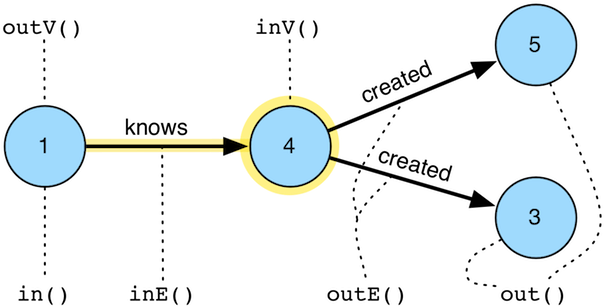The vertex steps (flatMap) are fundamental to the Gremlin language. Via these steps, its possible to "move" on the graph — i.e. traverse.

• `out(string…​)`: Move to the outgoing adjacent vertices given the edge labels.

• `in(string…​)`: Move to the incoming adjacent vertices given the edge labels.

• `both(string…​)`: Move to both the incoming and outgoing adjacent vertices given the edge labels.

• `outE(string…​)`: Move to the outgoing incident edges given the edge labels.

• `inE(string…​)`: Move to the incoming incident edges given the edge labels.

• `bothE(string…​)`: Move to both the incoming and outgoing incident edges given the edge labels.

• `outV()`: Move to the outgoing vertex.

• `inV()`: Move to the incoming vertex.

• `bothV()`: Move to both vertices.

• `otherV()` : Move to the vertex that was not the vertex that was moved from.

 Groovy The term `in` is a reserved word in Groovy, and when therefore used as part of an anonymous traversal must be referred to in Gremlin with the double underscore `__.in()`.
 Javascript The term `in` is a reserved word in Python, and therefore must be referred to in Gremlin with `in_()`.
 Python The term `in` is a reserved word in Python, and therefore must be referred to in Gremlin with `in_()`.
``````gremlin> g.V(4)
==>v
gremlin> g.V(4).outE() //1\
==>e[4-created->5]
==>e[4-created->3]
gremlin> g.V(4).inE('knows') //2\
==>e[1-knows->4]
gremlin> g.V(4).inE('created') //3\
gremlin> g.V(4).bothE('knows','created','blah')
==>e[4-created->5]
==>e[4-created->3]
==>e[1-knows->4]
gremlin> g.V(4).bothE('knows','created','blah').otherV()
==>v
==>v
==>v
gremlin> g.V(4).both('knows','created','blah')
==>v
==>v
==>v
gremlin> g.V(4).outE().inV() //4\
==>v
==>v
gremlin> g.V(4).out() //5\
==>v
==>v
gremlin> g.V(4).inE().outV()
==>v
gremlin> g.V(4).inE().bothV()
==>v
==>v``````
``````g.V(4)
g.V(4).outE() //1\
g.V(4).inE('knows') //2\
g.V(4).inE('created') //3\
g.V(4).bothE('knows','created','blah')
g.V(4).bothE('knows','created','blah').otherV()
g.V(4).both('knows','created','blah')
g.V(4).outE().inV() //4\
g.V(4).out() //5\
g.V(4).inE().outV()
g.V(4).inE().bothV()``````
1. All outgoing edges.

2. All incoming knows-edges.

3. All incoming created-edges.

4. Moving forward touching edges and vertices.

5. Moving forward only touching vertices.

### Where Step

The `where()`-step filters the current object based on either the object itself (`Scope.local`) or the path history of the object (`Scope.global`) (filter). This step is typically used in conjunction with either `match()`-step or `select()`-step, but can be used in isolation.

``````gremlin> g.V(1).as('a').out('created').in('created').where(neq('a')) //1\
==>v
==>v
gremlin> g.withSideEffect('a',['josh','peter']).V(1).out('created').in('created').values('name').where(within('a')) //2\
==>josh
==>peter
gremlin> g.V(1).out('created').in('created').where(out('created').count().is(gt(1))).values('name') //3\
==>josh``````
``````g.V(1).as('a').out('created').in('created').where(neq('a')) //1\
g.withSideEffect('a',['josh','peter']).V(1).out('created').in('created').values('name').where(within('a')) //2\
g.V(1).out('created').in('created').where(out('created').count().is(gt(1))).values('name') //3``````
1. Who are marko’s collaborators, where marko can not be his own collaborator? (predicate)

2. Of the co-creators of marko, only keep those whose name is josh or peter. (using a sideEffect)

3. Which of marko’s collaborators have worked on more than 1 project? (using a traversal)

 Important Please see `match().where()` and `select().where()` for how `where()` can be used in conjunction with `Map` projecting steps — i.e. `Scope.local`.

A few more examples of filtering an arbitrary object based on a anonymous traversal is provided below.

``````gremlin> g.V().where(out('created')).values('name') //1\
==>marko
==>josh
==>peter
gremlin> g.V().out('knows').where(out('created')).values('name') //2\
==>josh
gremlin> g.V().where(out('created').count().is(gte(2))).values('name') //3\
==>josh
gremlin> g.V().where(out('knows').where(out('created'))).values('name') //4\
==>marko
gremlin> g.V().where(__.not(out('created'))).where(__.in('knows')).values('name') //5\
gremlin> g.V().where(__.not(out('created')).and().in('knows')).values('name') //6\
gremlin> g.V().as('a').out('knows').as('b').
where('a',gt('b')).
by('age').
select('a','b').
by('name') //7\
gremlin> g.V().as('a').out('knows').as('b').
where('a',gt('b').or(eq('b'))).
by('age').
by('age').
by(__.in('knows').values('age')).
select('a','b').
by('name') //8\
==>[a:marko,b:josh]``````
``````g.V().where(out('created')).values('name') //1\
g.V().out('knows').where(out('created')).values('name') //2\
g.V().where(out('created').count().is(gte(2))).values('name') //3\
g.V().where(out('knows').where(out('created'))).values('name') //4\
g.V().where(__.not(out('created'))).where(__.in('knows')).values('name') //5\
g.V().where(__.not(out('created')).and().in('knows')).values('name') //6\
g.V().as('a').out('knows').as('b').
where('a',gt('b')).
by('age').
select('a','b').
by('name') //7\
g.V().as('a').out('knows').as('b').
where('a',gt('b').or(eq('b'))).
by('age').
by('age').
by(__.in('knows').values('age')).
select('a','b').
by('name') //8``````
1. What are the names of the people who have created a project?

2. What are the names of the people that are known by someone one and have created a project?

3. What are the names of the people how have created two or more projects?

4. What are the names of the people who know someone that has created a project? (This only works in OLTP — see the `WARNING` below)

5. What are the names of the people who have not created anything, but are known by someone?

6. The concatenation of `where()`-steps is the same as a single `where()`-step with an and’d clause.

7. Marko knows josh and vadas but is only older than vadas.

8. Marko is younger than josh, but josh knows someone equal in age to marko (which is marko).

 Warning The anonymous traversal of `where()` processes the current object "locally". In OLAP, where the atomic unit of computing is the vertex and its local "star graph," it is important that the anonymous traversal does not leave the confines of the vertex’s star graph. In other words, it can not traverse to an adjacent vertex’s properties or edges.

## A Note on Predicates

A `P` is a predicate of the form `Function<Object,Boolean>`. That is, given some object, return true or false. The provided predicates are outlined in the table below and are used in various steps such as `has()`-step, `where()`-step, `is()`-step, etc.

Predicate Description

`eq(object)`

Is the incoming object equal to the provided object?

`neq(object)`

Is the incoming object not equal to the provided object?

`lt(number)`

Is the incoming number less than the provided number?

`lte(number)`

Is the incoming number less than or equal to the provided number?

`gt(number)`

Is the incoming number greater than the provided number?

`gte(number)`

Is the incoming number greater than or equal to the provided number?

`inside(number,number)`

Is the incoming number greater than the first provided number and less than the second?

`outside(number,number)`

Is the incoming number less than the first provided number or greater than the second?

`between(number,number)`

Is the incoming number greater than or equal to the first provided number and less than the second?

`within(objects…​)`

Is the incoming object in the array of provided objects?

`without(objects…​)`

Is the incoming object not in the array of the provided objects?

``````gremlin> eq(2)
==>eq(2)
gremlin> not(neq(2)) //1\
==>eq(2)
gremlin> not(within('a','b','c'))
==>without([a, b, c])
gremlin> not(within('a','b','c')).test('d') //2\
==>true
gremlin> not(within('a','b','c')).test('a')
==>false
gremlin> within(1,2,3).and(not(eq(2))).test(3) //3\
==>true
gremlin> inside(1,4).or(eq(5)).test(3) //4\
==>true
gremlin> inside(1,4).or(eq(5)).test(5)
==>true
gremlin> between(1,2) //5\
==>and(gte(1), lt(2))
gremlin> not(between(1,2))
==>or(lt(1), gte(2))``````
``````eq(2)
not(neq(2)) //1\
not(within('a','b','c'))
not(within('a','b','c')).test('d') //2\
not(within('a','b','c')).test('a')
within(1,2,3).and(not(eq(2))).test(3) //3\
inside(1,4).or(eq(5)).test(3) //4\
inside(1,4).or(eq(5)).test(5)
between(1,2) //5\
not(between(1,2))``````
1. The `not()` of a `P`-predicate is another `P`-predicate.

2. `P`-predicates are arguments to various steps which internally `test()` the incoming value.

3. `P`-predicates can be and’d together.

4. `P`-predicates can be or' together.

5. `and()` is a `P`-predicate and thus, a `P`-predicate can be composed of multiple `P`-predicates.

 Tip To reduce the verbosity of predicate expressions, it is good to `import static org.apache.tinkerpop.gremlin.process.traversal.P.*`.

Finally, note that `where()`-step takes a `P<String>`. The provided string value refers to a variable binding, not to the explicit string value.

``````gremlin> g.V().as('a').both().both().as('b').count()
==>30
gremlin> g.V().as('a').both().both().as('b').where('a',neq('b')).count()
==>18``````
``````g.V().as('a').both().both().as('b').count()
g.V().as('a').both().both().as('b').where('a',neq('b')).count()``````
 Note It is possible for graph system providers and users to extend `P` and provide new predicates. For instance, a `regex(pattern)` could be a graph system specific `P`.

## A Note on Barrier Steps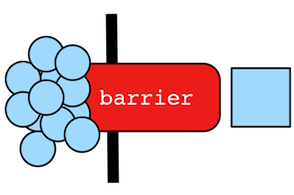Gremlin is primarily a lazy, stream processing language. This means that Gremlin fully processes (to the best of its abilities) any traversers currently in the traversal pipeline before getting more data from the start/head of the traversal. However, there are numerous situations in which a completely lazy computation is not possible (or impractical). When a computation is not lazy, a "barrier step" exists. There are three types of barriers:

1. `CollectingBarrierStep`: All of the traversers prior to the step are put into a collection and then processed in some way (e.g. ordered) prior to the collection being "drained" one-by-one to the next step. Examples include: `order()`, `sample()`, `aggregate()`, `barrier()`.

2. `ReducingBarrierStep`: All of the traversers prior to the step are processed by a reduce function and once all the previous traversers are processed, a single "reduced value" traverser is emitted to the next step. Note that the path history leading up to a reducing barrier step is destroyed given its many-to-one nature. Examples include: `fold()`, `count()`, `sum()`, `max()`, `min()`.

3. `SupplyingBarrierStep`: All of the traversers prior to the step are iterated (no processing) and then some provided supplier yields a single traverser to continue to the next step. Examples include: `cap()`.

In Gremlin OLAP (see `TraversalVertexProgram`), a barrier is introduced at the end of every adjacent vertex step. This means that the traversal does its best to compute as much as possible at the current, local vertex. What it can’t compute without referencing an adjacent vertex is aggregated into a barrier collection. When there are no more traversers at the local vertex, the barriered traversers are the messages that are propagated to remote vertices for further processing.

## A Note on Scopes

The `Scope` enum has two constants: `Scope.local` and `Scope.global`. Scope determines whether the particular step being scoped is with respects to the current object (`local`) at that step or to the entire stream of objects up to that step (`global`).

 Python The term `global` is a reserved word in Python, and therefore a `Scope` using that term must be referred as `global_`.
``````gremlin> g.V().has('name','marko').out('knows').count() //1\
==>2
gremlin> g.V().has('name','marko').out('knows').fold().count() //2\
==>1
gremlin> g.V().has('name','marko').out('knows').fold().count(local) //3\
==>2
gremlin> g.V().has('name','marko').out('knows').fold().count(global) //4\
==>1``````
``````g.V().has('name','marko').out('knows').count() //1\
g.V().has('name','marko').out('knows').fold().count() //2\
g.V().has('name','marko').out('knows').fold().count(local) //3\
g.V().has('name','marko').out('knows').fold().count(global) //4``````
1. Marko knows 2 people.

2. A list of Marko’s friends is created and thus, one object is counted (the single list).

3. A list of Marko’s friends is created and a `local`-count yields the number of objects in that list.

4. `count(global)` is the same as `count()` as the default behavior for most scoped steps is `global`.

The steps that support scoping are:

A few more examples of the use of `Scope` are provided below:

``````gremlin> g.V().both().group().by(label).select('software').dedup(local)
==>[v,v]
gremlin> g.V().groupCount().by(label).select(values).min(local)
==>2
gremlin> g.V().groupCount().by(label).order(local).by(values,desc)
==>[person:4,software:2]
gremlin> g.V().fold().sample(local,2)
==>[v,v]``````
``````g.V().both().group().by(label).select('software').dedup(local)
g.V().groupCount().by(label).select(values).min(local)
g.V().groupCount().by(label).order(local).by(values,desc)
g.V().fold().sample(local,2)``````

Finally, note that `local()`-step is a "hard-scoped step" that transforms any internal traversal into a locally-scoped operation. A contrived example is provided below:

``````gremlin> g.V().fold().local(unfold().count())
==>6
gremlin> g.V().fold().count(local)
==>6``````
``````g.V().fold().local(unfold().count())
g.V().fold().count(local)``````

## A Note On Lambdas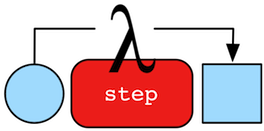A lambda is a function that can be referenced by software and thus, passed around like any other piece of data. In Gremlin, lambdas make it possible to generalize the behavior of a step such that custom steps can be created (on-the-fly) by the user. However, it is advised to avoid using lambdas if possible.

``````gremlin> g.V().filter{it.get().value('name') == 'marko'}.
flatMap{it.get().vertices(OUT,'created')}.
map {it.get().value('name')} //1\
==>lop
gremlin> g.V().has('name','marko').out('created').values('name') //2\
==>lop``````
``````g.V().filter{it.get().value('name') == 'marko'}.
flatMap{it.get().vertices(OUT,'created')}.
map {it.get().value('name')} //1\
g.V().has('name','marko').out('created').values('name') //2``````
1. A lambda-rich Gremlin traversal which should and can be avoided. (bad)

2. The same traversal (result), but without using lambdas. (good)

Gremlin attempts to provide the user a comprehensive collection of steps in the hopes that the user will never need to leverage a lambda in practice. It is advised that users only leverage a lambda if and only if there is no corresponding lambda-less step that encompasses the desired functionality. The reason being, lambdas can not be optimized by Gremlin’s compiler strategies as they can not be programmatically inspected (see traversal strategies). It is also not currently possible to send a lambda for remote execution to Gremlin-Server or a driver that supports remote execution.

In many situations where a lambda could be used, either a corresponding step exists or a traversal can be provided in its place. A `TraversalLambda` behaves like a typical lambda, but it can be optimized and it yields less objects than the corresponding pure-lambda form.

``````gremlin> g.V().out().out().path().by {it.value('name')}.
by {it.value('name')}.
by {g.V(it).in('created').values('name').fold().next()} //1\
==>[marko,josh,[josh]]
==>[marko,josh,[marko,josh,peter]]
gremlin> g.V().out().out().path().by('name').
by('name').
by(__.in('created').values('name').fold()) //2\
==>[marko,josh,[josh]]
==>[marko,josh,[marko,josh,peter]]``````
``````g.V().out().out().path().by {it.value('name')}.
by {it.value('name')}.
by {g.V(it).in('created').values('name').fold().next()} //1\
g.V().out().out().path().by('name').
by('name').
by(__.in('created').values('name').fold()) //2``````
1. The length-3 paths have each of their objects transformed by a lambda. (bad)

2. The length-3 paths have their objects transformed by a lambda-less step and a traversal lambda. (good)

## TraversalStrategyA `TraversalStrategy` analyzes a `Traversal` and, if the traversal meets its criteria, can mutate it accordingly. Traversal strategies are executed at compile-time and form the foundation of the Gremlin traversal machine’s compiler. There are 5 categories of strategies which are itemized below:

• There is an application-level feature that can be embedded into the traversal logic (decoration).

• There is a more efficient way to express the traversal at the TinkerPop3 level (optimization).

• There is a more efficient way to express the traversal at the graph system/language/driver level (provider optimization).

• There are some final adjustments/cleanups/analyses required before executing the traversal (finalization).

• There are certain traversals that are not legal for the application or traversal engine (verification).

 Note The `explain()`-step shows the user how each registered strategy mutates the traversal.

A simple `OptimizationStrategy` is the `IdentityRemovalStrategy`.

``````public final class IdentityRemovalStrategy extends AbstractTraversalStrategy<TraversalStrategy.OptimizationStrategy> implements TraversalStrategy.OptimizationStrategy {

private static final IdentityRemovalStrategy INSTANCE = new IdentityRemovalStrategy();

private IdentityRemovalStrategy() {
}

@Override
public void apply(Traversal.Admin<?, ?> traversal) {
if (traversal.getSteps().size() <= 1)
return;

for (IdentityStep<?> identityStep : TraversalHelper.getStepsOfClass(IdentityStep.class, traversal)) {
if (identityStep.getLabels().isEmpty() || !(identityStep.getPreviousStep() instanceof EmptyStep)) {
TraversalHelper.copyLabels(identityStep, identityStep.getPreviousStep(), false);
traversal.removeStep(identityStep);
}
}
}

public static IdentityRemovalStrategy instance() {
return INSTANCE;
}
}``````

This strategy simply removes any `IdentityStep` steps in the Traversal as `aStep().identity().identity().bStep()` is equivalent to `aStep().bStep()`. For those traversal strategies that require other strategies to execute prior or post to the strategy, then the following two methods can be defined in `TraversalStrategy` (with defaults being an empty set). If the `TraversalStrategy` is in a particular traversal category (i.e. decoration, optimization, provider-optimization, finalization, or verification), then priors and posts are only possible within the respective category.

``````public Set<Class<? extends S>> applyPrior();
public Set<Class<? extends S>> applyPost();``````
 Important `TraversalStrategy` categories are sorted within their category and the categories are then executed in the following order: decoration, optimization, provider optimization, finalization, and verification. If a designed strategy does not fit cleanly into these categories, then it can implement `TraversalStrategy` and its prior and posts can reference strategies within any category. However, such generalization are strongly discouraged.

An example of a `GraphSystemOptimizationStrategy` is provided below.

``g.V().has('name','marko')``

The expression above can be executed in a `O(|V|)` or `O(log(|V|)` fashion in TinkerGraph depending on whether there is or is not an index defined for "name."

``````public final class TinkerGraphStepStrategy extends AbstractTraversalStrategy<TraversalStrategy.ProviderOptimizationStrategy> implements TraversalStrategy.ProviderOptimizationStrategy {

private static final TinkerGraphStepStrategy INSTANCE = new TinkerGraphStepStrategy();

private TinkerGraphStepStrategy() {
}

@Override
public void apply(Traversal.Admin<?, ?> traversal) {
if (TraversalHelper.onGraphComputer(traversal))
return;

for (GraphStep originalGraphStep : TraversalHelper.getStepsOfClass(GraphStep.class, traversal)) {
TinkerGraphStep<?, ?> tinkerGraphStep = new TinkerGraphStep<>(originalGraphStep);
TraversalHelper.replaceStep(originalGraphStep, tinkerGraphStep, traversal);
Step<?, ?> currentStep = tinkerGraphStep.getNextStep();
while (currentStep instanceof HasStep || currentStep instanceof NoOpBarrierStep) {
if (currentStep instanceof HasStep) {
for (HasContainer hasContainer : ((HasContainerHolder) currentStep).getHasContainers()) {
if (!GraphStep.processHasContainerIds(tinkerGraphStep, hasContainer))
}
TraversalHelper.copyLabels(currentStep, currentStep.getPreviousStep(), false);
traversal.removeStep(currentStep);
}
currentStep = currentStep.getNextStep();
}
}
}

public static TinkerGraphStepStrategy instance() {
return INSTANCE;
}
}``````

The traversal is redefined by simply taking a chain of `has()`-steps after `g.V()` (`TinkerGraphStep`) and providing their `HasContainers` to `TinkerGraphStep`. Then its up to `TinkerGraphStep` to determine if an appropriate index exists. Given that the strategy uses non-TinkerPop3 provided steps, it should go into the `ProviderOptimizationStrategy` category to ensure the added step does not interfere with the assumptions of the `OptimizationStrategy` strategies.

``````gremlin> t = g.V().has('name','marko'); null
gremlin> t.toString()
==>[GraphStep(vertex,[]), HasStep([name.eq(marko)])]
gremlin> t.iterate(); null
gremlin> t.toString()
==>[TinkerGraphStep(vertex,[name.eq(marko)]), NoneStep]``````
``````t = g.V().has('name','marko'); null
t.toString()
t.iterate(); null
t.toString()``````
 Warning The reason that `OptimizationStrategy` and `ProviderOptimizationStrategy` are two different categories is that optimization strategies should only rewrite the traversal using TinkerPop3 steps. This ensures that the optimizations executed at the end of the optimization strategy round are TinkerPop3 compliant. From there, provider optimizations can analyze the traversal and rewrite the traversal as desired using graph system specific steps (e.g. replacing `GraphStep.HasStep…​HasStep` with `TinkerGraphStep`). If provider optimizations use graph system specific steps and implement `OptimizationStrategy`, then other TinkerPop3 optimizations may fail to optimize the traversal or mis-understand the graph system specific step behaviors (e.g. `ProviderVertexStep extends VertexStep`) and yield incorrect semantics.

Finally, here is a complicated traversal that has various components that are optimized by the default TinkerPop strategies.

``````gremlin> g.V().hasLabel('person'). //1\
and(has('name'), //2\
has('name','marko'),
filter(has('age',gt(20)))). //3\
match(__.as('a').has('age',lt(32)), //4\
__.as('a').repeat(outE().inV()).times(2).as('b')). //5\
where('a',neq('b')). //6\
where(__.as('b').both().count().is(gt(1))). //7\
select('b'). //8\
groupCount().
by(out().count()). //9\
explain()
==>Traversal Explanation
================================================================================================================================================================================================================================================
Original Traversal                 [GraphStep(vertex,[]), HasStep([~label.eq(person)]), AndStep([[TraversalFilterStep([PropertiesStep([name],value)])], [HasStep([name.eq(marko)])], [TraversalFilterStep([HasStep([age.gt(20)])])]]), MatchS
tep(AND,[[MatchStartStep(a), HasStep([age.lt(32)]), MatchEndStep], [MatchStartStep(a), RepeatStep([VertexStep(OUT,edge), EdgeVertexStep(IN), RepeatEndStep],until(loops(2)),emit(false)), MatchEndStep(b)]
]), WherePredicateStep(a,neq(b)), WhereTraversalStep([WhereStartStep(b), VertexStep(BOTH,vertex), CountGlobalStep, IsStep(gt(1))]), SelectOneStep(last,b), GroupCountStep([VertexStep(OUT,vertex), CountGl
obalStep])]

ConnectiveStrategy           [D]   [GraphStep(vertex,[]), HasStep([~label.eq(person)]), AndStep([[TraversalFilterStep([PropertiesStep([name],value)])], [HasStep([name.eq(marko)])], [TraversalFilterStep([HasStep([age.gt(20)])])]]), MatchS
tep(AND,[[MatchStartStep(a), HasStep([age.lt(32)]), MatchEndStep], [MatchStartStep(a), RepeatStep([VertexStep(OUT,edge), EdgeVertexStep(IN), RepeatEndStep],until(loops(2)),emit(false)), MatchEndStep(b)]
]), WherePredicateStep(a,neq(b)), WhereTraversalStep([WhereStartStep(b), VertexStep(BOTH,vertex), CountGlobalStep, IsStep(gt(1))]), SelectOneStep(last,b), GroupCountStep([VertexStep(OUT,vertex), CountGl
obalStep])]
EarlyLimitStrategy           [O]   [GraphStep(vertex,[]), HasStep([~label.eq(person)]), AndStep([[TraversalFilterStep([PropertiesStep([name],value)])], [HasStep([name.eq(marko)])], [TraversalFilterStep([HasStep([age.gt(20)])])]]), MatchS
tep(AND,[[MatchStartStep(a), HasStep([age.lt(32)]), MatchEndStep], [MatchStartStep(a), RepeatStep([VertexStep(OUT,edge), EdgeVertexStep(IN), RepeatEndStep],until(loops(2)),emit(false)), MatchEndStep(b)]
]), WherePredicateStep(a,neq(b)), WhereTraversalStep([WhereStartStep(b), VertexStep(BOTH,vertex), CountGlobalStep, IsStep(gt(1))]), SelectOneStep(last,b), GroupCountStep([VertexStep(OUT,vertex), CountGl
obalStep])]
MatchPredicateStrategy       [O]   [GraphStep(vertex,[]), HasStep([~label.eq(person)]), AndStep([[TraversalFilterStep([PropertiesStep([name],value)])], [HasStep([name.eq(marko)])], [TraversalFilterStep([HasStep([age.gt(20)])])]]), MatchS
tep(AND,[[MatchStartStep(a), HasStep([age.lt(32)]), MatchEndStep], [MatchStartStep(a), RepeatStep([VertexStep(OUT,edge), EdgeVertexStep(IN), RepeatEndStep],until(loops(2)),emit(false)), MatchEndStep(b)]
, [MatchStartStep(a), WherePredicateStep(neq(b)), MatchEndStep], [MatchStartStep(b), WhereTraversalStep([WhereStartStep, VertexStep(BOTH,vertex), CountGlobalStep, IsStep(gt(1))]), MatchEndStep]]), Selec
tOneStep(last,b), GroupCountStep([VertexStep(OUT,vertex), CountGlobalStep])]
FilterRankingStrategy        [O]   [GraphStep(vertex,[]), HasStep([~label.eq(person)]), AndStep([[TraversalFilterStep([PropertiesStep([name],value)])], [HasStep([name.eq(marko)])], [TraversalFilterStep([HasStep([age.gt(20)])])]]), MatchS
tep(AND,[[MatchStartStep(a), HasStep([age.lt(32)]), MatchEndStep], [MatchStartStep(a), RepeatStep([VertexStep(OUT,edge), EdgeVertexStep(IN), RepeatEndStep],until(loops(2)),emit(false)), MatchEndStep(b)]
, [MatchStartStep(a), WherePredicateStep(neq(b)), MatchEndStep], [MatchStartStep(b), WhereTraversalStep([WhereStartStep, VertexStep(BOTH,vertex), CountGlobalStep, IsStep(gt(1))]), MatchEndStep]]), Selec
tOneStep(last,b), GroupCountStep([VertexStep(OUT,vertex), CountGlobalStep])]
InlineFilterStrategy         [O]   [GraphStep(vertex,[]), HasStep([~label.eq(person)]), TraversalFilterStep([PropertiesStep([name],value)]), HasStep([name.eq(marko), age.gt(20), age.lt(32)])@[a], MatchStep(AND,[[MatchStartStep(a), Repeat
Step([VertexStep(OUT,edge), EdgeVertexStep(IN), RepeatEndStep],until(loops(2)),emit(false)), MatchEndStep(b)], [MatchStartStep(a), WherePredicateStep(neq(b)), MatchEndStep], [MatchStartStep(b), WhereTra
versalStep([WhereStartStep, VertexStep(BOTH,vertex), CountGlobalStep, IsStep(gt(1))]), MatchEndStep]]), SelectOneStep(last,b), GroupCountStep([VertexStep(OUT,vertex), CountGlobalStep])]
IncidentToAdjacentStrategy   [O]   [GraphStep(vertex,[]), HasStep([~label.eq(person)]), TraversalFilterStep([PropertiesStep([name],value)]), HasStep([name.eq(marko), age.gt(20), age.lt(32)])@[a], MatchStep(AND,[[MatchStartStep(a), Repeat
Step([VertexStep(OUT,vertex), RepeatEndStep],until(loops(2)),emit(false)), MatchEndStep(b)], [MatchStartStep(a), WherePredicateStep(neq(b)), MatchEndStep], [MatchStartStep(b), WhereTraversalStep([WhereS
tartStep, VertexStep(BOTH,vertex), CountGlobalStep, IsStep(gt(1))]), MatchEndStep]]), SelectOneStep(last,b), GroupCountStep([VertexStep(OUT,vertex), CountGlobalStep])]
AdjacentToIncidentStrategy   [O]   [GraphStep(vertex,[]), HasStep([~label.eq(person)]), TraversalFilterStep([PropertiesStep([name],property)]), HasStep([name.eq(marko), age.gt(20), age.lt(32)])@[a], MatchStep(AND,[[MatchStartStep(a), Rep
eatStep([VertexStep(OUT,vertex), RepeatEndStep],until(loops(2)),emit(false)), MatchEndStep(b)], [MatchStartStep(a), WherePredicateStep(neq(b)), MatchEndStep], [MatchStartStep(b), WhereTraversalStep([Whe
reStartStep, VertexStep(BOTH,edge), CountGlobalStep, IsStep(gt(1))]), MatchEndStep]]), SelectOneStep(last,b), GroupCountStep([VertexStep(OUT,edge), CountGlobalStep])]
RepeatUnrollStrategy         [O]   [GraphStep(vertex,[]), HasStep([~label.eq(person)]), TraversalFilterStep([PropertiesStep([name],property)]), HasStep([name.eq(marko), age.gt(20), age.lt(32)])@[a], MatchStep(AND,[[MatchStartStep(a), Ver
texStep(OUT,vertex), NoOpBarrierStep(2500), VertexStep(OUT,vertex), NoOpBarrierStep(2500), MatchEndStep(b)], [MatchStartStep(a), WherePredicateStep(neq(b)), MatchEndStep], [MatchStartStep(b), WhereTrave
rsalStep([WhereStartStep, VertexStep(BOTH,edge), CountGlobalStep, IsStep(gt(1))]), MatchEndStep]]), SelectOneStep(last,b), GroupCountStep([VertexStep(OUT,edge), CountGlobalStep])]
CountStrategy                [O]   [GraphStep(vertex,[]), HasStep([~label.eq(person)]), TraversalFilterStep([PropertiesStep([name],property)]), HasStep([name.eq(marko), age.gt(20), age.lt(32)])@[a], MatchStep(AND,[[MatchStartStep(a), Ver
texStep(OUT,vertex), NoOpBarrierStep(2500), VertexStep(OUT,vertex), NoOpBarrierStep(2500), MatchEndStep(b)], [MatchStartStep(a), WherePredicateStep(neq(b)), MatchEndStep], [MatchStartStep(b), WhereTrave
rsalStep([WhereStartStep, VertexStep(BOTH,edge), RangeGlobalStep(0,2), CountGlobalStep, IsStep(gt(1))]), MatchEndStep]]), SelectOneStep(last,b), GroupCountStep([VertexStep(OUT,edge), CountGlobalStep])]
PathRetractionStrategy       [O]   [GraphStep(vertex,[]), HasStep([~label.eq(person)]), TraversalFilterStep([PropertiesStep([name],property)]), HasStep([name.eq(marko), age.gt(20), age.lt(32)])@[a], MatchStep(AND,[[MatchStartStep(a), Ver
texStep(OUT,vertex), NoOpBarrierStep(2500), VertexStep(OUT,vertex), NoOpBarrierStep(2500), MatchEndStep(b)], [MatchStartStep(a), WherePredicateStep(neq(b)), MatchEndStep], [MatchStartStep(b), WhereTrave
rsalStep([WhereStartStep, VertexStep(BOTH,edge), RangeGlobalStep(0,2), CountGlobalStep, IsStep(gt(1))]), MatchEndStep]]), SelectOneStep(last,b), GroupCountStep([VertexStep(OUT,edge), CountGlobalStep])]
LazyBarrierStrategy          [O]   [GraphStep(vertex,[]), HasStep([~label.eq(person)]), TraversalFilterStep([PropertiesStep([name],property)]), HasStep([name.eq(marko), age.gt(20), age.lt(32)])@[a], MatchStep(AND,[[MatchStartStep(a), Ver
texStep(OUT,vertex), NoOpBarrierStep(2500), VertexStep(OUT,vertex), NoOpBarrierStep(2500), MatchEndStep(b)], [MatchStartStep(a), WherePredicateStep(neq(b)), MatchEndStep], [MatchStartStep(b), WhereTrave
rsalStep([WhereStartStep, VertexStep(BOTH,edge), RangeGlobalStep(0,2), CountGlobalStep, IsStep(gt(1))]), MatchEndStep]]), SelectOneStep(last,b), GroupCountStep([VertexStep(OUT,edge), CountGlobalStep])]
TinkerGraphCountStrategy     [P]   [GraphStep(vertex,[]), HasStep([~label.eq(person)]), TraversalFilterStep([PropertiesStep([name],property)]), HasStep([name.eq(marko), age.gt(20), age.lt(32)])@[a], MatchStep(AND,[[MatchStartStep(a), Ver
texStep(OUT,vertex), NoOpBarrierStep(2500), VertexStep(OUT,vertex), NoOpBarrierStep(2500), MatchEndStep(b)], [MatchStartStep(a), WherePredicateStep(neq(b)), MatchEndStep], [MatchStartStep(b), WhereTrave
rsalStep([WhereStartStep, VertexStep(BOTH,edge), RangeGlobalStep(0,2), CountGlobalStep, IsStep(gt(1))]), MatchEndStep]]), SelectOneStep(last,b), GroupCountStep([VertexStep(OUT,edge), CountGlobalStep])]
TinkerGraphStepStrategy      [P]   [TinkerGraphStep(vertex,[~label.eq(person)]), TraversalFilterStep([PropertiesStep([name],property)]), HasStep([name.eq(marko), age.gt(20), age.lt(32)])@[a], MatchStep(AND,[[MatchStartStep(a), VertexStep
(OUT,vertex), NoOpBarrierStep(2500), VertexStep(OUT,vertex), NoOpBarrierStep(2500), MatchEndStep(b)], [MatchStartStep(a), WherePredicateStep(neq(b)), MatchEndStep], [MatchStartStep(b), WhereTraversalSte
p([WhereStartStep, VertexStep(BOTH,edge), RangeGlobalStep(0,2), CountGlobalStep, IsStep(gt(1))]), MatchEndStep]]), SelectOneStep(last,b), GroupCountStep([VertexStep(OUT,edge), CountGlobalStep])]
ProfileStrategy              [F]   [TinkerGraphStep(vertex,[~label.eq(person)]), TraversalFilterStep([PropertiesStep([name],property)]), HasStep([name.eq(marko), age.gt(20), age.lt(32)])@[a], MatchStep(AND,[[MatchStartStep(a), VertexStep
(OUT,vertex), NoOpBarrierStep(2500), VertexStep(OUT,vertex), NoOpBarrierStep(2500), MatchEndStep(b)], [MatchStartStep(a), WherePredicateStep(neq(b)), MatchEndStep], [MatchStartStep(b), WhereTraversalSte
p([WhereStartStep, VertexStep(BOTH,edge), RangeGlobalStep(0,2), CountGlobalStep, IsStep(gt(1))]), MatchEndStep]]), SelectOneStep(last,b), GroupCountStep([VertexStep(OUT,edge), CountGlobalStep])]
StandardVerificationStrategy [V]   [TinkerGraphStep(vertex,[~label.eq(person)]), TraversalFilterStep([PropertiesStep([name],property)]), HasStep([name.eq(marko), age.gt(20), age.lt(32)])@[a], MatchStep(AND,[[MatchStartStep(a), VertexStep
(OUT,vertex), NoOpBarrierStep(2500), VertexStep(OUT,vertex), NoOpBarrierStep(2500), MatchEndStep(b)], [MatchStartStep(a), WherePredicateStep(neq(b)), MatchEndStep], [MatchStartStep(b), WhereTraversalSte
p([WhereStartStep, VertexStep(BOTH,edge), RangeGlobalStep(0,2), CountGlobalStep, IsStep(gt(1))]), MatchEndStep]]), SelectOneStep(last,b), GroupCountStep([VertexStep(OUT,edge), CountGlobalStep])]

Final Traversal                    [TinkerGraphStep(vertex,[~label.eq(person)]), TraversalFilterStep([PropertiesStep([name],property)]), HasStep([name.eq(marko), age.gt(20), age.lt(32)])@[a], MatchStep(AND,[[MatchStartStep(a), VertexStep
(OUT,vertex), NoOpBarrierStep(2500), VertexStep(OUT,vertex), NoOpBarrierStep(2500), MatchEndStep(b)], [MatchStartStep(a), WherePredicateStep(neq(b)), MatchEndStep], [MatchStartStep(b), WhereTraversalSte
p([WhereStartStep, VertexStep(BOTH,edge), RangeGlobalStep(0,2), CountGlobalStep, IsStep(gt(1))]), MatchEndStep]]), SelectOneStep(last,b), GroupCountStep([VertexStep(OUT,edge), CountGlobalStep])]``````
``````g.V().hasLabel('person'). //1\
and(has('name'), //2\
has('name','marko'),
filter(has('age',gt(20)))). //3\
match(__.as('a').has('age',lt(32)), //4\
__.as('a').repeat(outE().inV()).times(2).as('b')). //5\
where('a',neq('b')). //6\
where(__.as('b').both().count().is(gt(1))). //7\
select('b'). //8\
groupCount().
by(out().count()). //9\
explain()``````
1. `TinkerGraphStepStrategy` pulls in `has()`-step predicates for global, graph-centric index lookups.

2. `FilterRankStrategy` sorts filter steps by their time/space execution costs.

3. `InlineFilterStrategy` de-nests filters to increase the likelihood of filter concatenation and aggregation.

4. `InlineFilterStrategy` pulls out named predicates from `match()`-step to more easily allow provider strategies to use indices.

5. `RepeatUnrollStrategy` will unroll loops and `IncidentToAdjacentStrategy` will turn `outE().inV()`-patterns into `out()`.

6. `MatchPredicateStrategy` will pull in `where()`-steps so that they can be subjected to `match()`-steps runtime query optimizer.

7. `CountStrategy` will limit the traversal to only the number of traversers required for the `count().is(x)`-check.

8. `PathRetractionStrategy` will remove paths from the traversers and increase the likelihood of bulking as path data is not required after `select('b')`.

9. `AdjacentToIncidentStrategy` will turn `out()` into `outE()` to increase data access locality.

A collection of useful `DecorationStrategy` strategies are provided with TinkerPop3 and are generally useful to end-users. The following sub-sections detail these strategies:

### ElementIdStrategy

`ElementIdStrategy` provides control over element identifiers. Some Graph implementations, such as TinkerGraph, allow specification of custom identifiers when creating elements:

``````gremlin> g = TinkerGraph.open().traversal()
==>graphtraversalsource[tinkergraph[vertices:0 edges:0], standard]
==>v[42a]
gremlin> g.V('42a')
==>v[42a]``````
``````g = TinkerGraph.open().traversal()
g.V('42a')``````

Other `Graph` implementations, such as Neo4j, generate element identifiers automatically and cannot be assigned. As a helper, `ElementIdStrategy` can be used to make identifier assignment possible by using vertex and edge indices under the hood.

``````gremlin> graph = Neo4jGraph.open('/tmp/neo4j')
==>neo4jgraph[community single [/tmp/neo4j]]
gremlin> strategy = ElementIdStrategy.build().create()
==>ElementIdStrategy
gremlin> g = graph.traversal().withStrategies(strategy)
==>graphtraversalsource[neo4jgraph[community single [/tmp/neo4j]], standard]
==>42a``````
``````graph = Neo4jGraph.open('/tmp/neo4j')
strategy = ElementIdStrategy.build().create()
g = graph.traversal().withStrategies(strategy)
 Important The key that is used to store the assigned identifier should be indexed in the underlying graph database. If it is not indexed, then lookups for the elements that use these identifiers will perform a linear scan.

### EventStrategy

The purpose of the `EventStrategy` is to raise events to one or more `MutationListener` objects as changes to the underlying `Graph` occur within a `Traversal`. Such a strategy is useful for logging changes, triggering certain actions based on change, or any application that needs notification of some mutating operation during a `Traversal`. If the transaction is rolled back, the event queue is reset.

The following events are raised to the `MutationListener`:

• New vertex

• New edge

• Vertex property changed

• Edge property changed

• Vertex property removed

• Edge property removed

• Vertex removed

• Edge removed

To start processing events from a `Traversal` first implement the `MutationListener` interface. An example of this implementation is the `ConsoleMutationListener` which writes output to the console for each event. The following console session displays the basic usage:

``````gremlin> import org.apache.tinkerpop.gremlin.process.traversal.step.util.event.*
gremlin> graph = TinkerFactory.createModern()
==>tinkergraph[vertices:6 edges:6]
gremlin> l = new ConsoleMutationListener(graph)
==>MutationListener[tinkergraph[vertices:6 edges:6]]
==>EventStrategy
gremlin> g = graph.traversal().withStrategies(strategy)
==>graphtraversalsource[tinkergraph[vertices:6 edges:6], standard]
Vertex [v] added to graph [tinkergraph[vertices:7 edges:6]]
==>v
gremlin> g.E().drop()
Edge [e[1-knows->2]] removed from graph [tinkergraph[vertices:7 edges:6]]
Edge [e[1-knows->4]] removed from graph [tinkergraph[vertices:7 edges:5]]
Edge [e[1-created->3]] removed from graph [tinkergraph[vertices:7 edges:4]]
Edge [e[4-created->5]] removed from graph [tinkergraph[vertices:7 edges:3]]
Edge [e[4-created->3]] removed from graph [tinkergraph[vertices:7 edges:2]]
Edge [e[6-created->3]] removed from graph [tinkergraph[vertices:7 edges:1]]``````
``````import org.apache.tinkerpop.gremlin.process.traversal.step.util.event.*
graph = TinkerFactory.createModern()
l = new ConsoleMutationListener(graph)
g = graph.traversal().withStrategies(strategy)
g.E().drop()``````

By default, the `EventStrategy` is configured with an `EventQueue` that raises events as they occur within execution of a `Step`. As such, the final line of Gremlin execution that drops all edges shows a bit of an inconsistent count, where the removed edge count is accounted for after the event is raised. The strategy can also be configured with a `TransactionalEventQueue` that captures the changes within a transaction and does not allow them to fire until the transaction is committed.

 Warning `EventStrategy` is not meant for usage in tracking global mutations across separate processes. In other words, a mutation in one JVM process is not raised as an event in a different JVM process. In addition, events are not raised when mutations occur outside of the `Traversal` context.

Another default configuration for `EventStrategy` revolves around the concept of "detachment". Graph elements are detached from the graph as copies when passed to referring mutation events. Therefore, when adding a new `Vertex` in TinkerGraph, the event will not contain a `TinkerVertex` but will instead include a `DetachedVertex`. This behavior can be modified with the `detach()` method on the `EventStrategy.Builder` which accepts the following inputs: `null` meaning no detachment and the return of the original element, `DetachedFactory` which is the same as the default behavior, and `ReferenceFactory` which will return "reference" elements only with no properties.

 Important If setting the `detach()` configuration to `null`, be aware that transactional graphs will likely create a new transaction immediately following the `commit()` that raises the events. The graph elements raised in the events may also not behave as "snapshots" at the time of their creation as they are "live" references to actual database elements.

### PartitionStrategy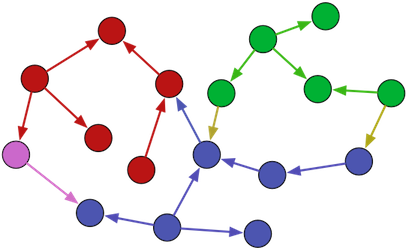`PartitionStrategy` partitions the vertices and edges of a graph into `String` named partitions (i.e. buckets, subgraphs, etc.). The idea behind `PartitionStrategy` is presented in the image above where each element is in a single partition (represented by its color). Partitions can be read from, written to, and linked/joined by edges that span one or two partitions (e.g. a tail vertex in one partition and a head vertex in another).

There are three primary configurations in `PartitionStrategy`:

1. Partition Key - The property key that denotes a String value representing a partition.

2. Write Partition - A `String` denoting what partition all future written elements will be in.

3. Read Partitions - A `Set<String>` of partitions that can be read from.

The best way to understand `PartitionStrategy` is via example.

``````gremlin> graph = TinkerFactory.createModern()
==>tinkergraph[vertices:6 edges:6]
==>PartitionStrategy
==>PartitionStrategy
gremlin> gA = graph.traversal().withStrategies(strategyA)
==>graphtraversalsource[tinkergraph[vertices:6 edges:6], standard]
gremlin> gA.addV() // this vertex has a property of {_partition:"a"}
==>v
gremlin> gB = graph.traversal().withStrategies(strategyB)
==>graphtraversalsource[tinkergraph[vertices:7 edges:6], standard]
gremlin> gB.addV() // this vertex has a property of {_partition:"b"}
==>v
gremlin> gA.V()
==>v
gremlin> gB.V()
==>v``````
``````graph = TinkerFactory.createModern()
gA = graph.traversal().withStrategies(strategyA)
gA.addV() // this vertex has a property of {_partition:"a"}
gB = graph.traversal().withStrategies(strategyB)
gB.addV() // this vertex has a property of {_partition:"b"}
gA.V()
gB.V()``````

Partitions may also extend to `VertexProperty` elements if the `Graph` can support meta-properties and if the `includeMetaProperties` value is set to `true` when the `PartitionStrategy` is built. The `partitionKey` will be stored in the meta-properties of the `VertexProperty` and blind the traversal to those properties. Please note that the `VertexProperty` will only be hidden by way of the `Traversal` itself. For example, calling `Vertex.property(k)` bypasses the context of the `PartitionStrategy` and will thus allow all properties to be accessed.

By writing elements to particular partitions and then restricting read partitions, the developer is able to create multiple graphs within a single address space. Moreover, by supporting references between partitions, it is possible to merge those multiple graphs (i.e. join partitions).

`ReadOnlyStrategy` is largely self-explanatory. A `Traversal` that has this strategy applied will throw an `IllegalStateException` if the `Traversal` has any mutating steps within it.

### SubgraphStrategy

`SubgraphStrategy` is similar to `PartitionStrategy` in that it constrains a `Traversal` to certain vertices, edges, and vertex properties as determined by a `Traversal`-based criterion defined individually for each.

``````gremlin> graph = TinkerFactory.createTheCrew()
==>tinkergraph[vertices:6 edges:14]
gremlin> g = graph.traversal()
==>graphtraversalsource[tinkergraph[vertices:6 edges:14], standard]
gremlin> g.V().as('a').values('location').as('b'). //1\
select('a','b').by('name').by()
==>[a:marko,b:san diego]
==>[a:marko,b:santa cruz]
==>[a:marko,b:brussels]
==>[a:marko,b:santa fe]
==>[a:stephen,b:centreville]
==>[a:stephen,b:dulles]
==>[a:stephen,b:purcellville]
==>[a:matthias,b:bremen]
==>[a:matthias,b:baltimore]
==>[a:matthias,b:oakland]
==>[a:matthias,b:seattle]
==>[a:daniel,b:spremberg]
==>[a:daniel,b:kaiserslautern]
==>[a:daniel,b:aachen]
gremlin> g = g.withStrategies(SubgraphStrategy.build().vertexProperties(hasNot('endTime')).create()) //2\
==>graphtraversalsource[tinkergraph[vertices:6 edges:14], standard]
gremlin> g.V().as('<``````